# Selina Solutions Concise Physics Class 10 Chapter 8 Current Electricity

Selina Solutions Concise Physics Class 10 Chapter 8 Current Electricity provides complete information regarding the concept of Current. These solutions are a single window with accurate answers to help students in their exam preparations. By going through Selina Solutions students come to understand the topics clearly, which they might have missed during class hours. Students aiming to perform well in the exams must practise these solutions diligently.

Selina Solutions Concise Physics Class 10 are prepared in accordance with the ICSE. These precise answers help students during exam preparation as the answers are well structured, according to their individual understanding capacities. Use of a simple language in Selina Solutions improves interest among students in learning the concepts covered under the chapter in a better way. Students can download the Selina Solutions Concise Physics Class 10 PDF free from the links available here.

## Selina Solutions Concise Physics Chapter 8 Current Electricity:-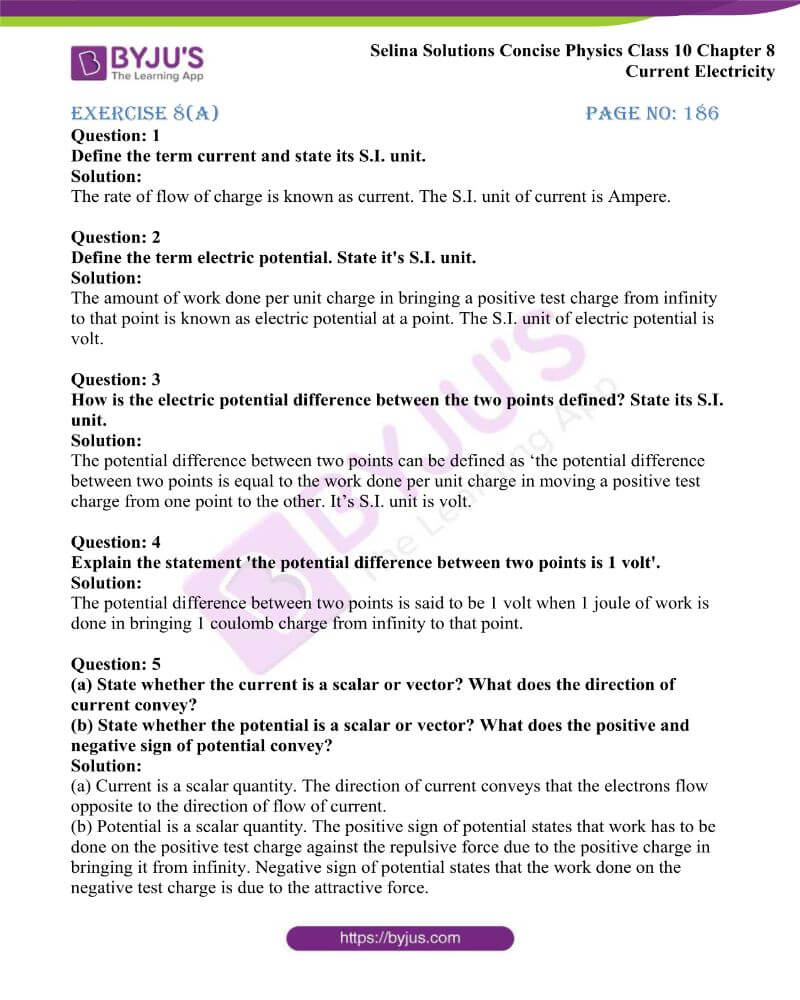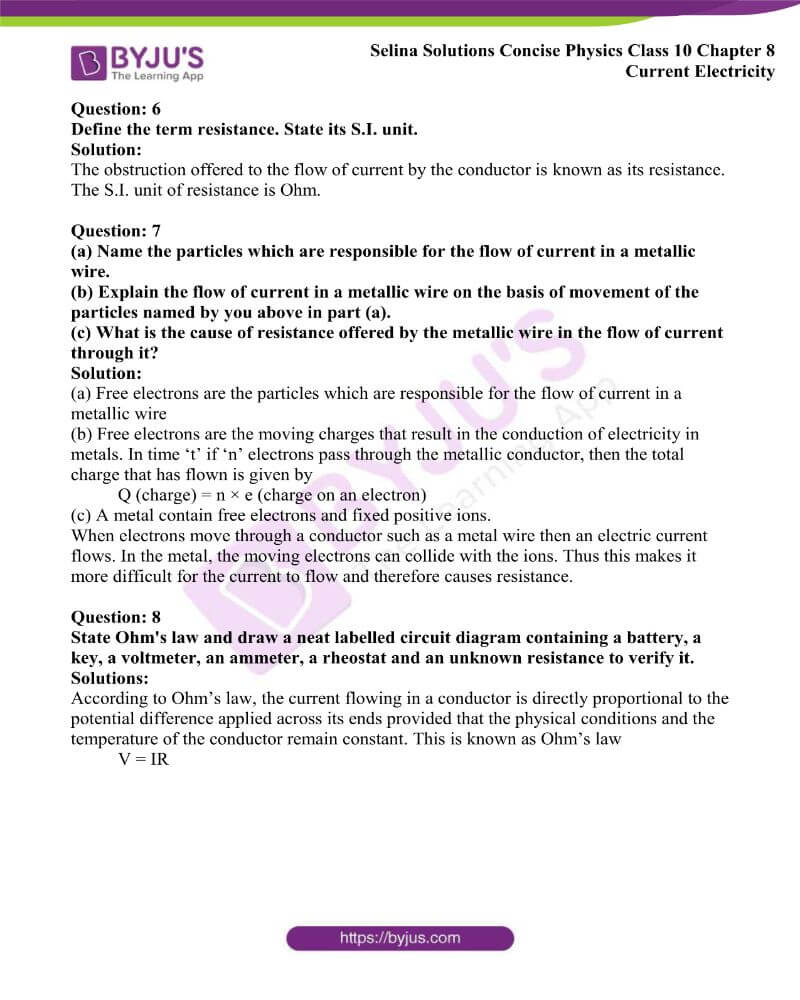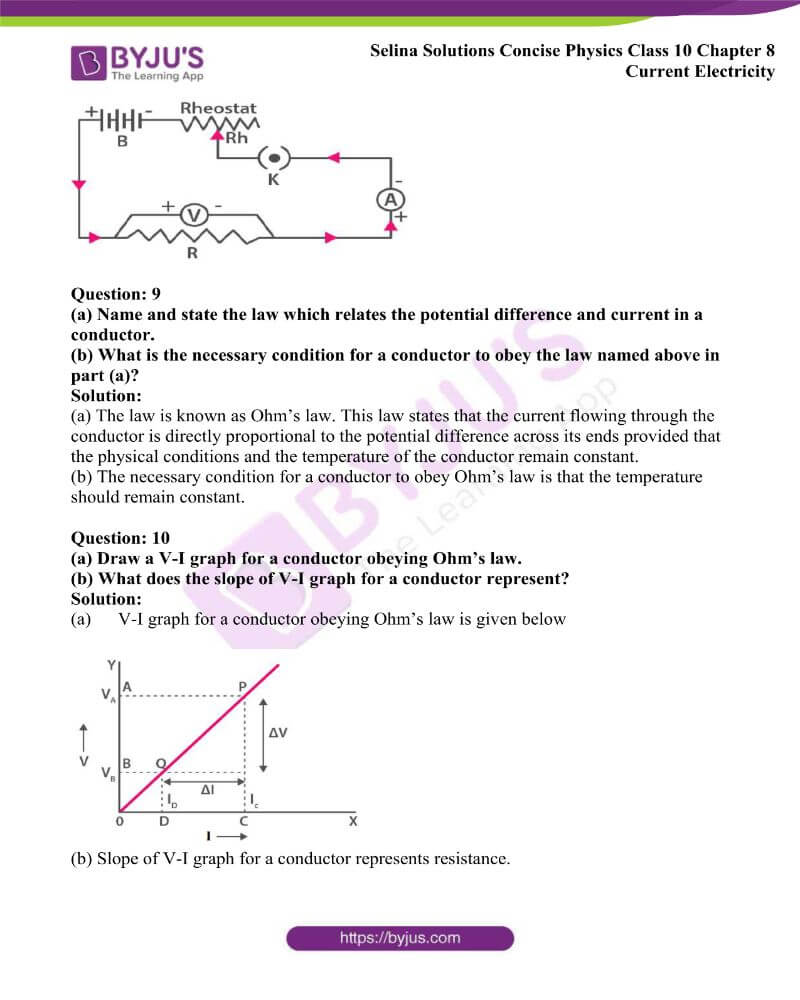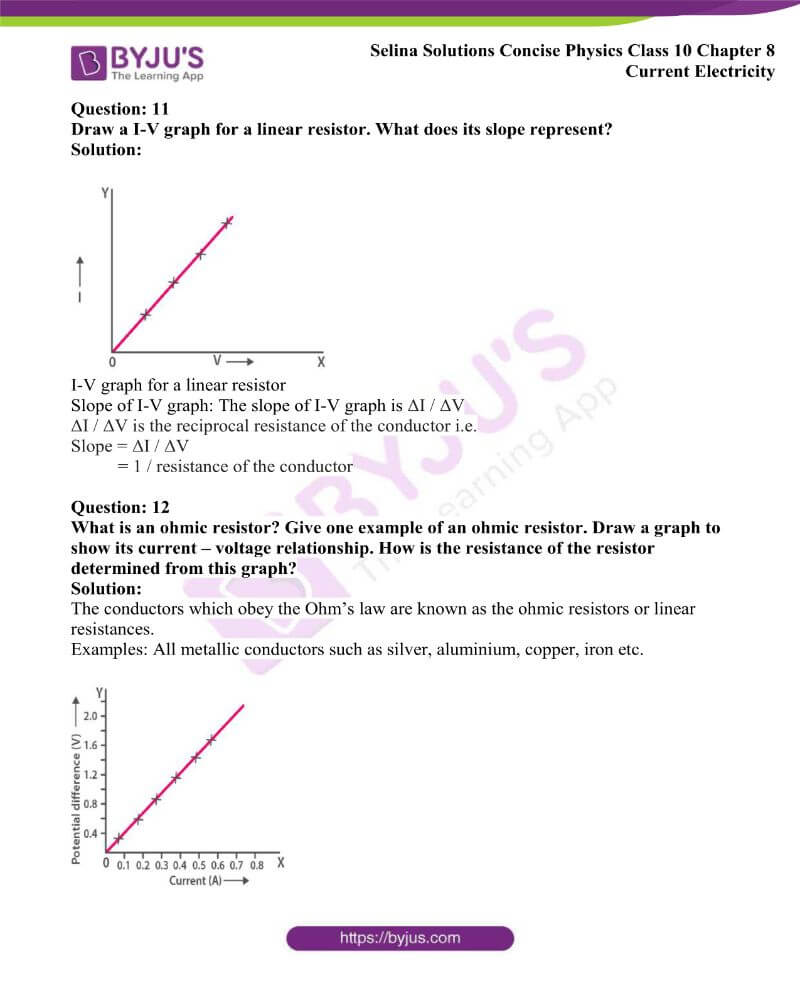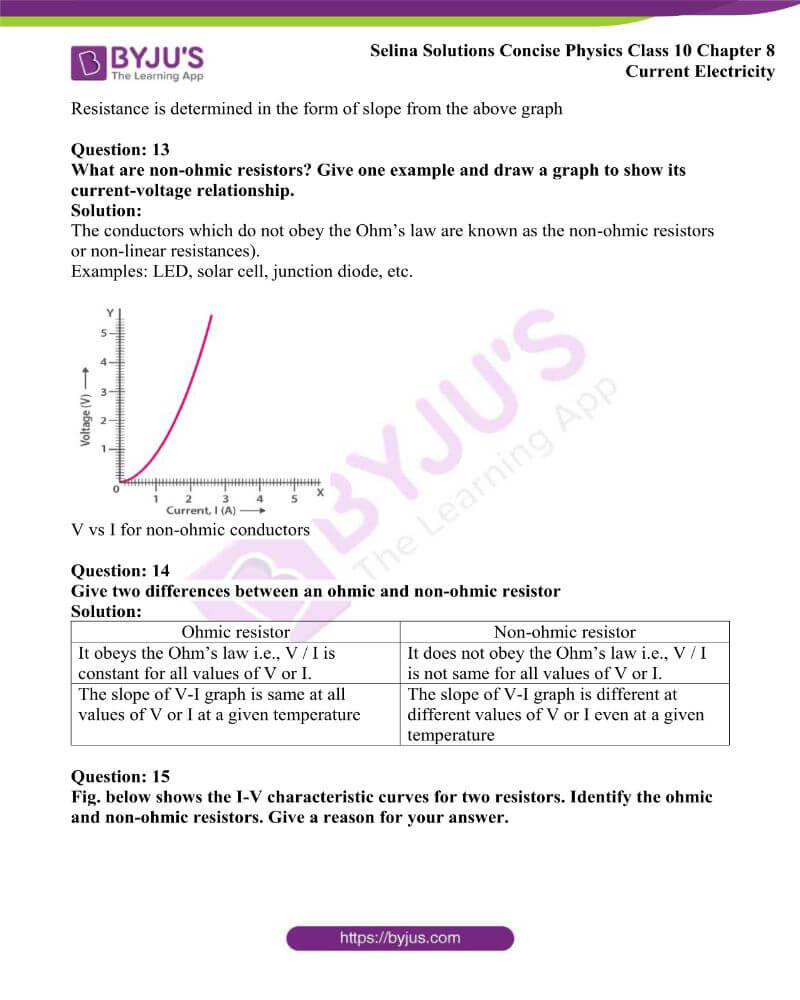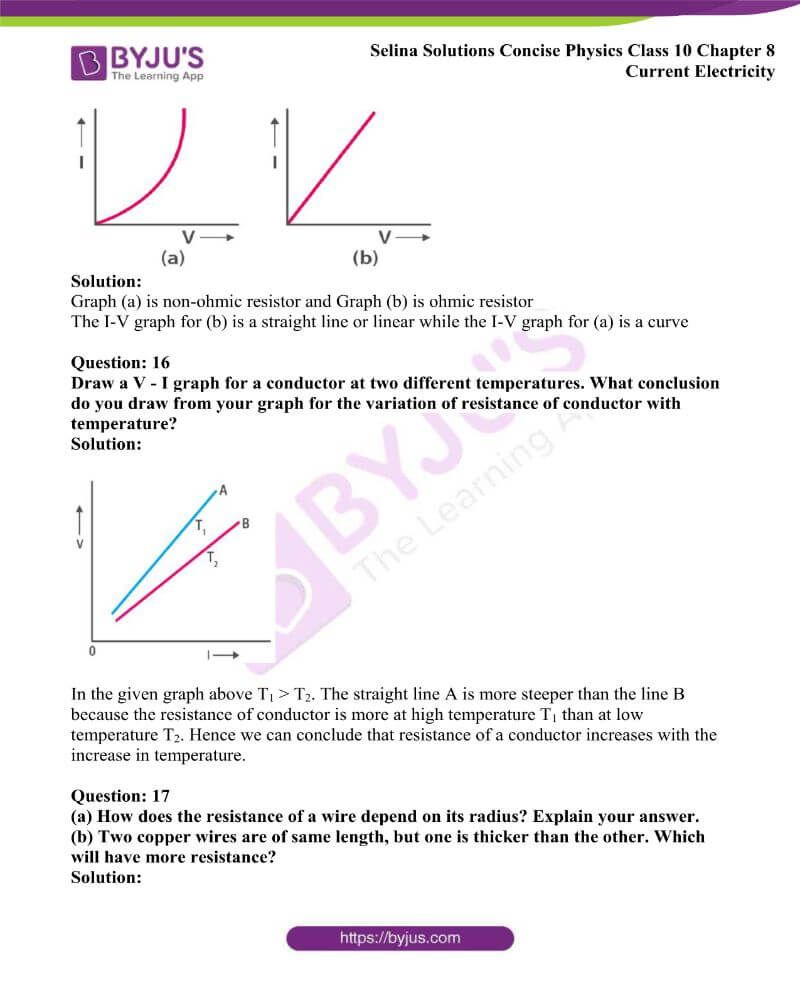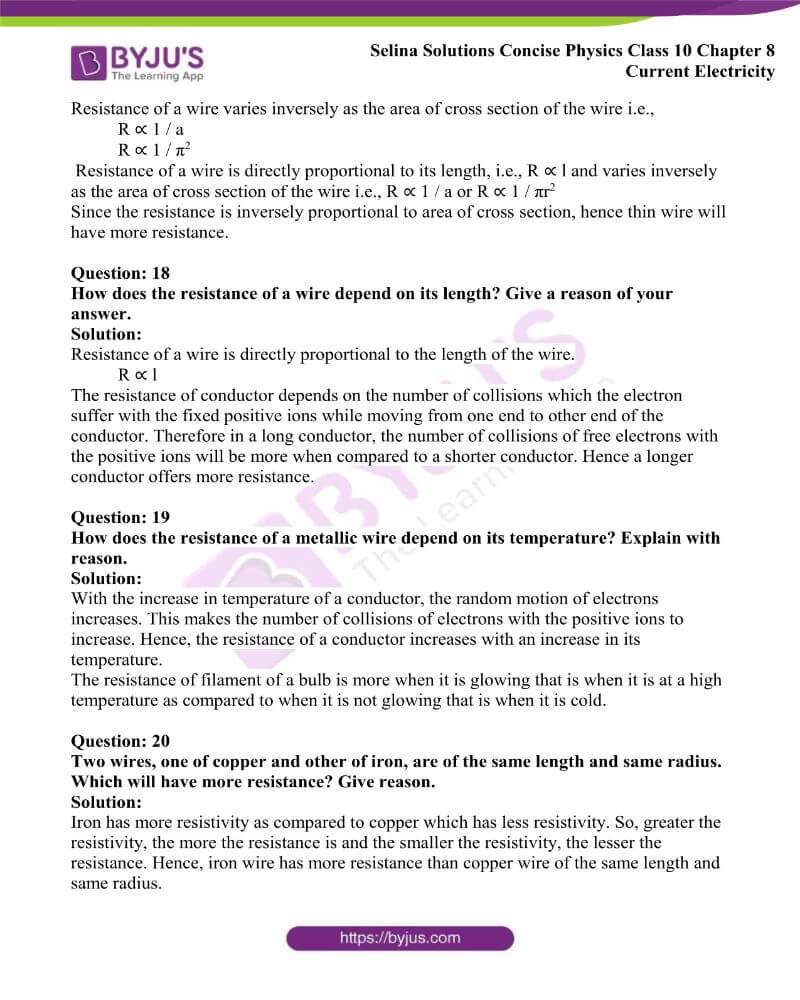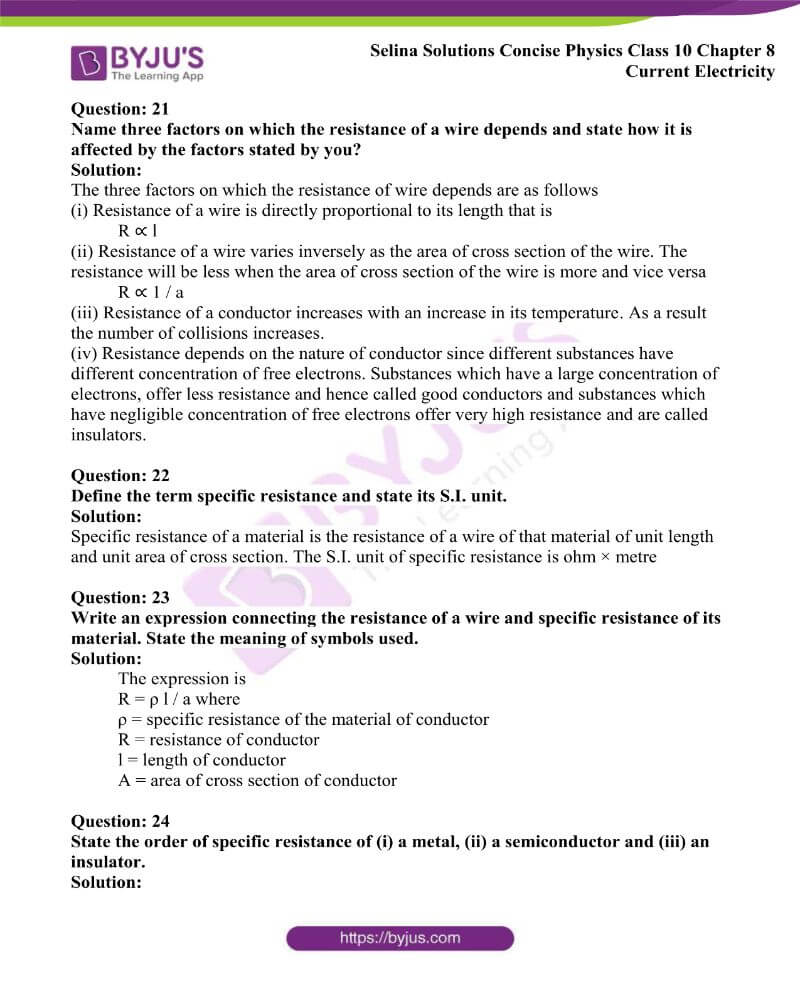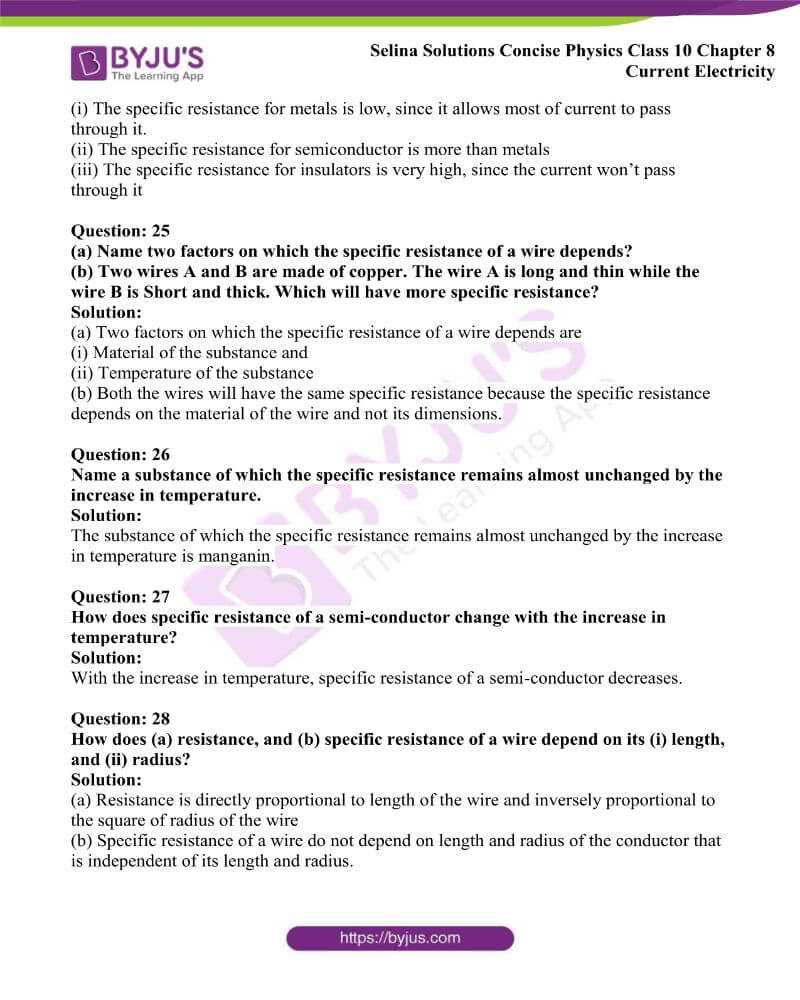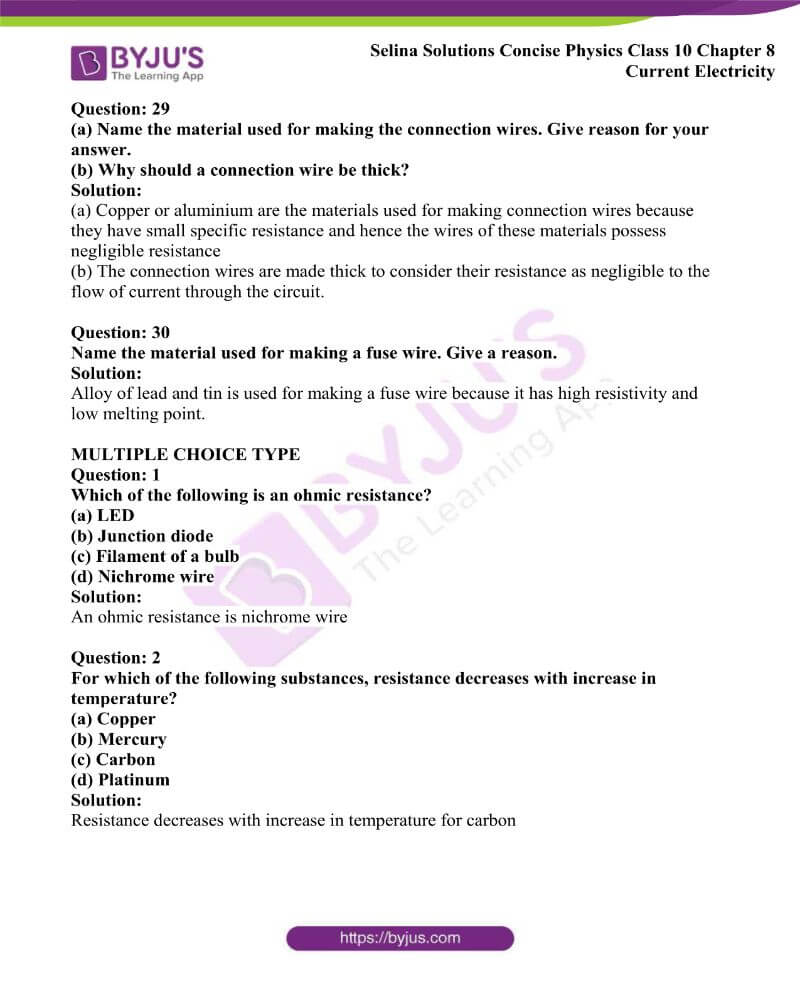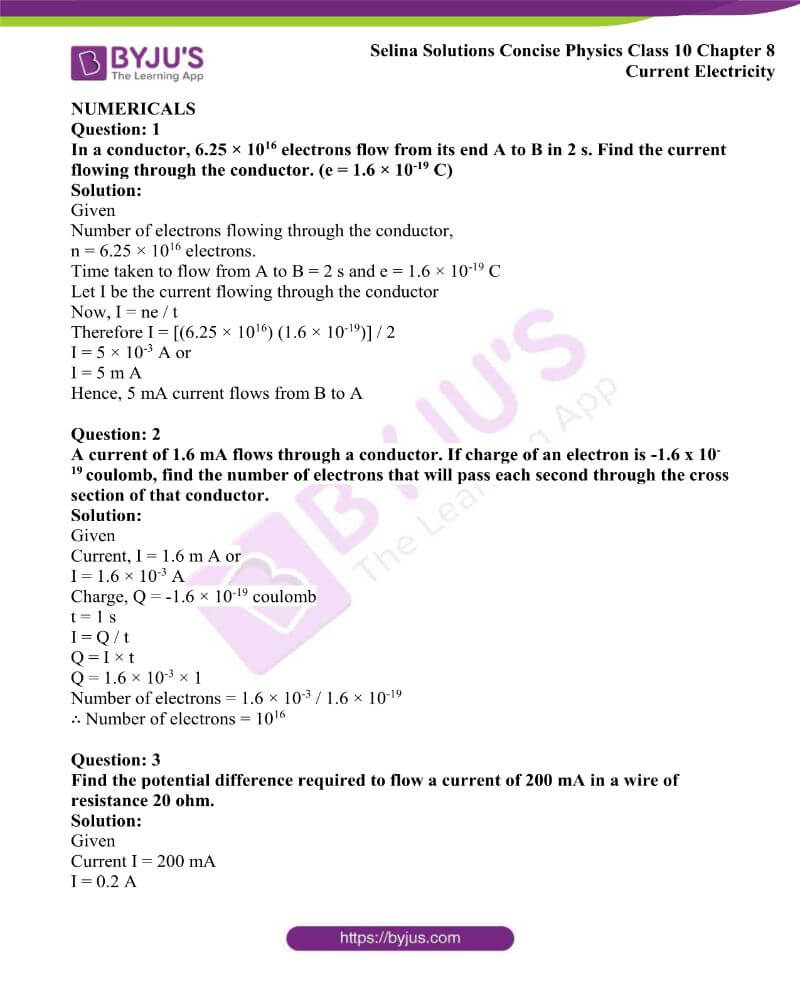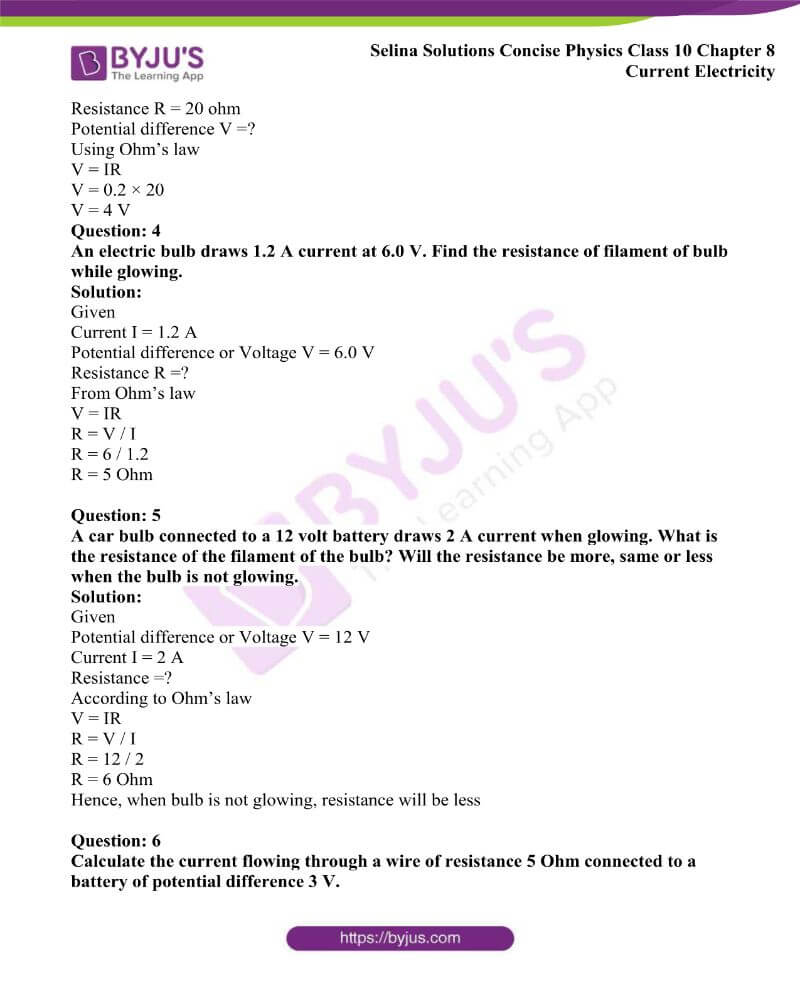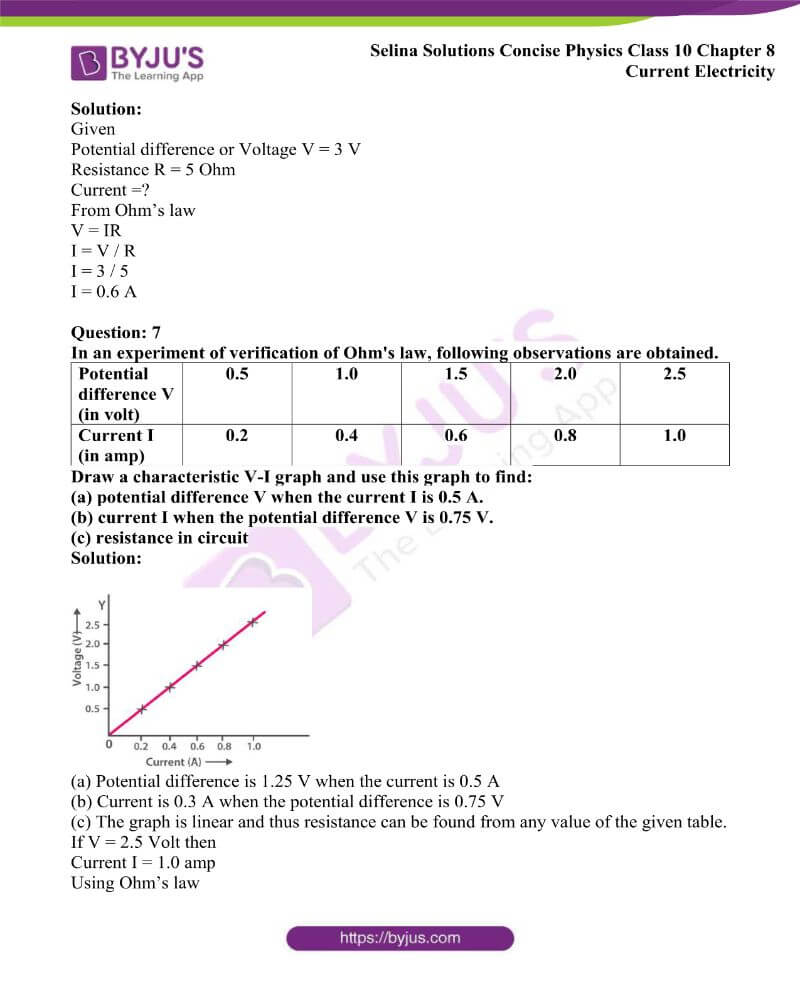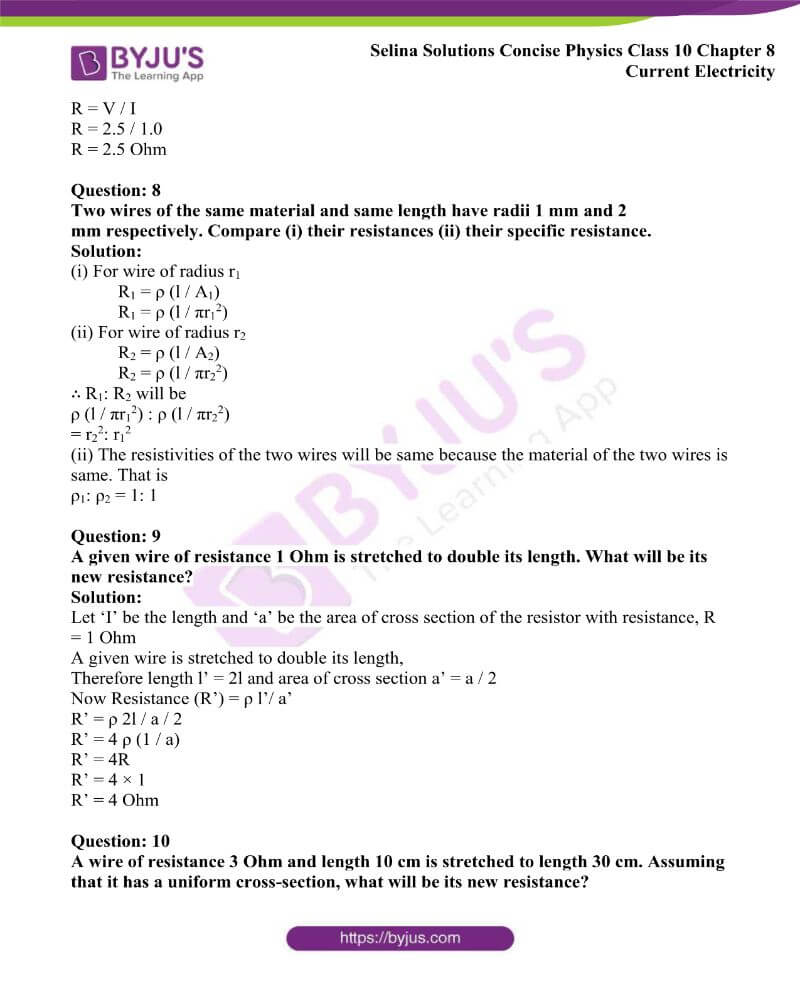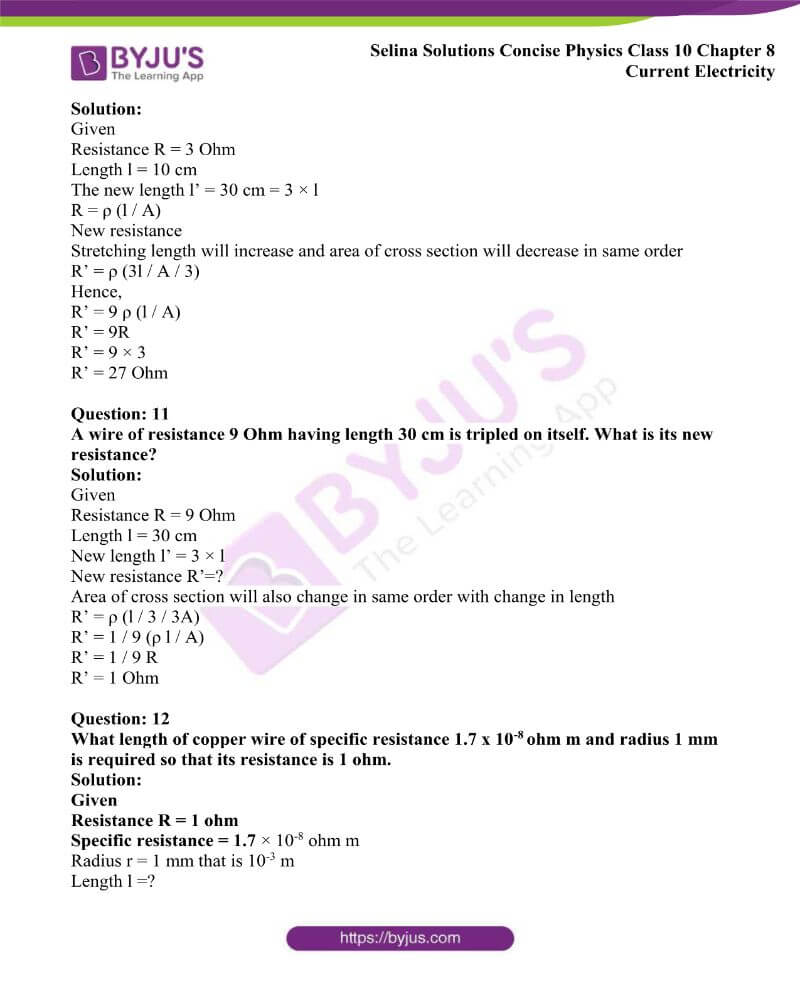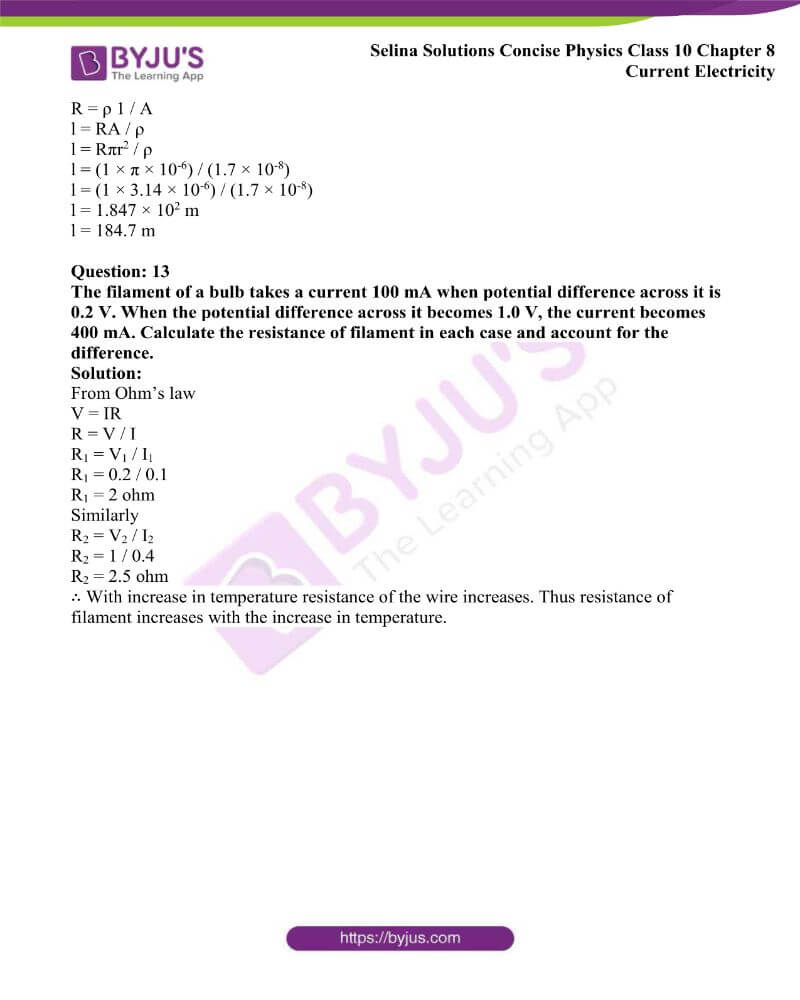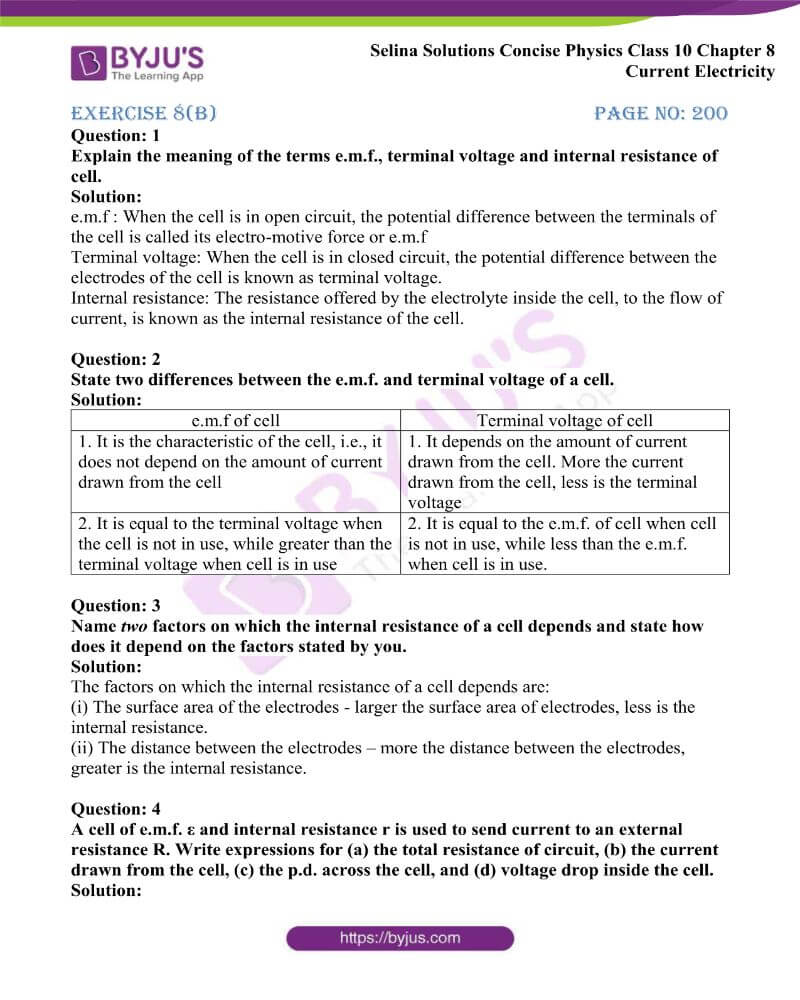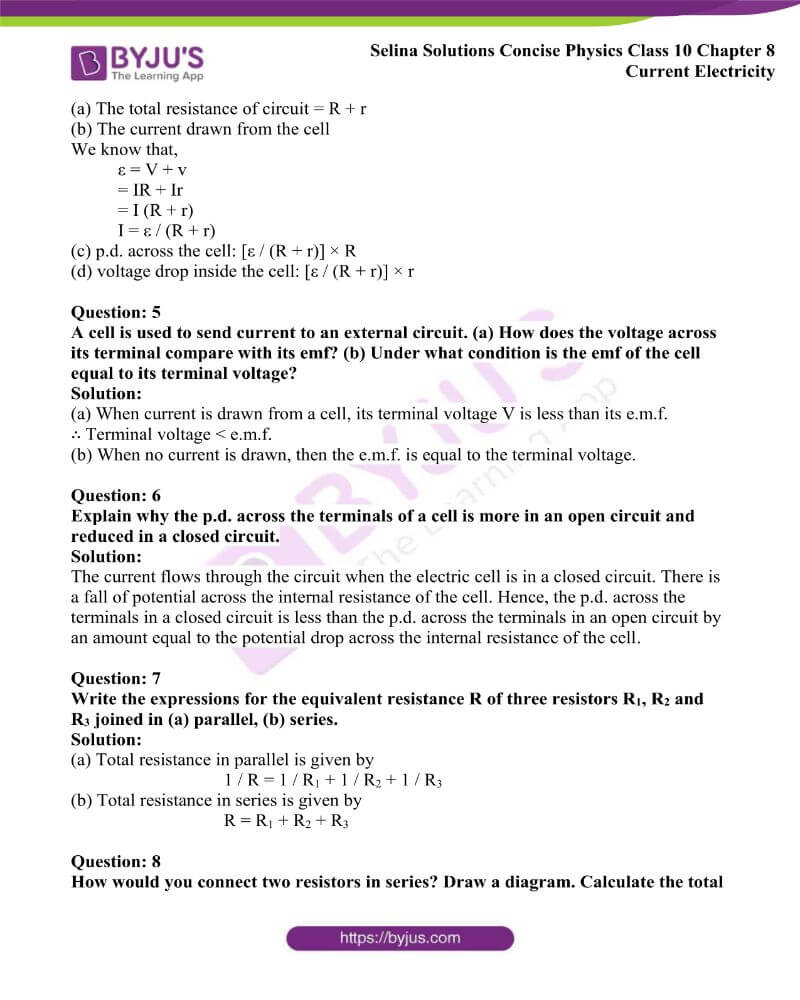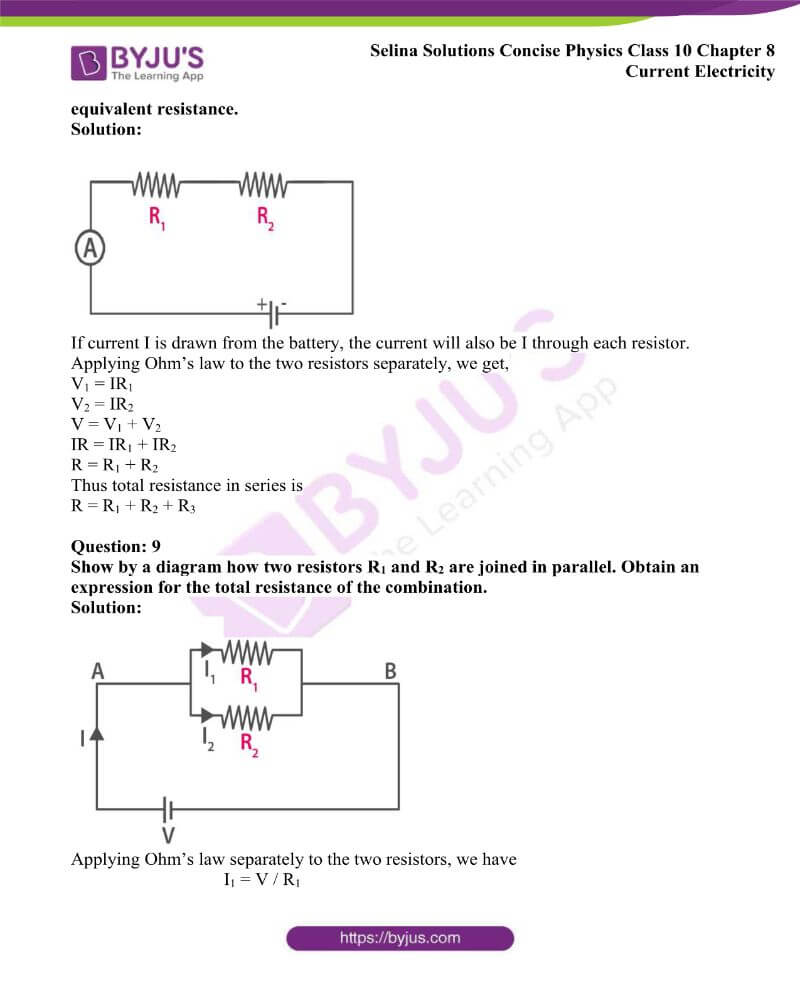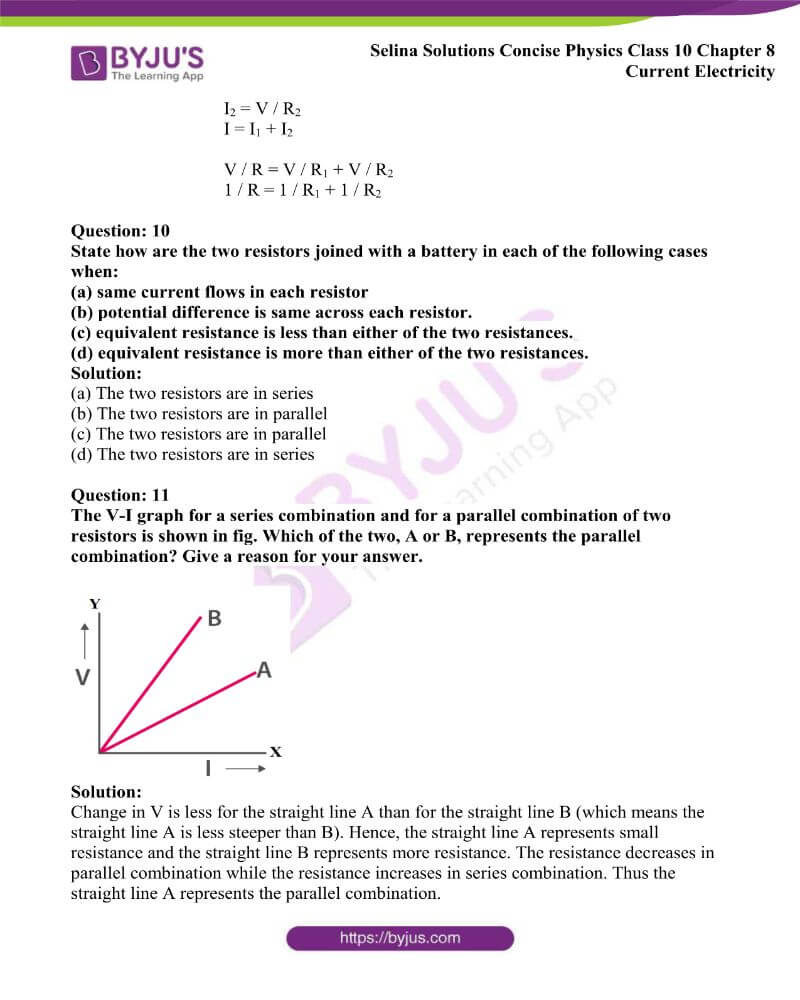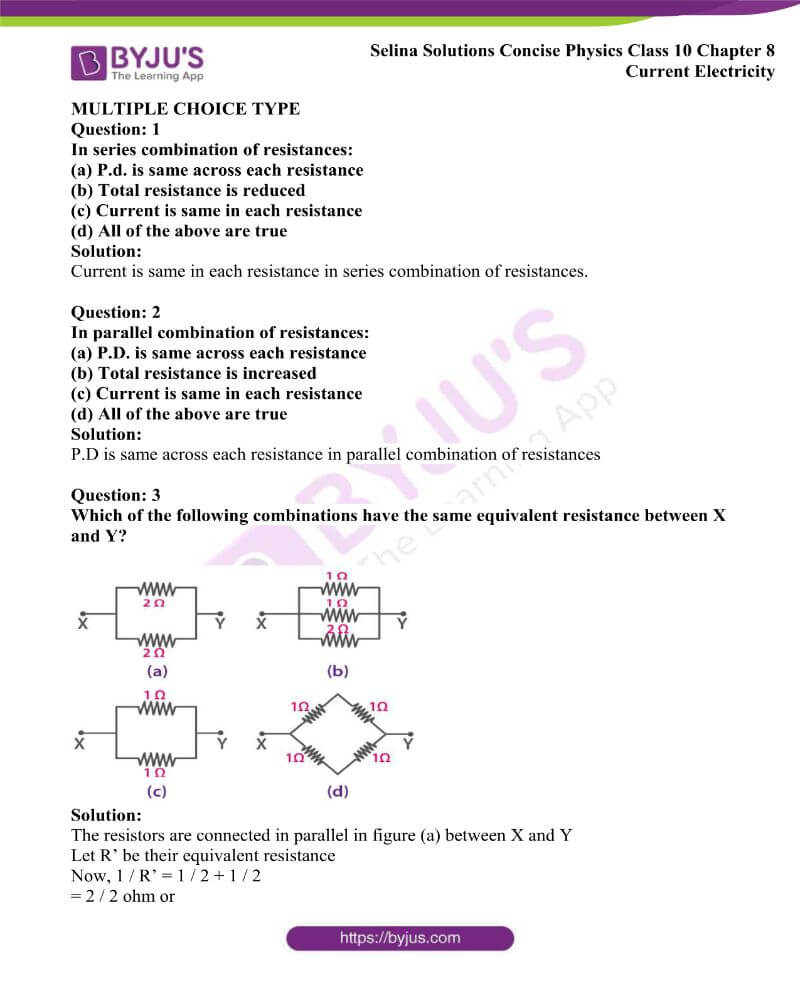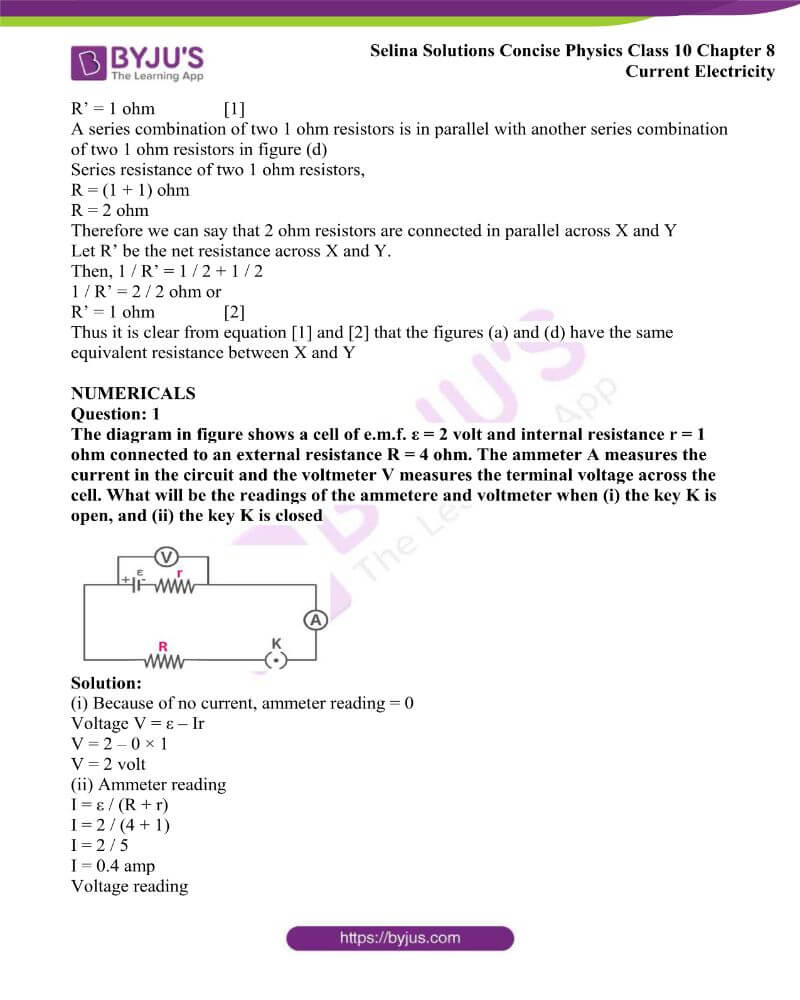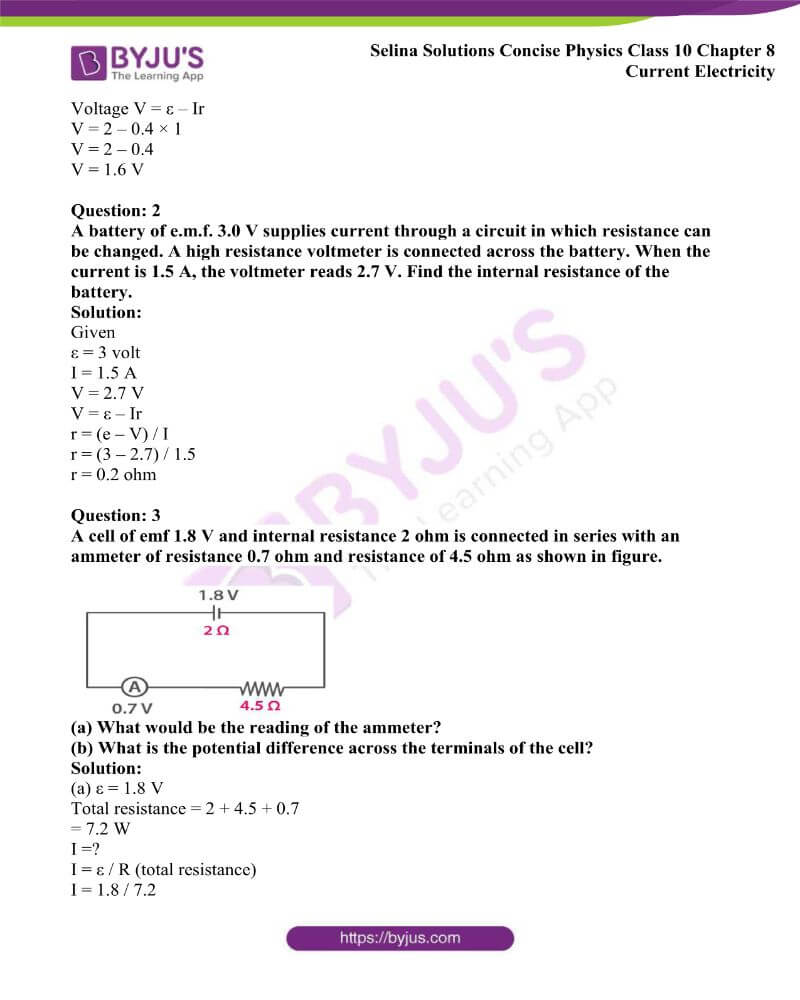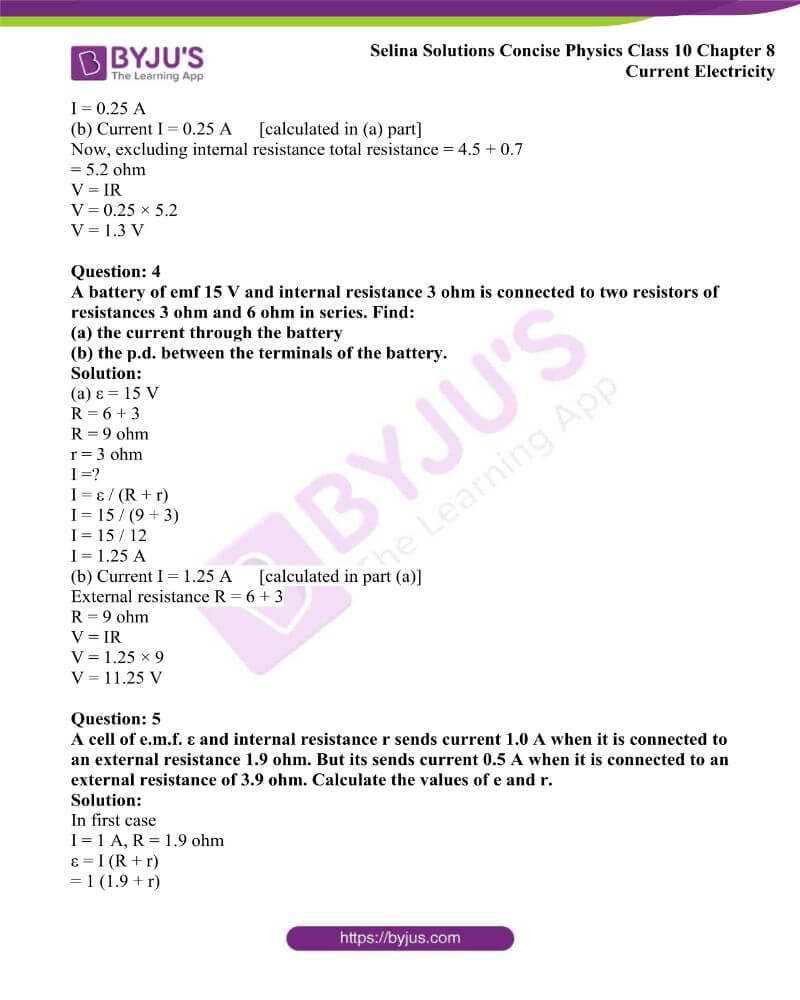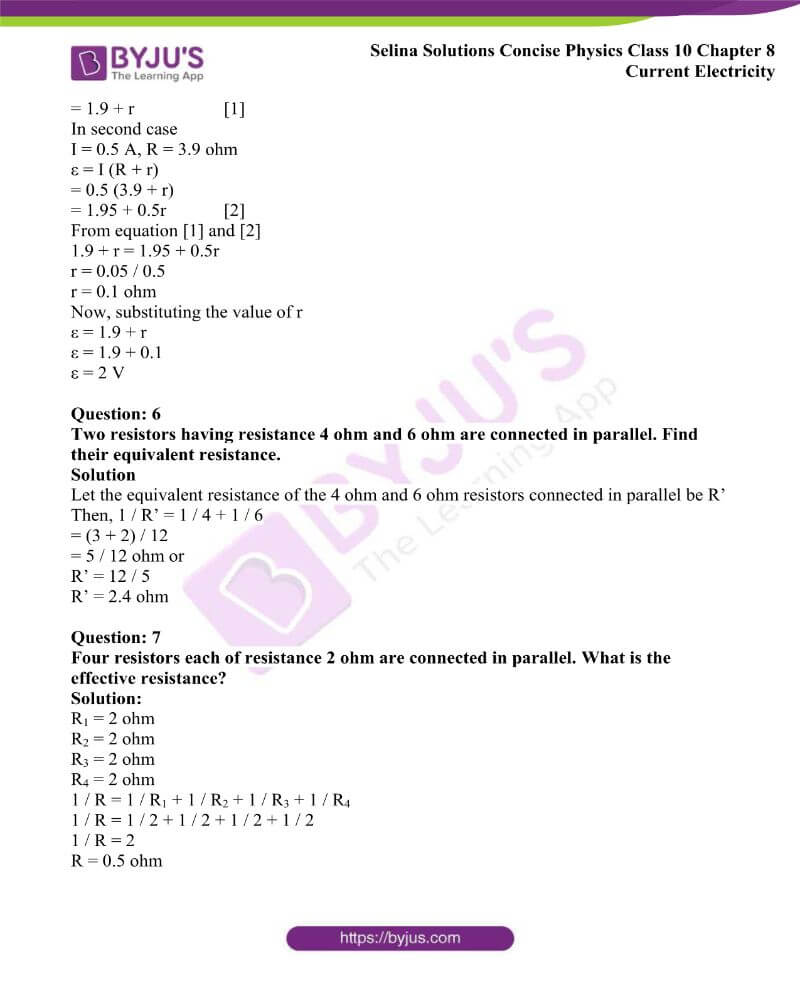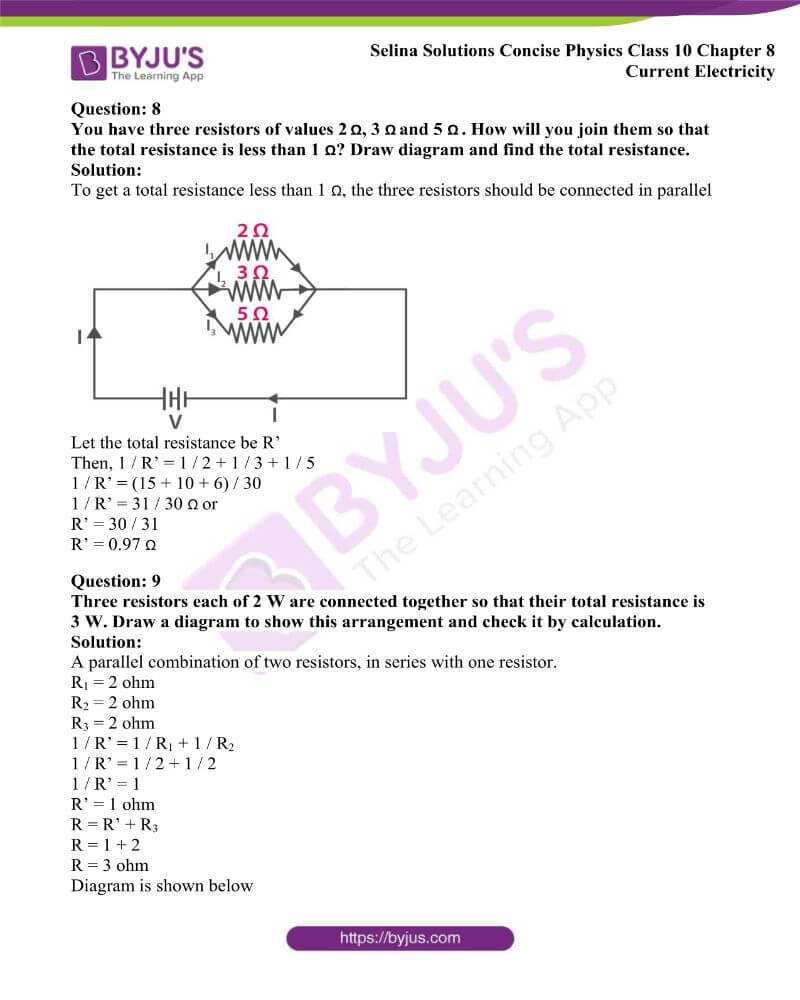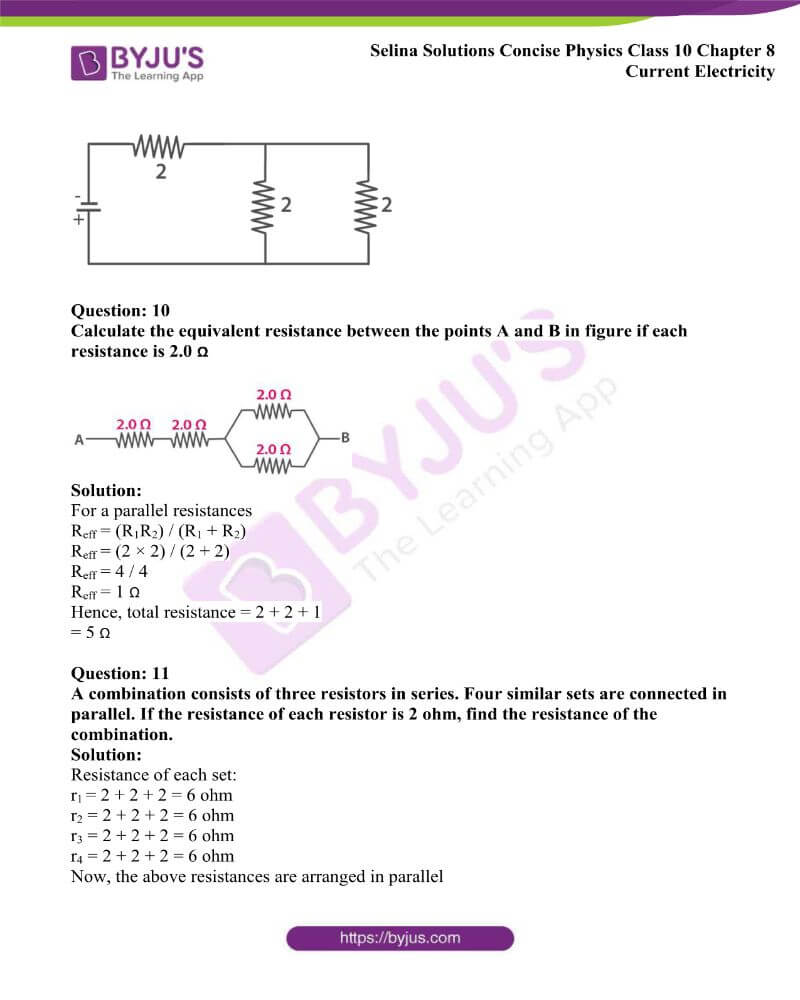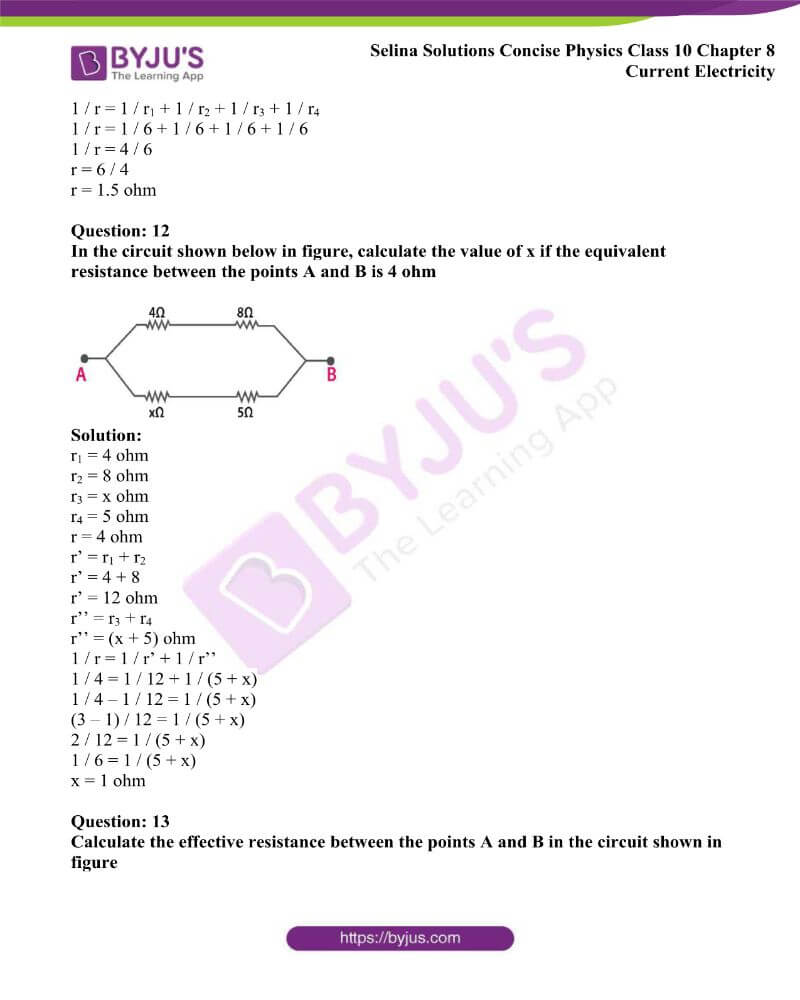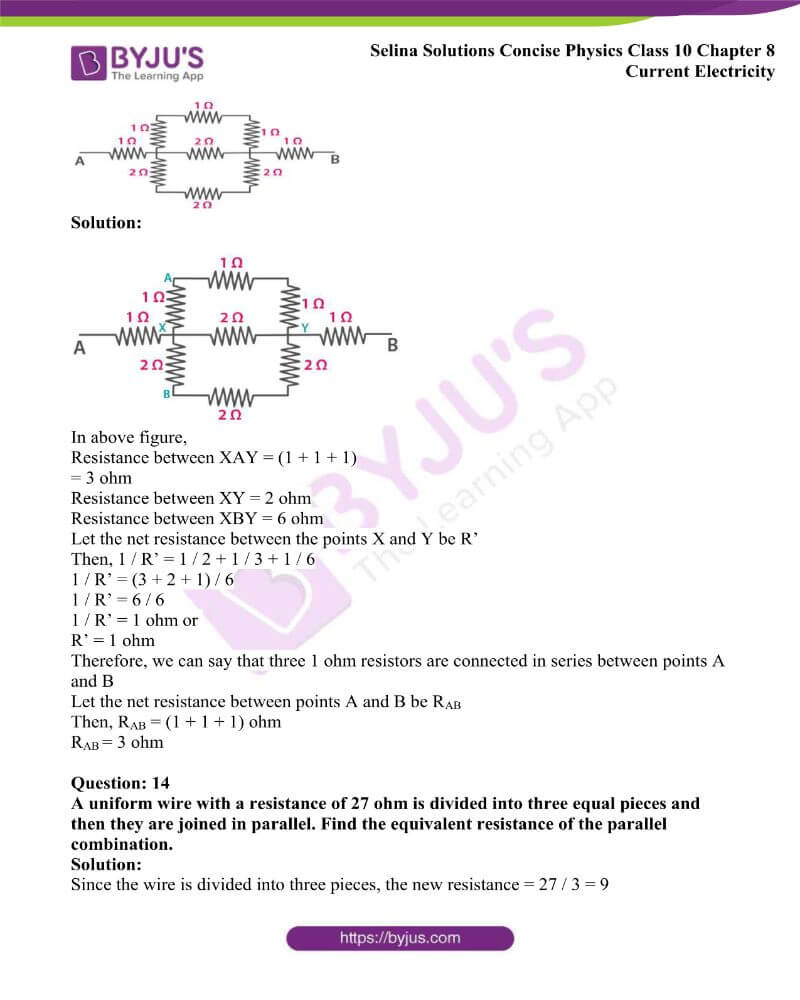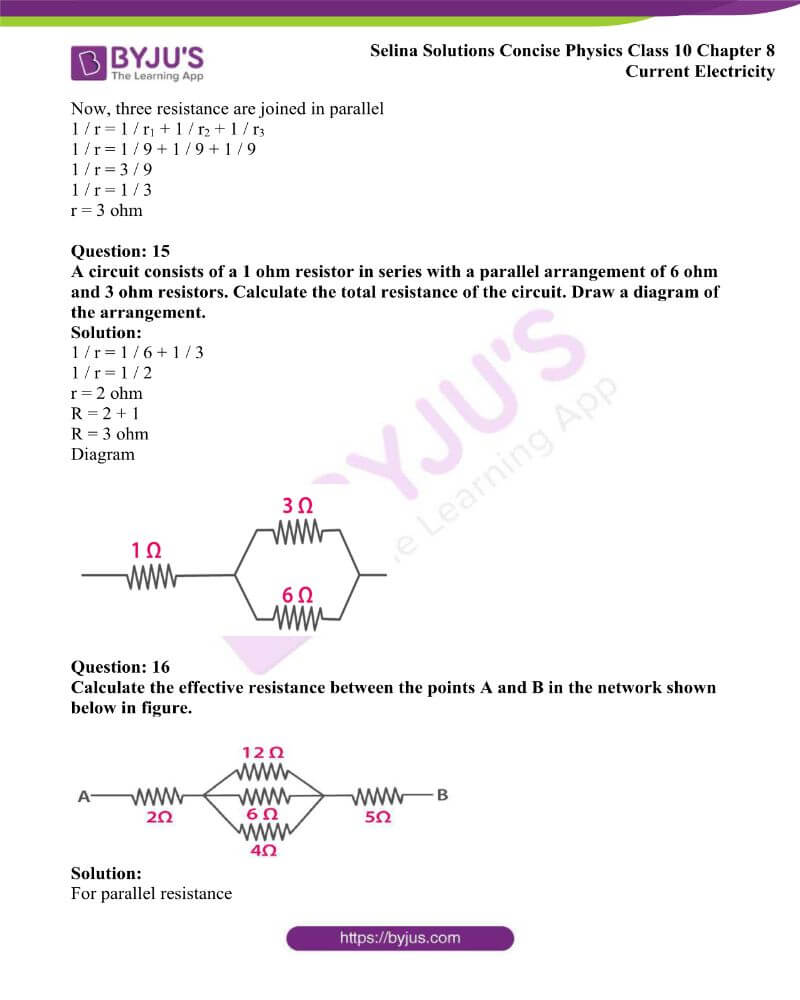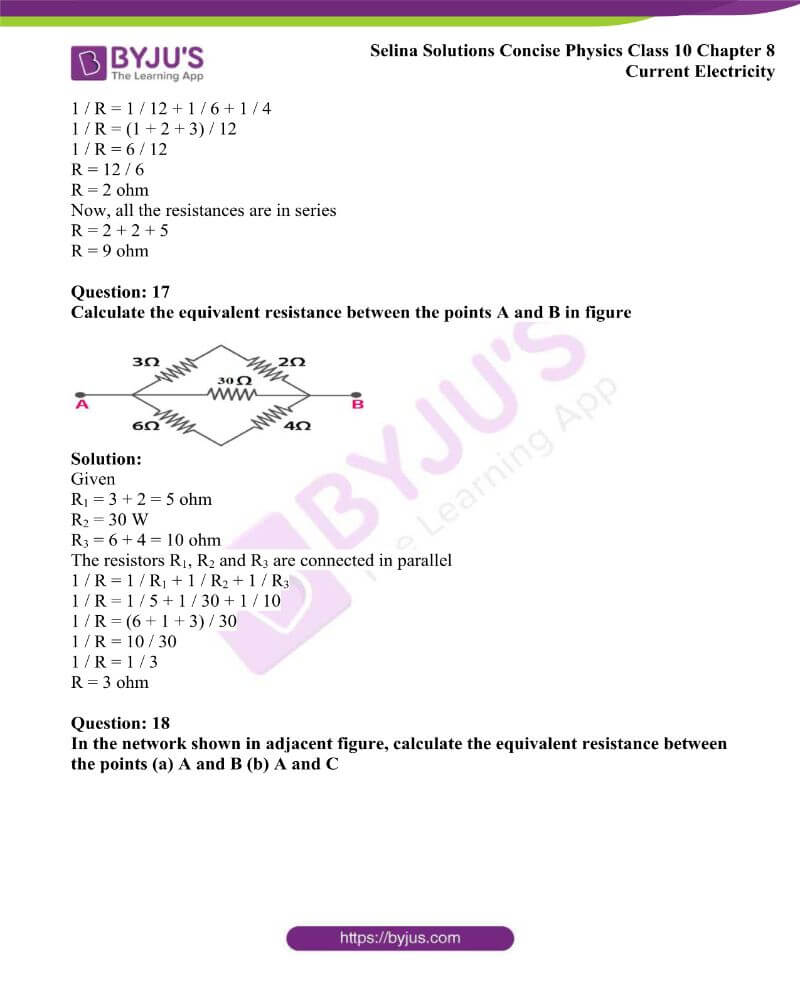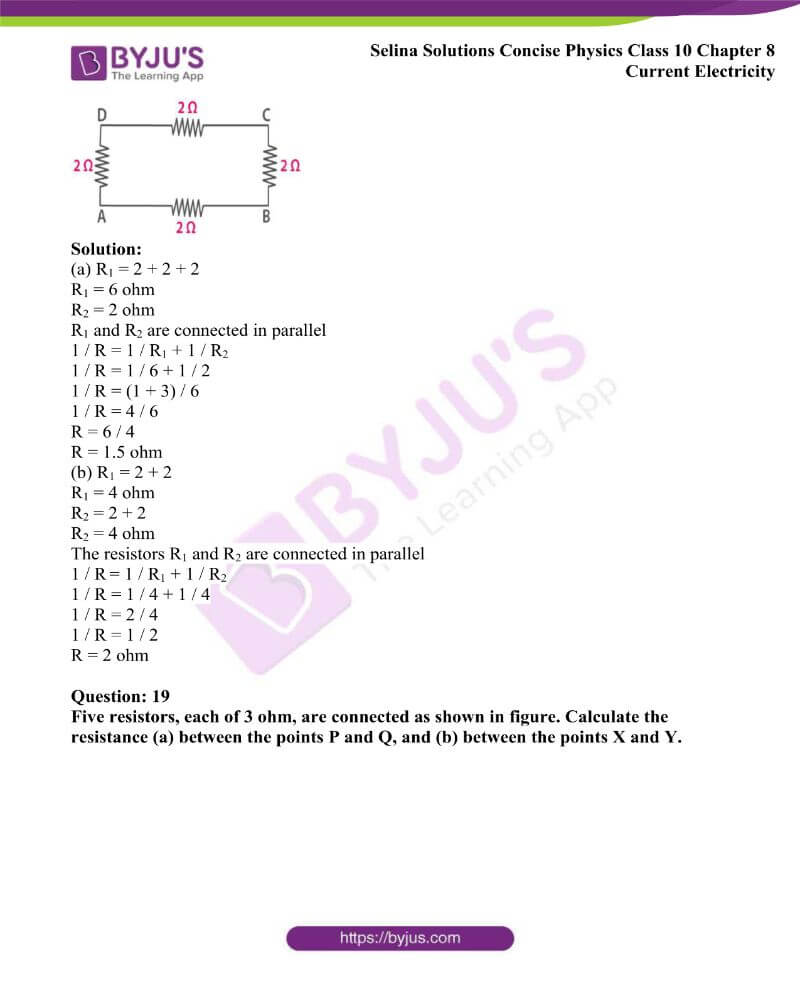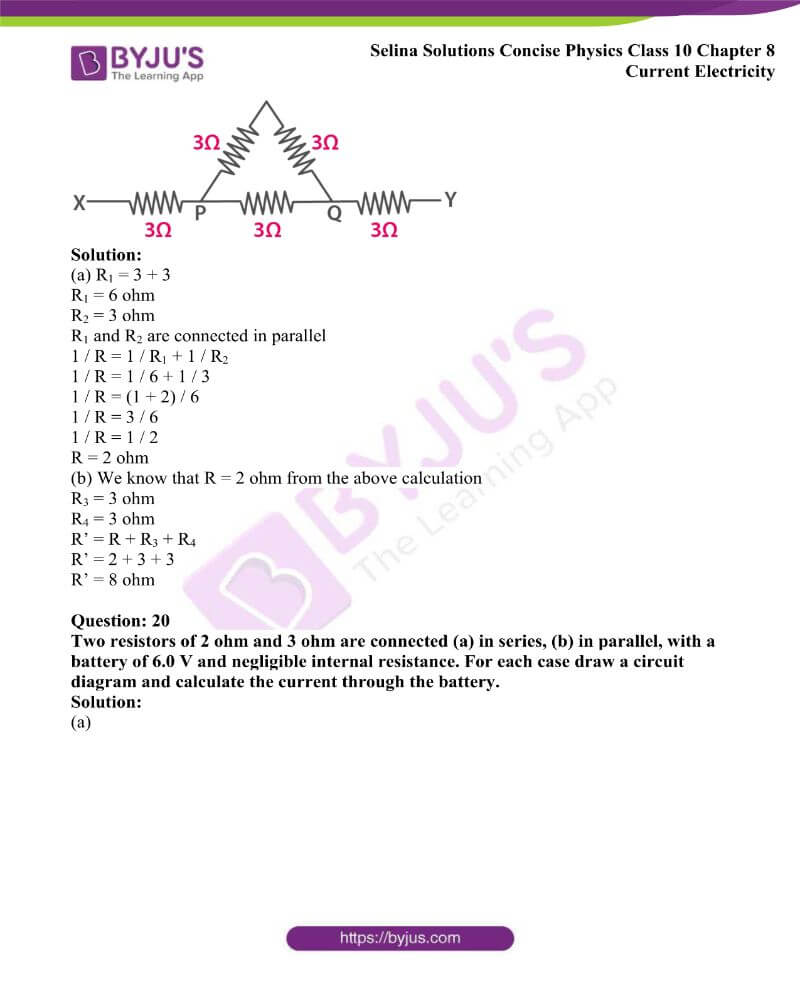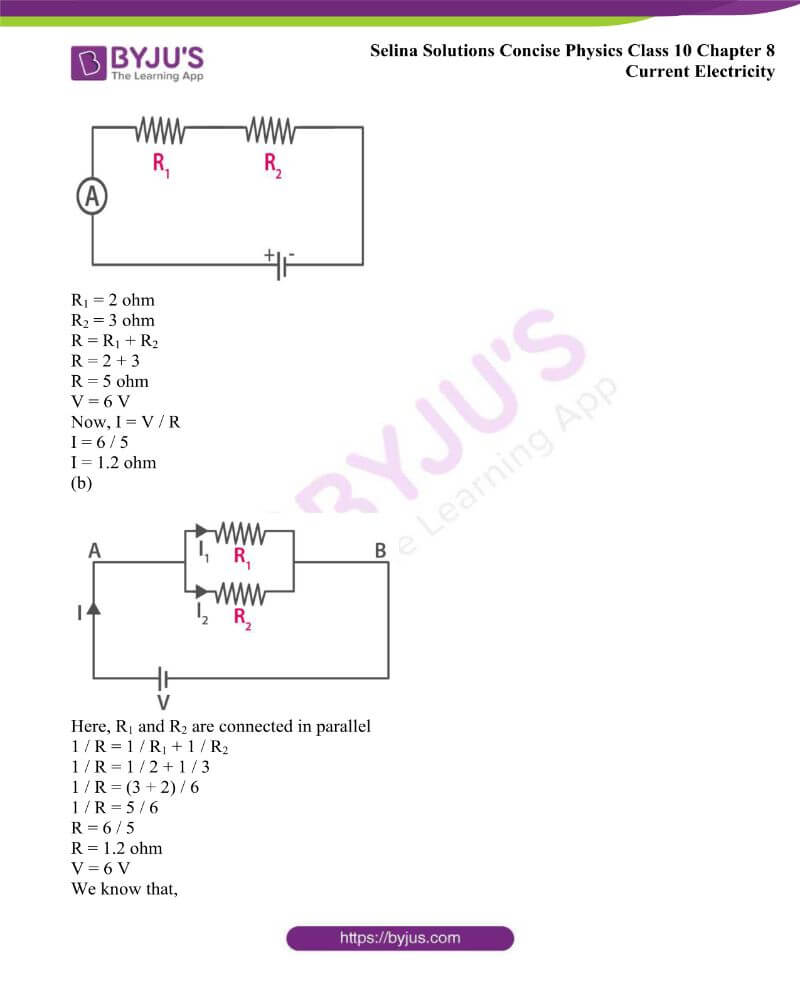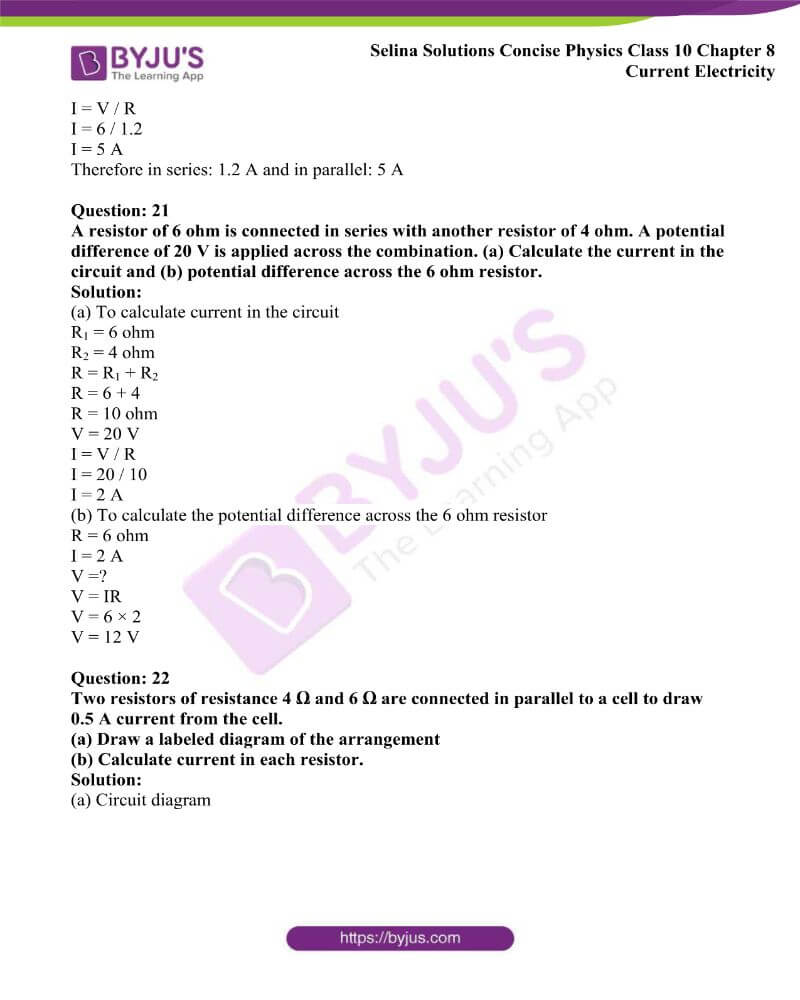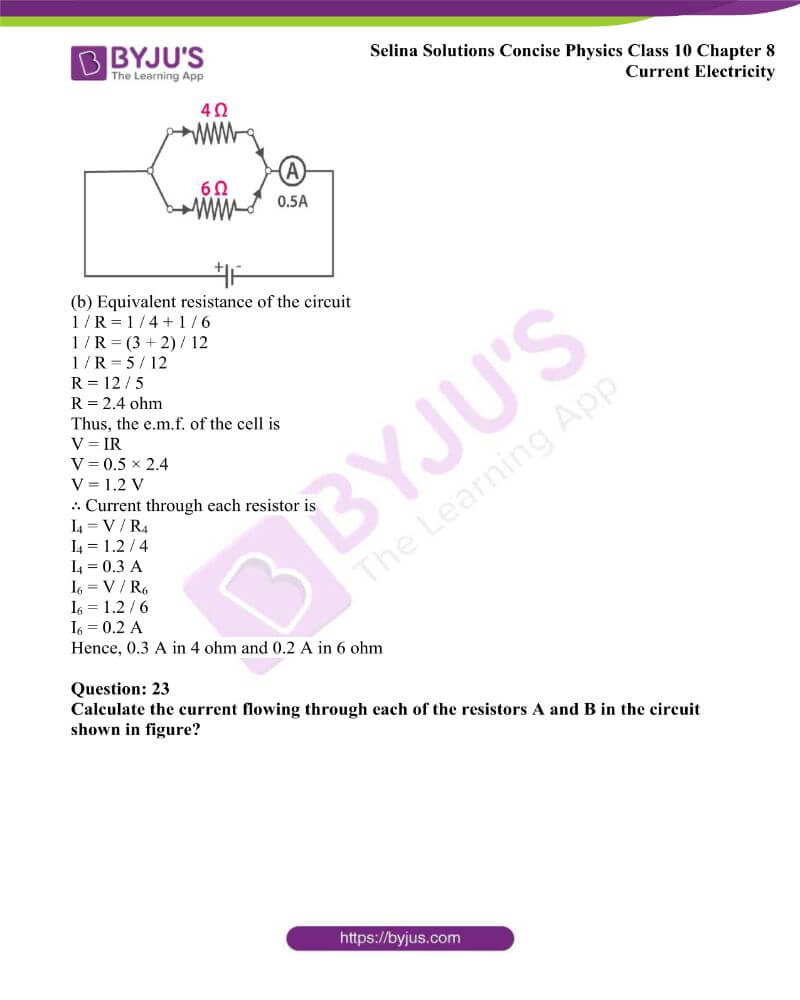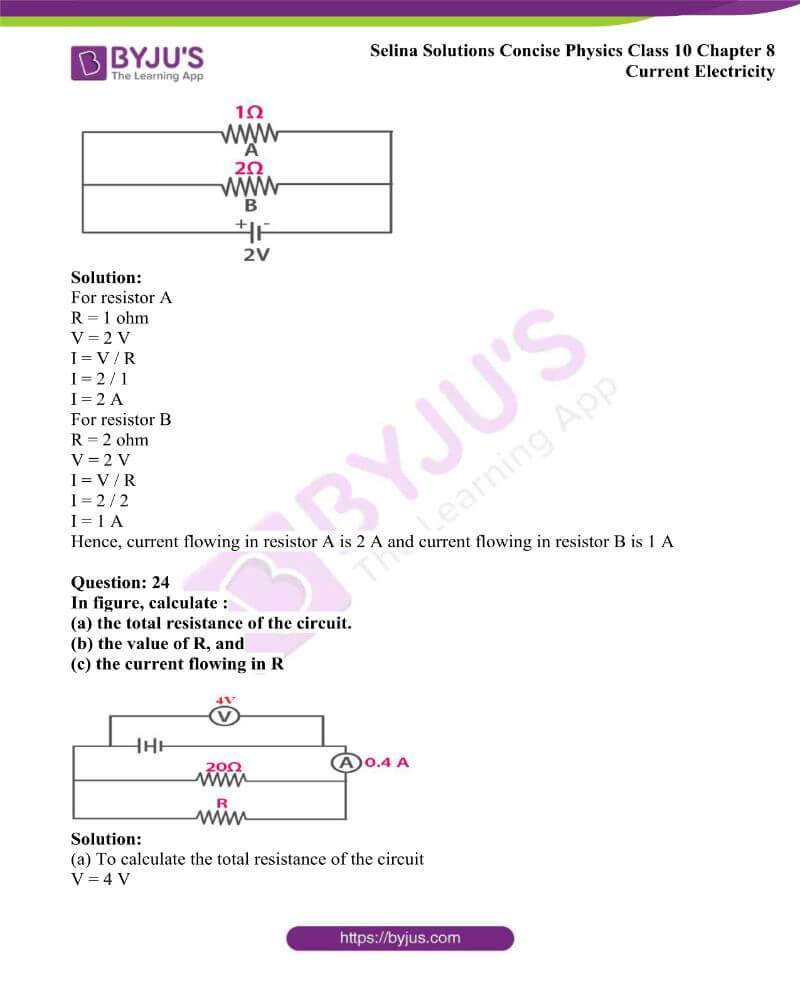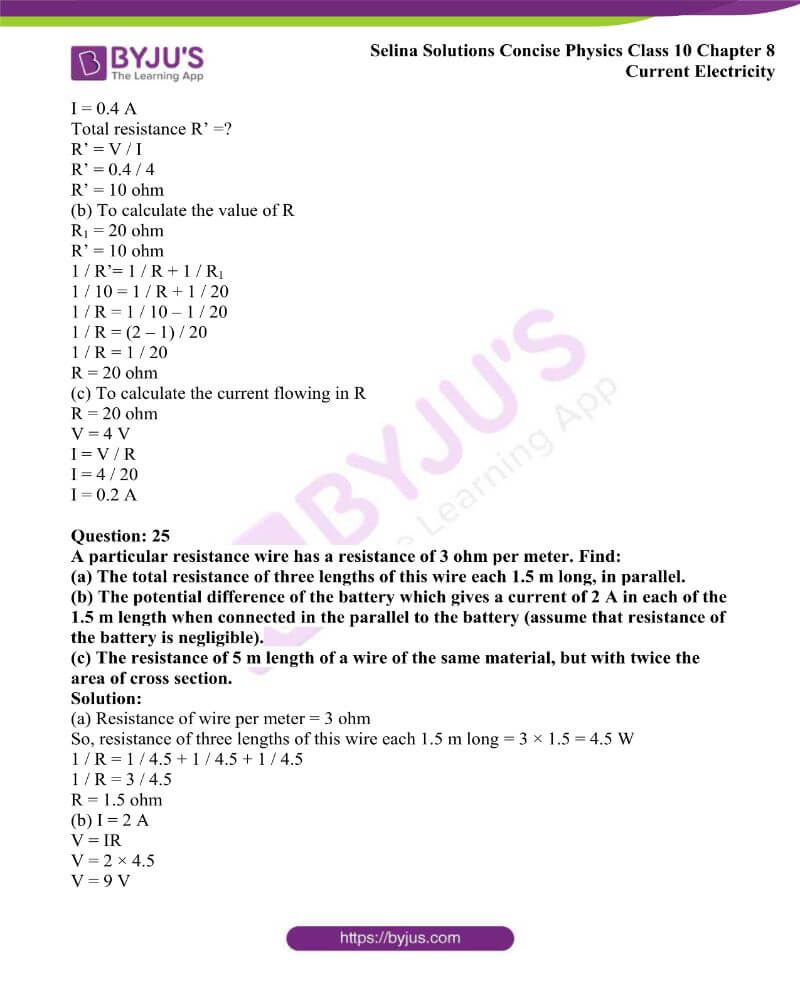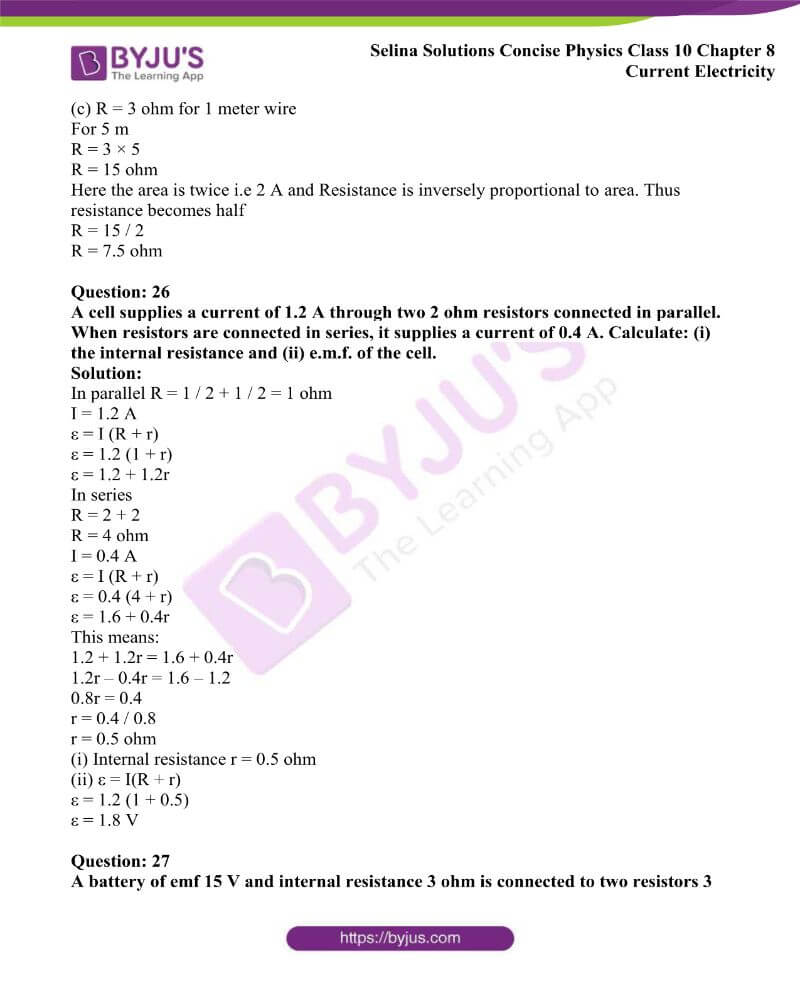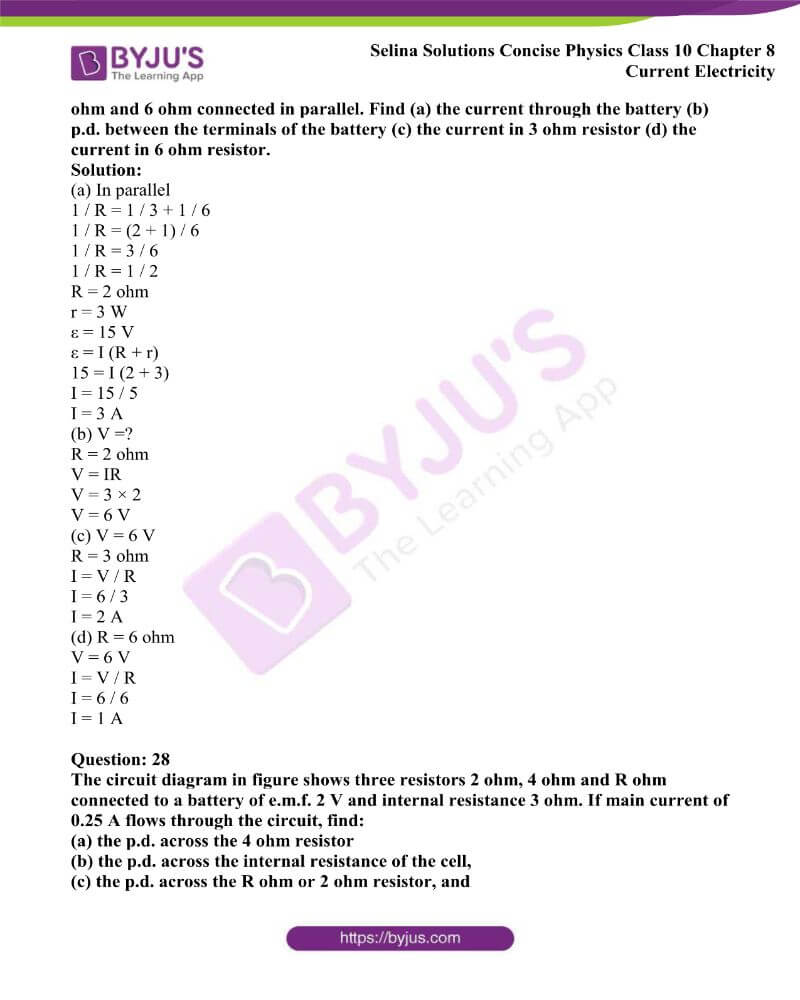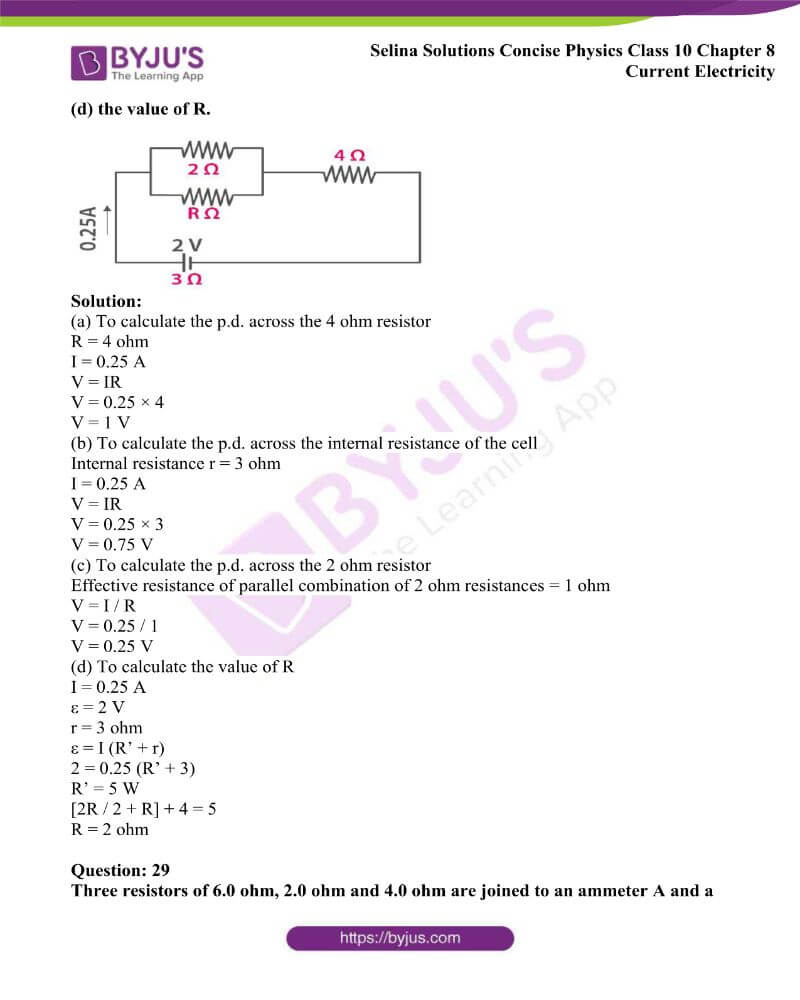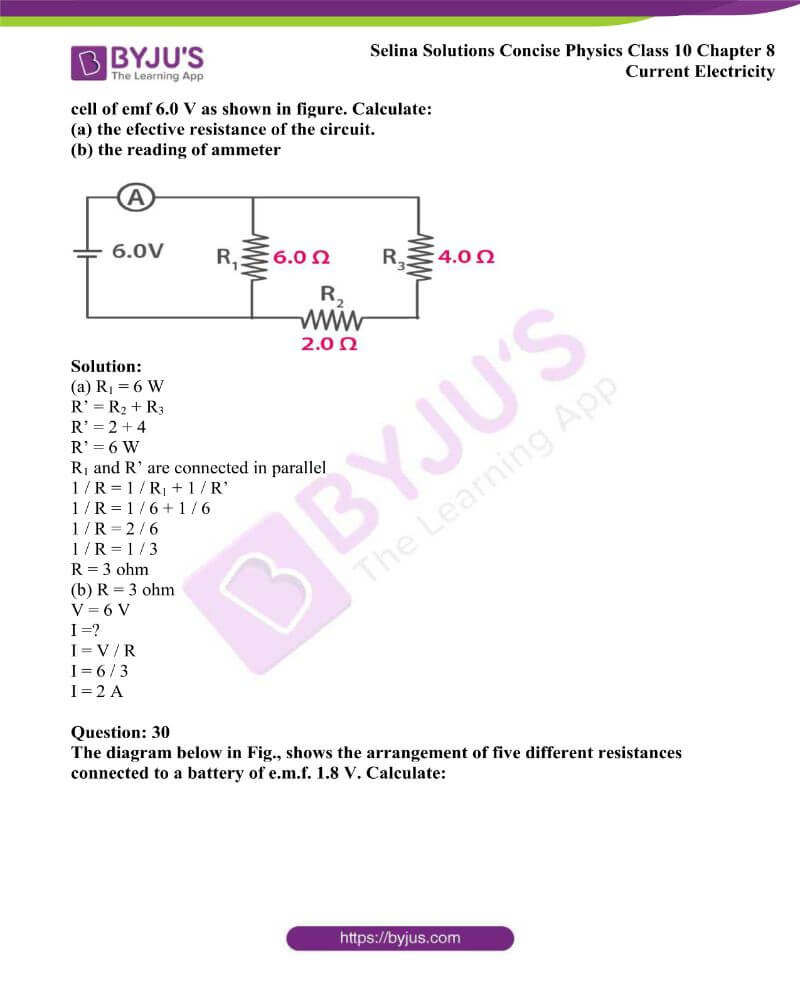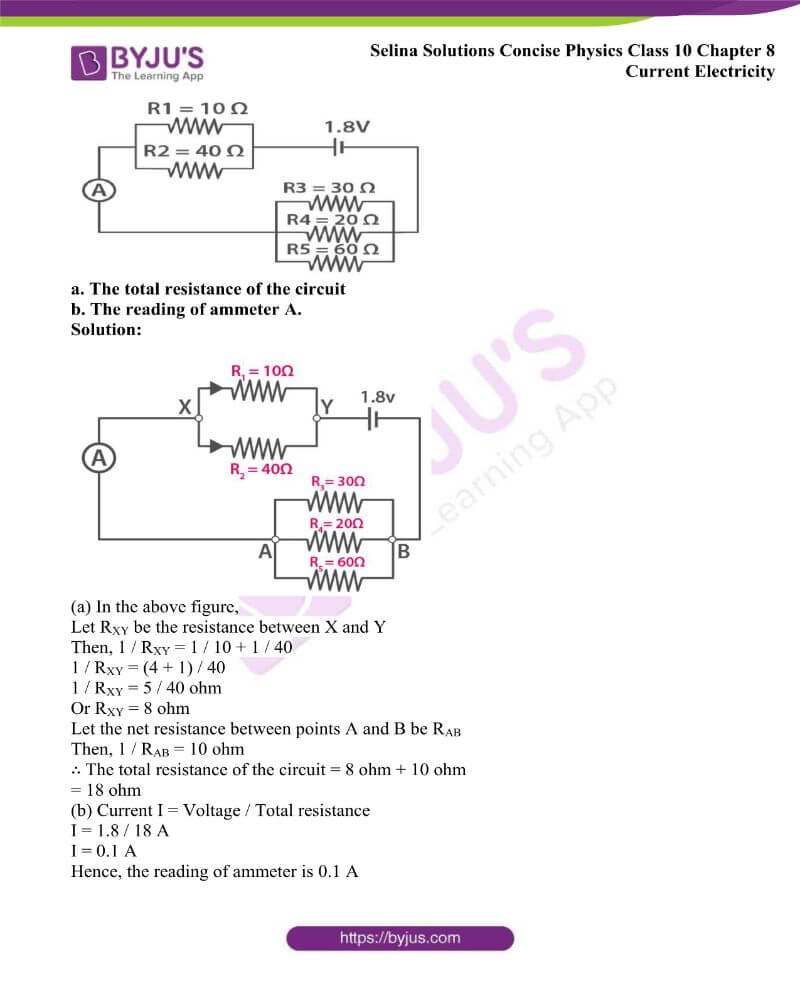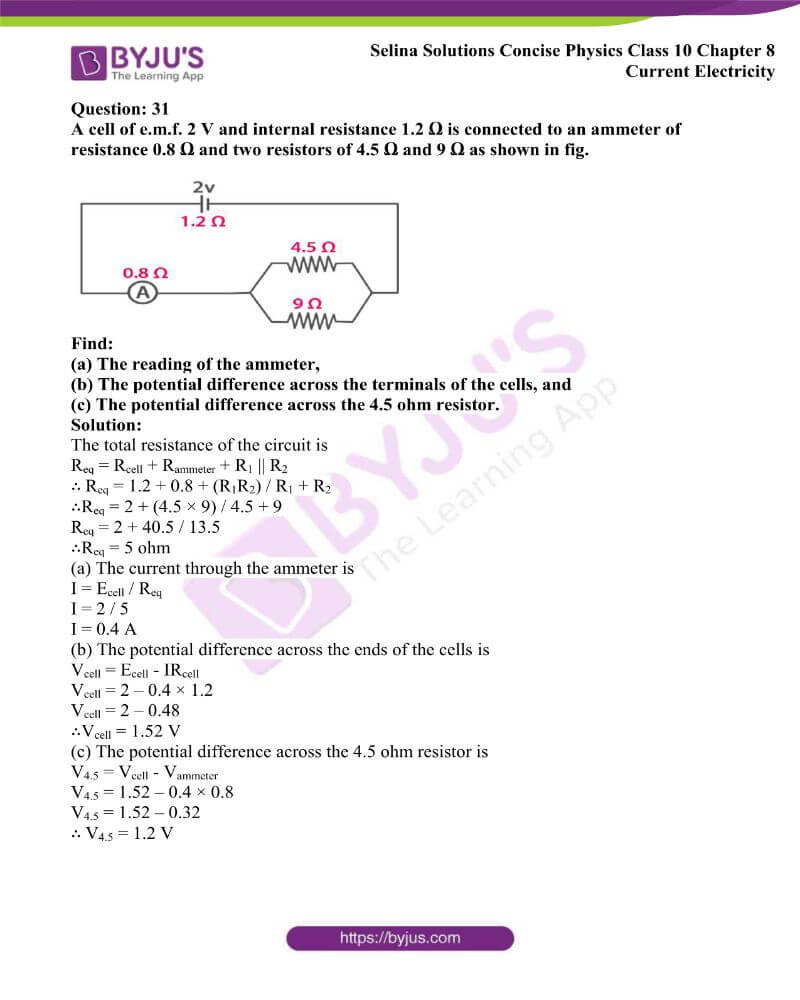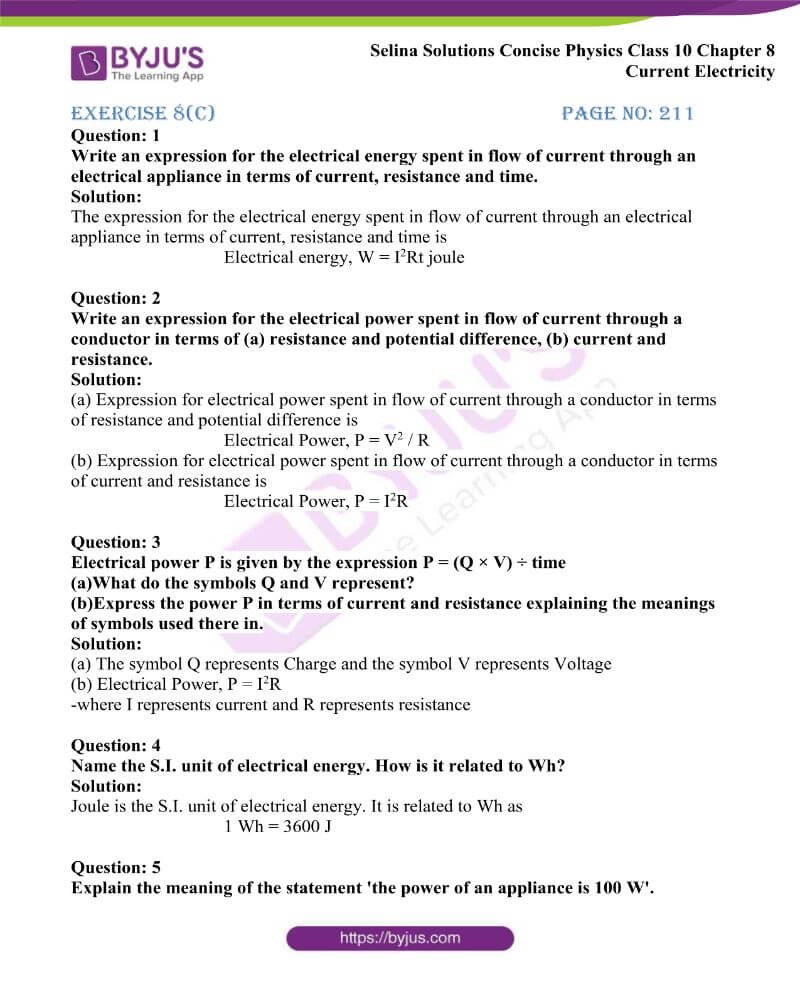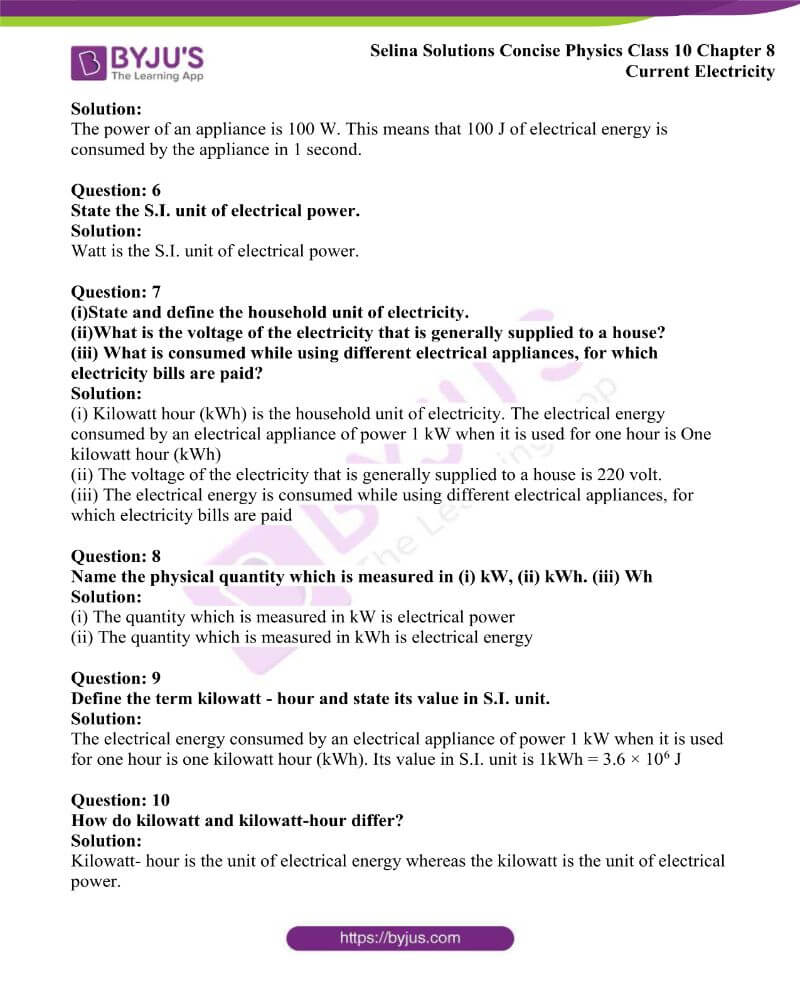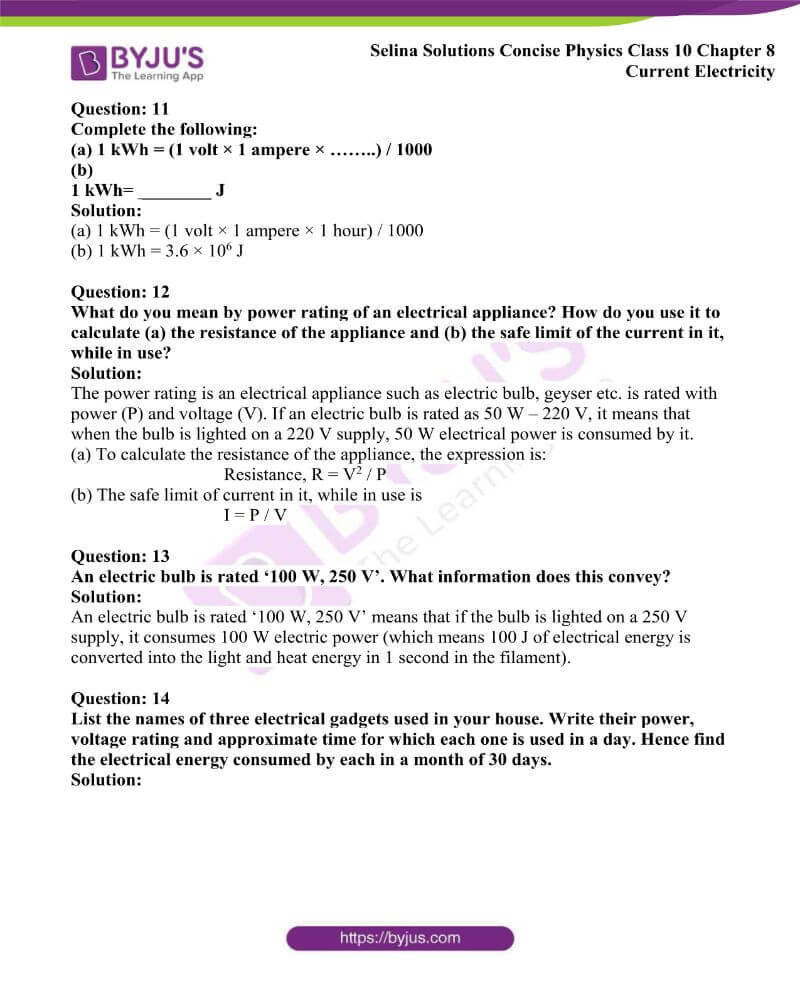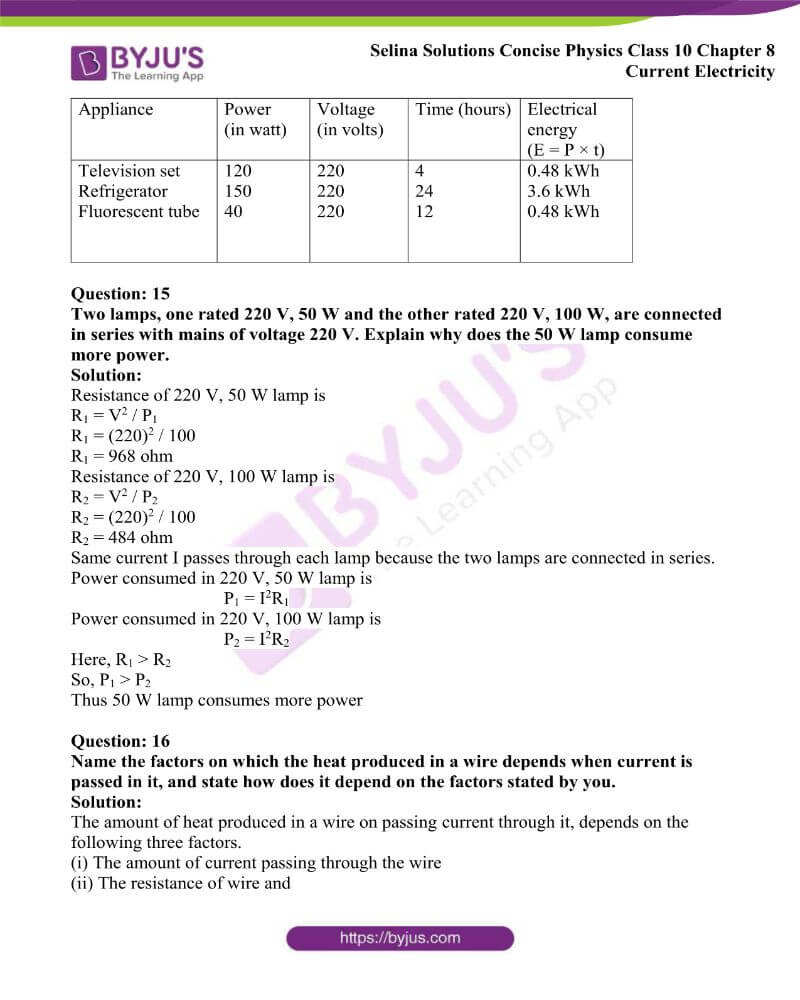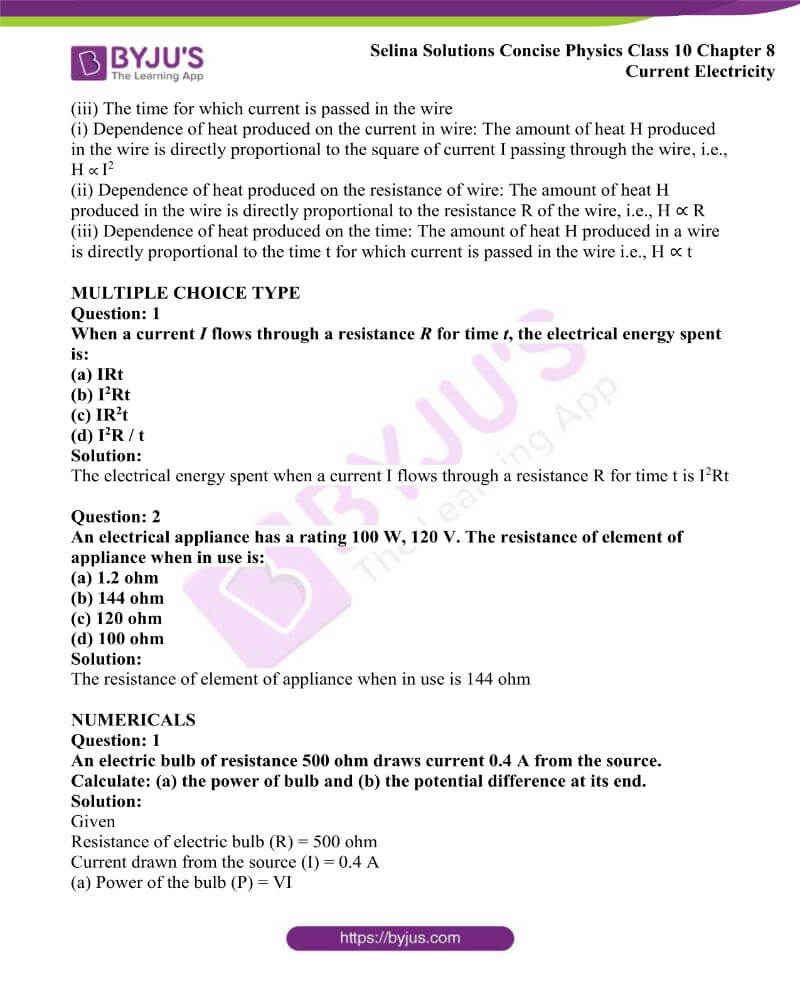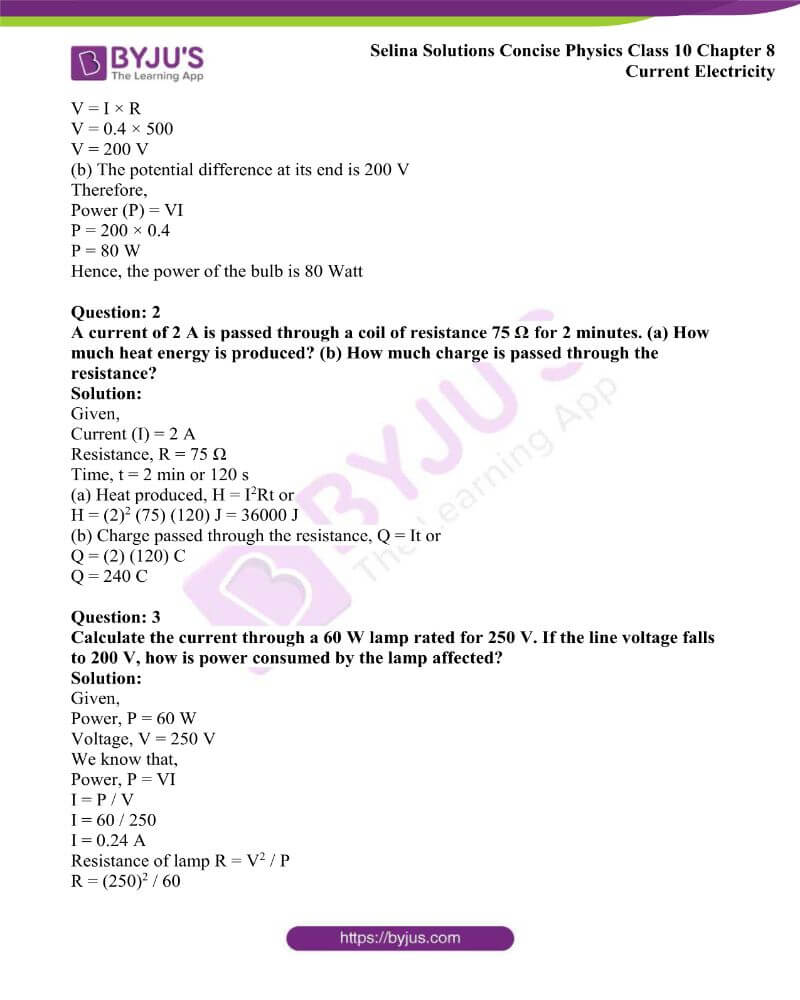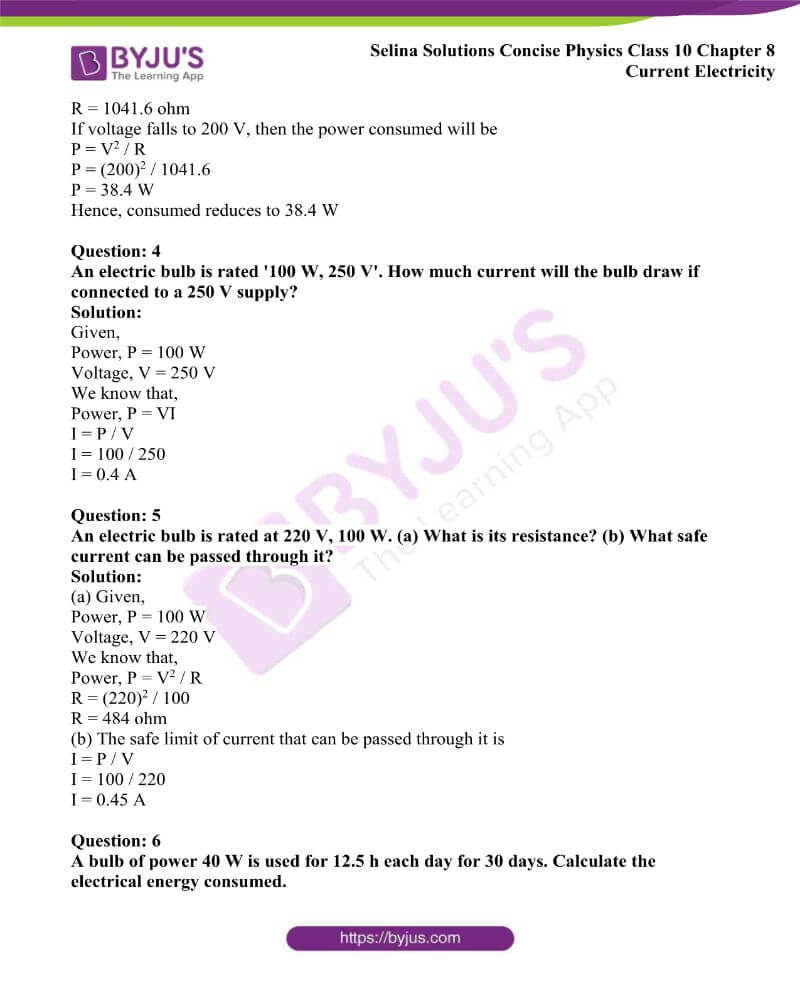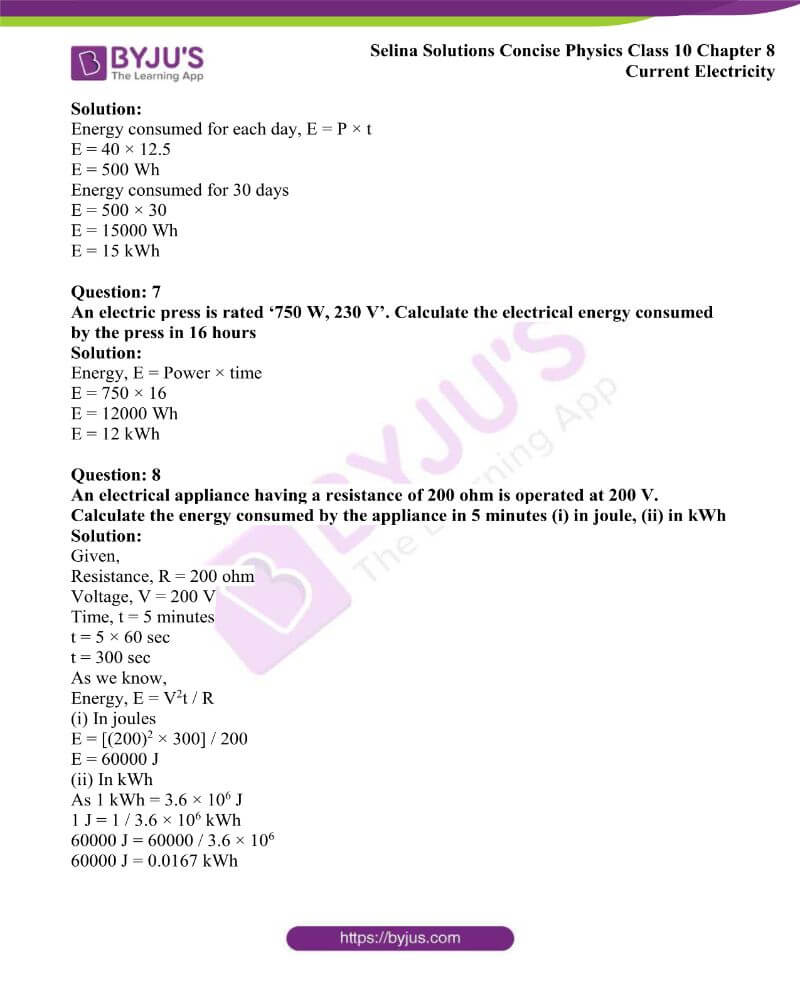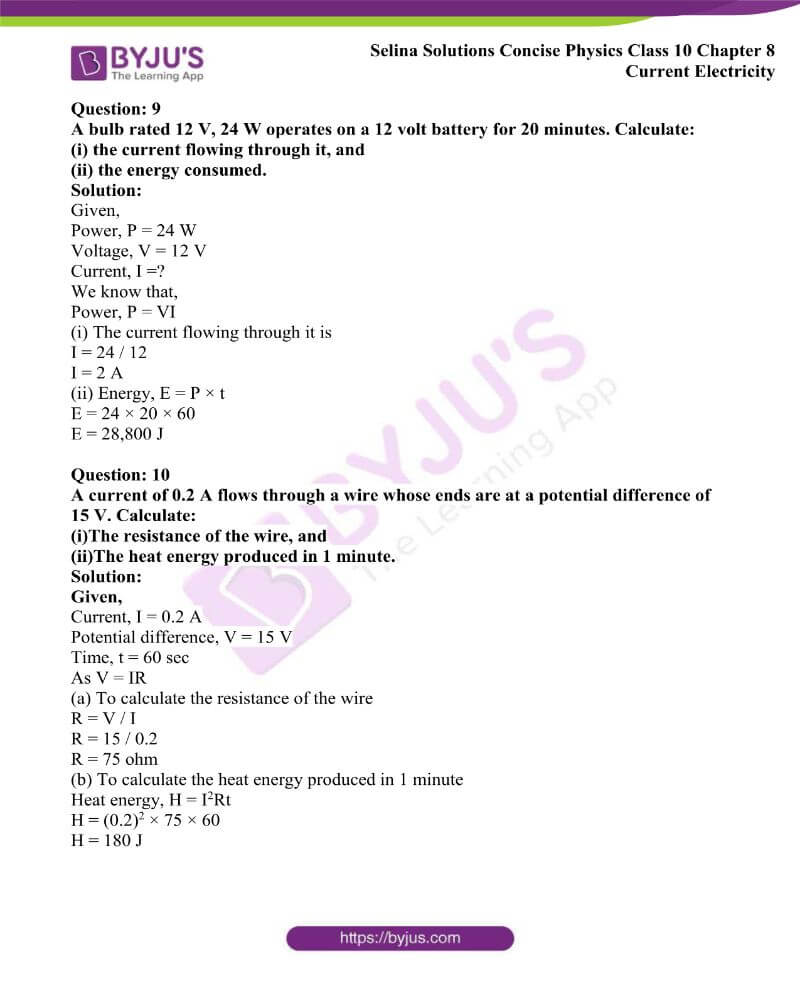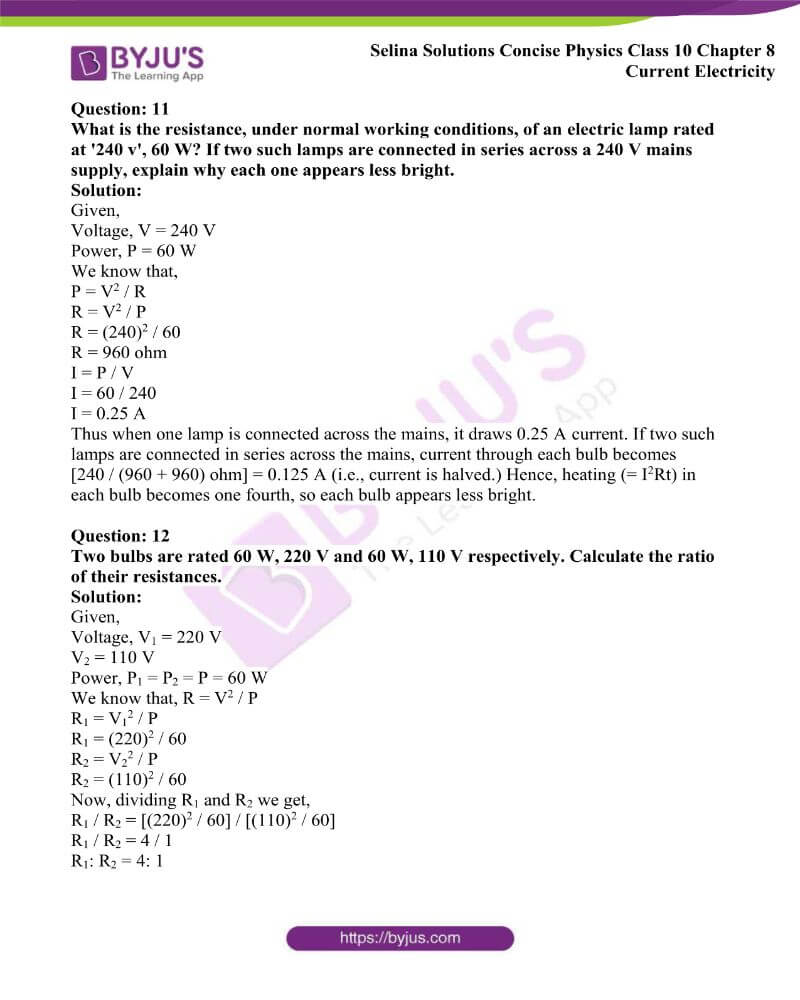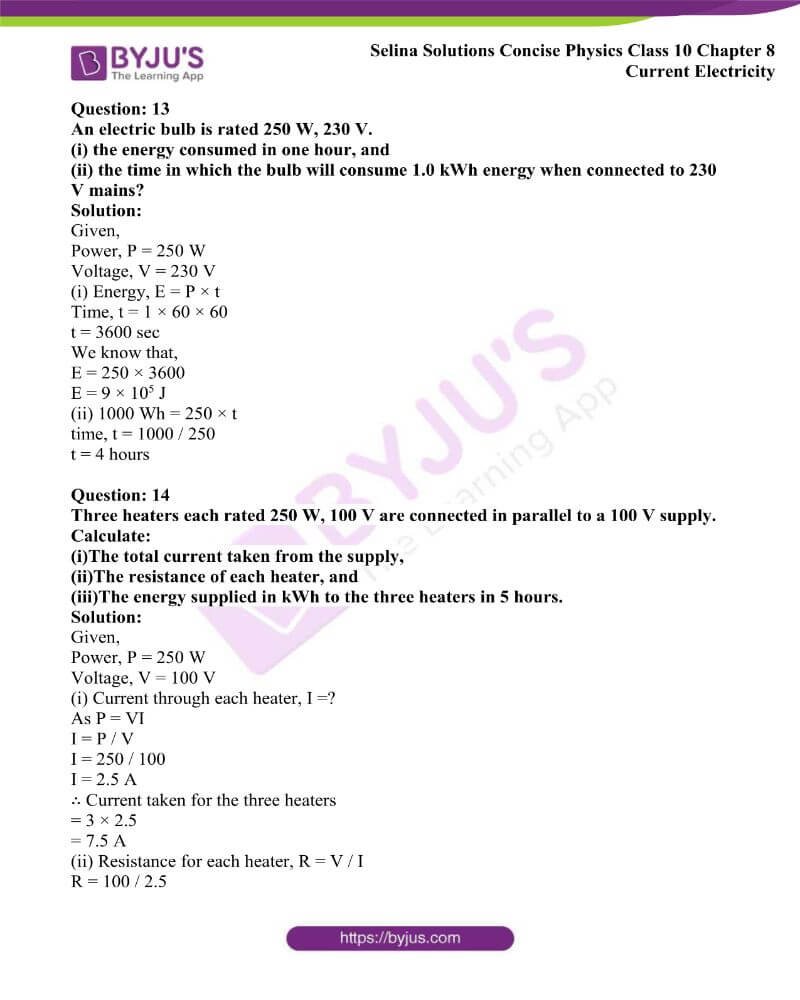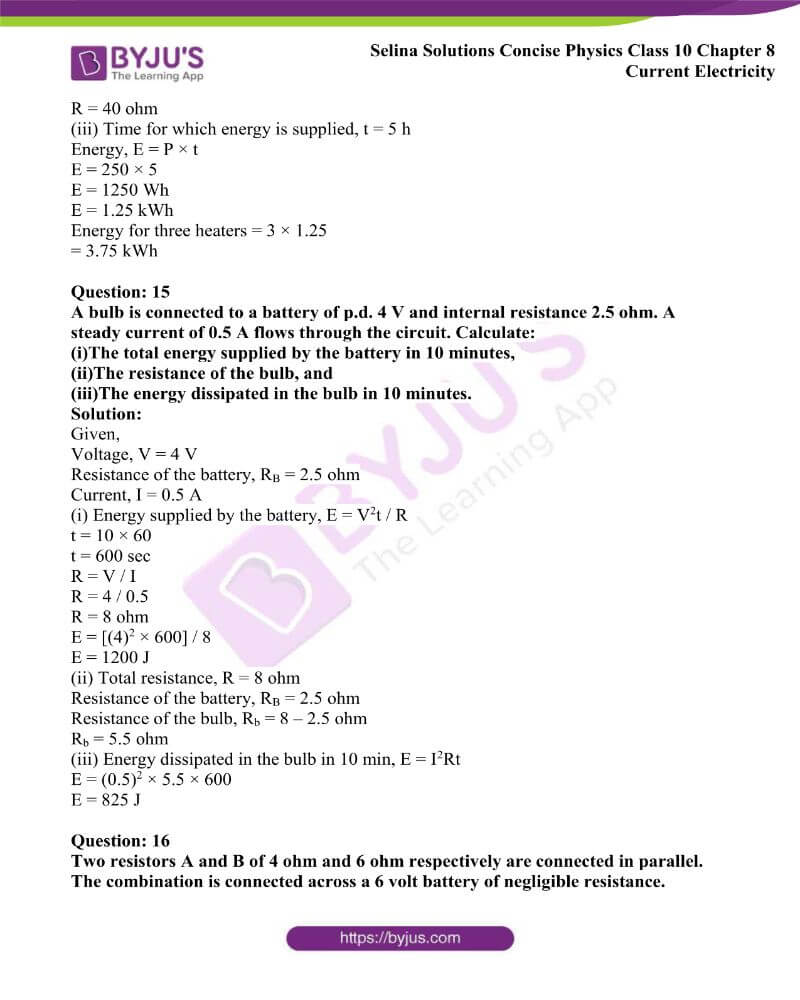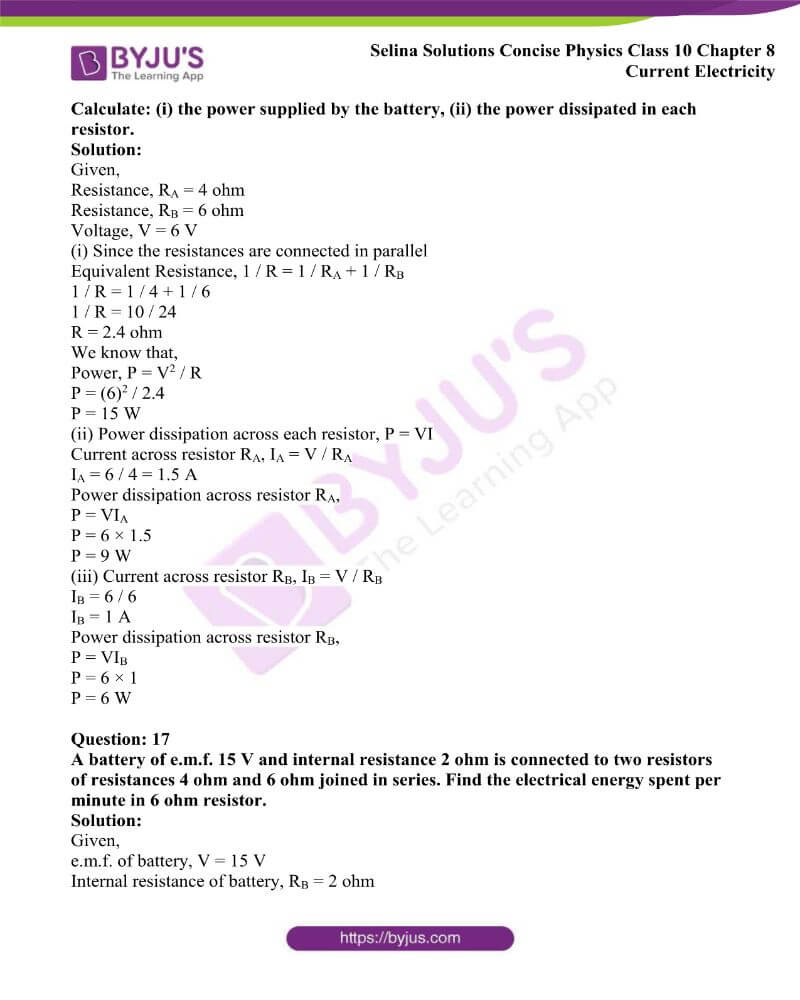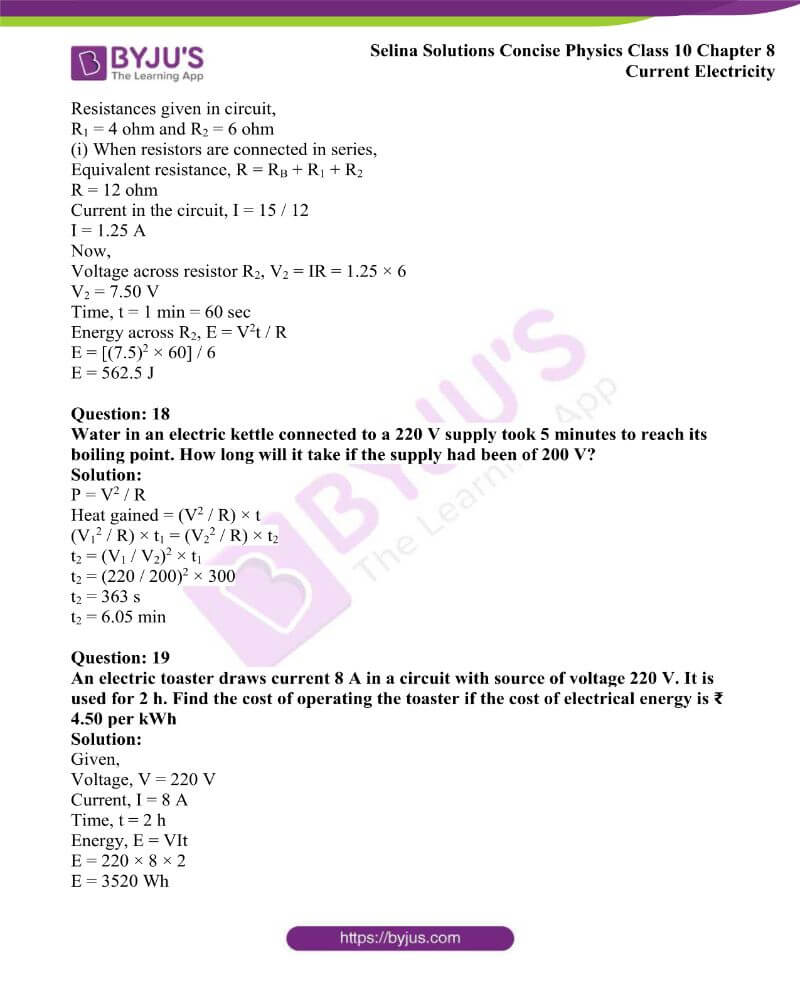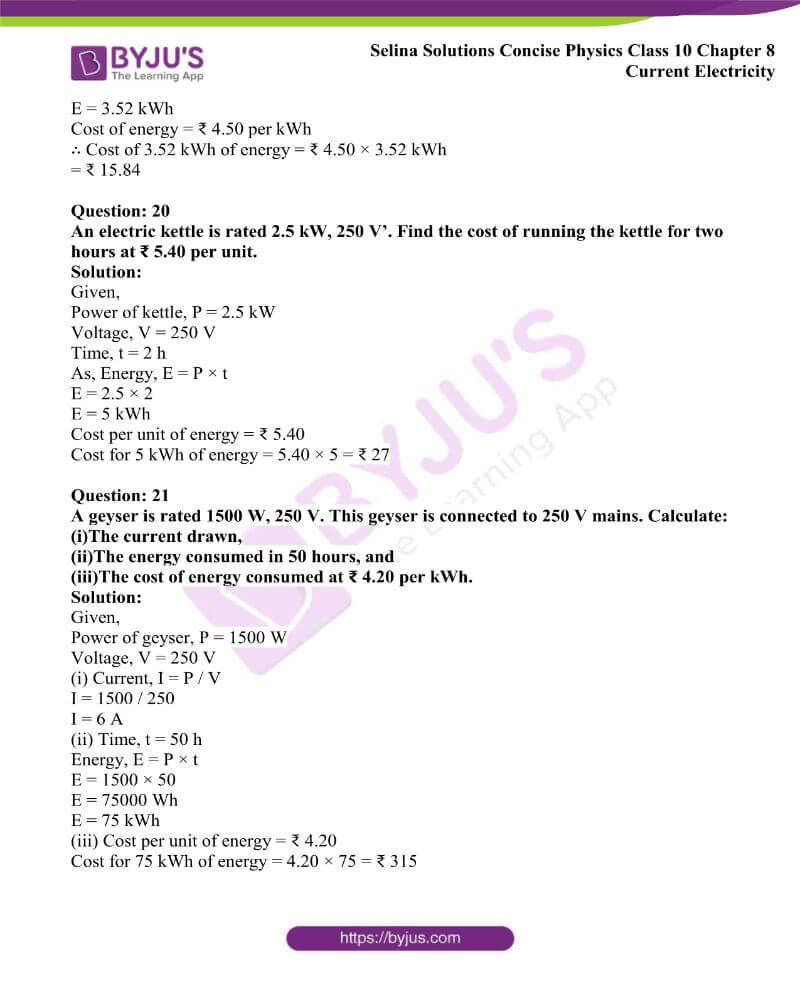### Access Answers to Physics Selina Solutions Concise Physics Class 10 Chapter 8 Current Electricity

Exercise 8(A) page no: 186

Question: 1

Define the term current and state its S.I. unit.

Solution:

The rate of flow of charge is known as current. The S.I. unit of current is Ampere.

Question: 2

Define the term electric potential. State it’s S.I. unit.

Solution:

The amount of work done per unit charge in bringing a positive test charge from infinity to that point is known as electric potential at a point. The S.I. unit of electric potential is volt.

Question: 3

How is the electric potential difference between the two points defined? State its S.I. unit.

Solution:

The potential difference between two points can be defined as the potential difference between two points is equal to the work done per unit charge in moving a positive test charge from one point to the other. It’s S.I. unit is volt.

Question: 4

Explain the statement ‘the potential difference between two points is 1 volt’.

Solution:

The potential difference between two points is said to be 1 volt when 1 joule of work is done in bringing 1 coulomb charge from infinity to that point.

Question: 5

(a) State whether the current is a scalar or vector? What does the direction of current convey?

(b) State whether the potential is a scalar or vector? What does the positive and negative sign of potential convey?

Solution:

(a) Current is a scalar quantity. The direction of current conveys that the electrons flow opposite to the direction of flow of current.

(b) Potential is a scalar quantity. The positive sign of potential states that work has to be done on the positive test charge against the repulsive force due to the positive charge in bringing it from infinity. Negative sign of potential states that the work done on the negative test charge is due to the attractive force.

Question: 6

Define the term resistance. State its S.I. unit.

Solution:

The obstruction offered to the flow of current by the conductor is known as its resistance. The S.I. unit of resistance is Ohm.

Question: 7

(a) Name the particles which are responsible for the flow of current in a metallic wire.

(b) Explain the flow of current in a metallic wire on the basis of movement of the particles named by you above in part (a).

(c) What is the cause of resistance offered by the metallic wire in the flow of current through it?

Solution:

(a) Free electrons are the particles which are responsible for the flow of current in a metallic wire

(b) Free electrons are the moving charges that result in the conduction of electricity in metals. In time ‘t’ if ‘n’ electrons pass through the metallic conductor, then the total charge that has flown is given by

Q (charge) = n × e (charge on an electron)

(c) A metal contain free electrons and fixed positive ions.

When electrons move through a conductor such as a metal wire then an electric current flows. In the metal, the moving electrons can collide with the ions. Thus, this makes it more difficult for the current to flow and therefore causes resistance.

Question: 8

State Ohm’s law and draw a neat labelled circuit diagram containing a battery, a key, a voltmeter, an ammeter, a rheostat and an unknown resistance to verify it.

Solutions:

According to Ohm’s law, the current flowing in a conductor is directly proportional to the potential difference applied across its ends provided that the physical conditions and the temperature of the conductor remain constant. This is known as Ohm’s law

V = IR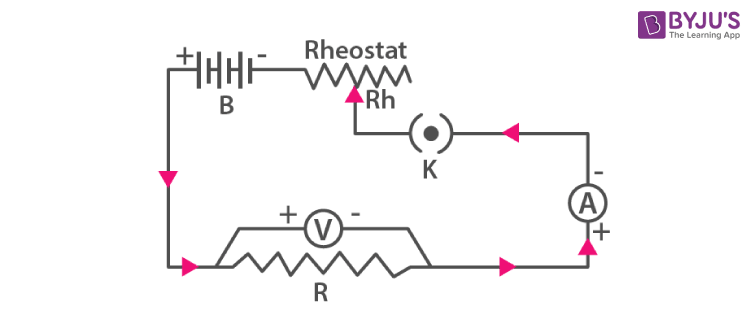Question: 9

(a) Name and state the law which relates the potential difference and current in a conductor.

(b) What is the necessary condition for a conductor to obey the law named above in part (a)?

Solution:

(a) The law is known as Ohm’s law. This law states that the current flowing through the conductor is directly proportional to the potential difference across its ends, provided that the physical conditions and the temperature of the conductor remain constant.

(b) The necessary condition for a conductor to obey Ohm’s law is that the temperature should remain constant.

Question: 10

(a) Draw a V-I graph for a conductor obeying Ohm’s law.

(b) What does the slope of V-I graph for a conductor represent?

Solution:

(a) V-I graph for a conductor obeying Ohm’s law is given below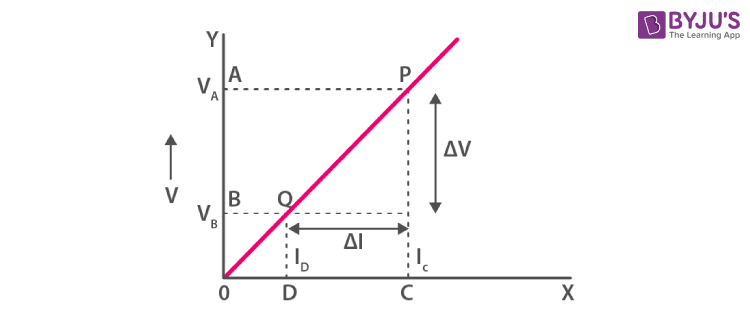(b) Slope of V-I graph for a conductor represents resistance.

Question: 11

Draw a I-V graph for a linear resistor. What does its slope represent?

Solution: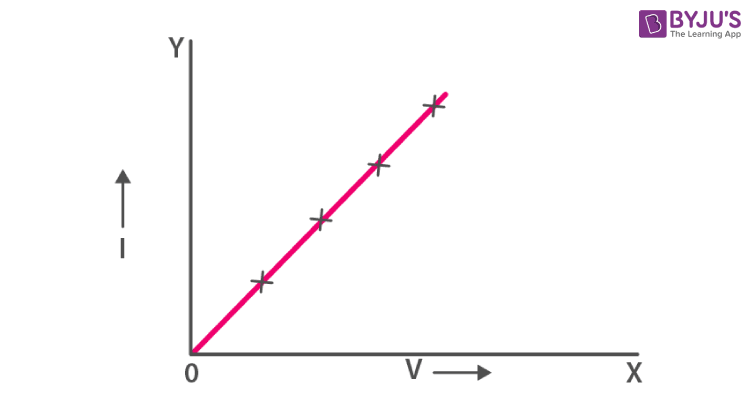I-V graph for a linear resistor

Slope of I-V graph: The slope of I-V graph is ΔI / ΔV

ΔI / ΔV is the reciprocal resistance of the conductor i.e.

Slope = ΔI / ΔV

= 1 / resistance of the conductor

Question: 12

What is an ohmic resistor? Give one example of an ohmic resistor. Draw a graph to show its current – voltage relationship. How is the resistance of the resistor determined from this graph?

Solution:

The conductors which obey the Ohm’s law are known as the ohmic resistors or linear resistances.

Examples: All metallic conductors such as silver, aluminium, copper, iron etc.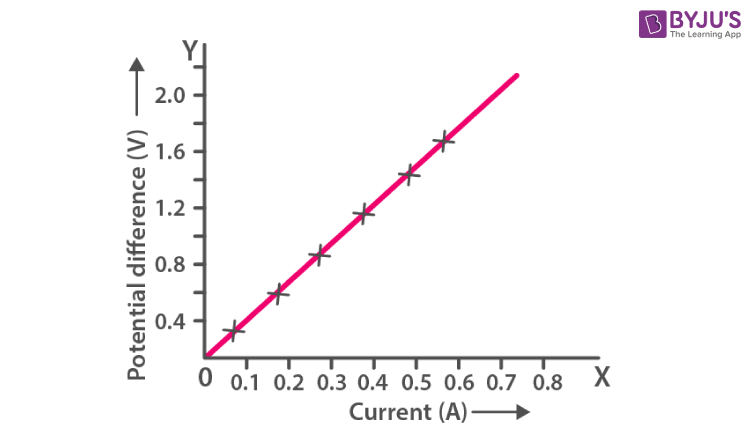Resistance is determined in the form of slope from the above graph

Question: 13

What are non-ohmic resistors? Give one example and draw a graph to show its current-voltage relationship.

Solution:

The conductors which do not obey the Ohm’s law are known as the non-ohmic resistors or non-linear resistances).

Examples: LED, solar cell, junction diode, etc.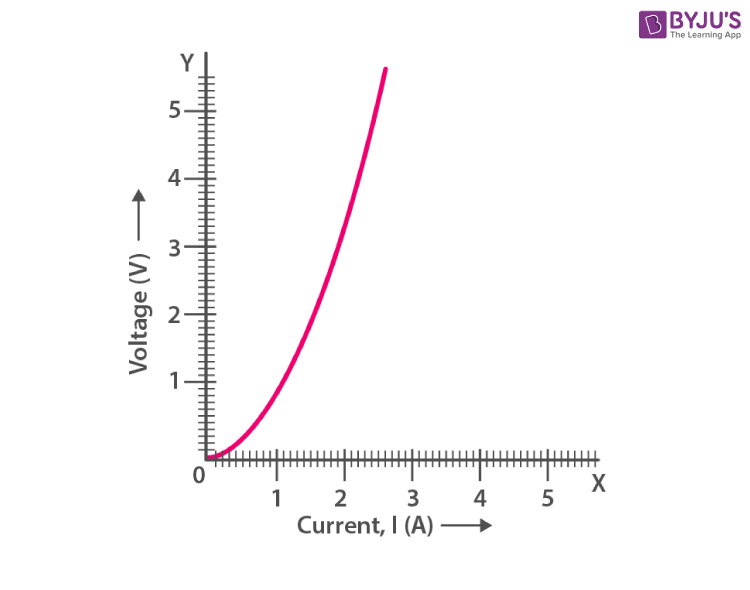V vs I for non-ohmic conductors

Question: 14

Give two differences between an ohmic and non-ohmic resistor

Solution:

 Ohmic resistor Non-ohmic resistor It obeys the Ohm’s law i.e., V / I is constant for all values of V or I. It does not obey the Ohm’s law i.e., V / I is not same for all values of V or I. The slope of V-I graph is same at all values of V or I at a given temperature The slope of V-I graph is different at different values of V or I even at a given temperature

Question: 15

Fig. below shows the I-V characteristic curves for two resistors. Identify the ohmic and non-ohmic resistors. Give a reason for your answer.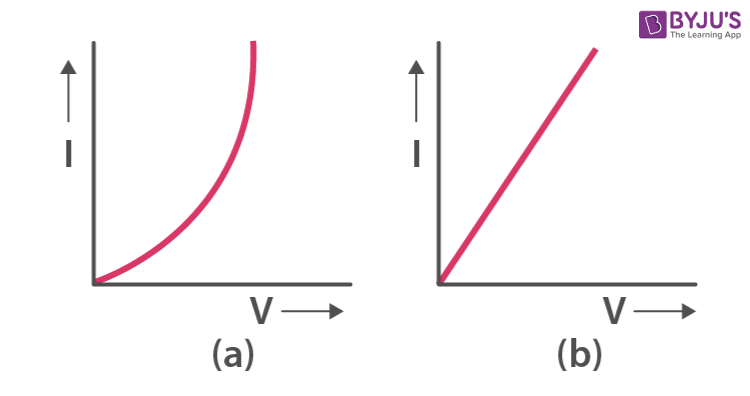Solution:

Graph (a) is non-ohmic resistor and Graph (b) is ohmic resistor

The I-V graph for (b) is a straight line or linear while the I-V graph for (a) is a curve

Question: 16

Draw a V – I graph for a conductor at two different temperatures. What conclusion do you draw from your graph for the variation of resistance of conductor with temperature?

Solution: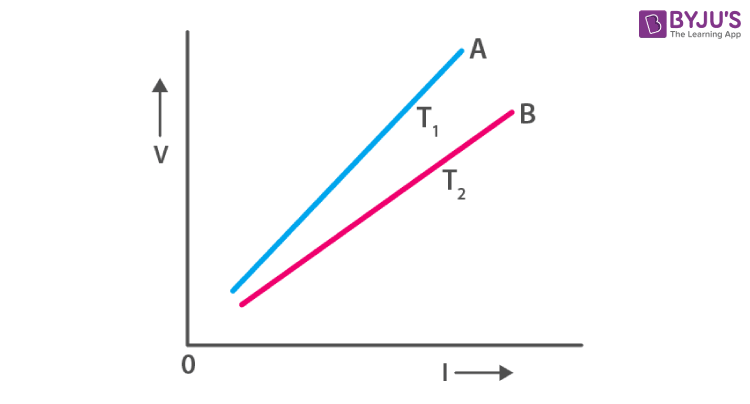In the given graph above T1 > T2. The straight line A is more steeper than the line B because the resistance of conductor is more at high temperature T1 than at low temperature T2. Hence we can conclude that resistance of a conductor increases with the increase in temperature.

Question: 17

(b) Two copper wires are of same length, but one is thicker than the other. Which will have more resistance?

Solution:

Resistance of a wire varies inversely as the area of cross section of the wire i.e.,

R ∝ 1 / a

R ∝ 1 / π2

Resistance of a wire is directly proportional to its length, i.e., R ∝ l and varies inversely as the area of cross section of the wire i.e., R ∝ 1 / a or R ∝ 1 / πr2

Since the resistance is inversely proportional to area of cross section, hence thin wire will have more resistance.

Question: 18

How does the resistance of a wire depend on its length? Give a reason of your answer.

Solution:

Resistance of a wire is directly proportional to the length of the wire.

R ∝ l

The resistance of conductor depends on the number of collisions which the electron suffer with the fixed positive ions while moving from one end to other end of the conductor. Therefore in a long conductor, the number of collisions of free electrons with the positive ions will be more when compared to a shorter conductor. Hence a longer conductor offers more resistance.

Question: 19

How does the resistance of a metallic wire depend on its temperature? Explain with reason.

Solution:

With the increase in temperature of a conductor, the random motion of electrons increases. This makes the number of collisions of electrons with the positive ions to increase. Hence, the resistance of a conductor increases with an increase in its temperature.

The resistance of filament of a bulb is more when it is glowing that is when it is at a high temperature as compared to when it is not glowing that is when it is cold.

Question: 20

Two wires, one of copper and other of iron, are of the same length and same radius. Which will have more resistance? Give reason.

Solution:

Iron has more resistivity as compared to copper which has less resistivity. So, greater the resistivity, the more the resistance is and the smaller the resistivity, the lesser the resistance. Hence, iron wire has more resistance than copper wire of the same length and same radius.

Question: 21

Name three factors on which the resistance of a wire depends and state how it is affected by the factors stated by you?

Solution:

The three factors on which the resistance of wire depends are as follows

(i) Resistance of a wire is directly proportional to its length that is

R ∝ l

(ii) Resistance of a wire varies inversely as the area of cross section of the wire. The resistance will be less when the area of cross section of the wire is more and vice versa

R ∝ 1 / a

(iii) Resistance of a conductor increases with an increase in its temperature. As a result the number of collisions increases.

(iv) Resistance depends on the nature of conductor since different substances have different concentration of free electrons. Substances which have a large concentration of electrons, offer less resistance and hence called good conductors and substances which have negligible concentration of free electrons offer very high resistance and are called insulators.

Question: 22

Define the term specific resistance and state its S.I. unit.

Solution:

Specific resistance of a material is the resistance of a wire of that material of unit length and unit area of cross section. The S.I. unit of specific resistance is ohm × metre

Question: 23

Write an expression connecting the resistance of a wire and specific resistance of its material. State the meaning of symbols used.

Solution:

The expression is

R = ρ l / a where

ρ = specific resistance of the material of conductor

R = resistance of conductor

l = length of conductor

A = area of cross section of conductor

Question: 24

State the order of specific resistance of (i) a metal, (ii) a semiconductor and (iii) an insulator.

Solution:

(i) The specific resistance for metals is low, since it allows most of current to pass through it.

(ii) The specific resistance for semiconductor is more than metals

(iii) The specific resistance for insulators is very high, since the current won’t pass through it

Question: 25

(a) Name two factors on which the specific resistance of a wire depends?

(b) Two wires A and B are made of copper. The wire A is long and thin while the wire B is Short and thick. Which will have more specific resistance?

Solution:

(a) Two factors on which the specific resistance of a wire depends are

(i) Material of the substance and

(ii) Temperature of the substance

(b) Both the wires will have the same specific resistance because the specific resistance depends on the material of the wire and not its dimensions.

Question: 26

Name a substance of which the specific resistance remains almost unchanged by the increase in temperature.

Solution:

The substance of which the specific resistance remains almost unchanged by the increase in temperature is manganin.

Question: 27

How does specific resistance of a semi-conductor change with the increase in temperature?

Solution:

With the increase in temperature, specific resistance of a semi-conductor decreases.

Question: 28

How does (a) resistance, and (b) specific resistance of a wire depend on its (i) length, and (ii) radius?

Solution:

(a) Resistance is directly proportional to length of the wire and inversely proportional to the square of radius of the wire

(b) Specific resistance of a wire do not depend on length and radius of the conductor that is independent of its length and radius.

Question: 29

(a) Name the material used for making the connection wires. Give reason for your answer.
(b) Why should a connection wire be thick?

Solution:

(a) Copper or aluminium are the materials used for making connection wires because they have small specific resistance and hence the wires of these materials possess negligible resistance

(b) The connection wires are made thick to consider their resistance as negligible to the flow of current through the circuit.

Question: 30

Name the material used for making a fuse wire. Give a reason.

Solution:

Alloy of lead and tin is used for making a fuse wire because it has high resistivity and low melting point.

MULTIPLE CHOICE TYPE

Question: 1

Which of the following is an ohmic resistance?

(a) LED

(b) Junction diode

(c) Filament of a bulb

(d) Nichrome wire

Solution:

An ohmic resistance is nichrome wire

Question: 2

For which of the following substances, resistance decreases with increase in temperature?

(a) Copper

(b) Mercury

(c) Carbon

(d) Platinum

Solution:

Resistance decreases with increase in temperature for carbon

NUMERICALS

Question: 1

In a conductor, 6.25 × 1016 electrons flow from its end A to B in 2 s. Find the current flowing through the conductor. (e = 1.6 × 10-19 C)

Solution:

Given

Number of electrons flowing through the conductor,

n = 6.25 × 1016 electrons.

Time taken to flow from A to B = 2 s and e = 1.6 × 10-19 C

Let I be the current flowing through the conductor

Now, I = ne / t

Therefore I = [(6.25 × 1016) (1.6 × 10-19)] / 2

I = 5 × 10-3 A or

I = 5 m A

Hence, 5 mA current flows from B to A

Question: 2

A current of 1.6 mA flows through a conductor. If charge of an electron is -1.6 x 10-19 coulomb, find the number of electrons that will pass each second through the cross section of that conductor.

Solution:

Given

Current, I = 1.6 m A or

I = 1.6 × 10-3 A

Charge, Q = -1.6 × 10-19 coulomb

t = 1 s

I = Q / t

Q = I × t

Q = 1.6 × 10-3 × 1

Number of electrons = 1.6 × 10-3 / 1.6 × 10-19

∴ Number of electrons = 1016

Question: 3

Find the potential difference required to flow a current of 200 mA in a wire of resistance 20 ohm.

Solution:

Given

Current I = 200 mA

I = 0.2 A

Resistance R = 20 ohm

Potential difference V =?

Using Ohm’s law

V = IR

V = 0.2 × 20

V = 4 V

Question: 4

An electric bulb draws 1.2 A current at 6.0 V. Find the resistance of filament of bulb while glowing.

Solution:

Given

Current I = 1.2 A

Potential difference or Voltage V = 6.0 V

Resistance R =?

From Ohm’s law

V = IR

R = V / I

R = 6 / 1.2

R = 5 Ohm

Question: 5

A car bulb connected to a 12 volt battery draws 2 A current when glowing. What is the resistance of the filament of the bulb? Will the resistance be more, same or less when the bulb is not glowing.

Solution:

Given

Potential difference or Voltage V = 12 V

Current I = 2 A

Resistance =?

According to Ohm’s law

V = IR

R = V / I

R = 12 / 2

R = 6 Ohm

Hence, when bulb is not glowing, resistance will be less

Question: 6

Calculate the current flowing through a wire of resistance 5 Ohm connected to a battery of potential difference 3 V.

Solution:

Given

Potential difference or Voltage V = 3 V

Resistance R = 5 Ohm

Current =?

From Ohm’s law

V = IR

I = V / R

I = 3 / 5

I = 0.6 A

Question: 7

In an experiment of verification of Ohm’s law, following observations are obtained.

 Potential difference V (in volt) 0.5 1 1.5 2 2.5 Current I (in amp) 0.2 0.4 0.6 0.8 1

Draw a characteristic V-I graph and use this graph to find:

(a) potential difference V when the current I is 0.5 A.

(b) current I when the potential difference V is 0.75 V.

(c) resistance in circuit

Solution: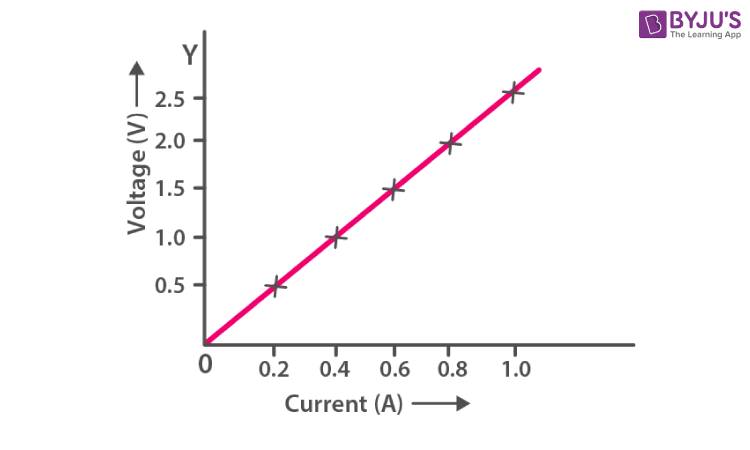(a) Potential difference is 1.25 V when the current is 0.5 A

(b) Current is 0.3 A when the potential difference is 0.75 V

(c) The graph is linear and thus resistance can be found from any value of the given table.

If V = 2.5 Volt then

Current I = 1.0 amp

Using Ohm’s law

R = V / I

R = 2.5 / 1.0

R = 2.5 Ohm

Question: 8

Two wires of the same material and same length have radii 1 mm and 2 mm respectively. Compare (i) their resistances (ii) their specific resistance.

Solution:

(i) For wire of radius r1

R1 = ρ (l / A1)

R1 = ρ (l / πr12)

(ii) For wire of radius r2

R2 = ρ (l / A2)

R2 = ρ (l / πr22)

∴ R1: R2 will be

ρ (l / πr12) : ρ (l / πr22)

= r22: r12

(ii) The resistivities of the two wires will be same because the material of the two wires is same. That is

ρ1: ρ2 = 1: 1

Question: 9

A given wire of resistance 1 Ohm is stretched to double its length. What will be its new resistance?

Solution:

Let ‘I’ be the length and ‘a’ be the area of cross section of the resistor with resistance, R = 1 Ohm

A given wire is stretched to double its length,

Therefore length l’ = 2l and area of cross section a’ = a / 2

Now Resistance (R’) = ρ l’/ a’

R’ = ρ 2l / a / 2

R’ = 4 ρ (1 / a)

R’ = 4R

R’ = 4 × 1

R’ = 4 Ohm

Question: 10

A wire of resistance 3 Ohm and length 10 cm is stretched to length 30 cm. Assuming that it has a uniform cross-section, what will be its new resistance?

Solution:

Given

Resistance R = 3 Ohm

Length l = 10 cm

The new length l’ = 30 cm = 3 × l

R = ρ (l / A)

New resistance

Stretching length will increase and area of cross section will decrease in same order

R’ = ρ (3l / A / 3)

Hence,

R’ = 9 ρ (l / A)

R’ = 9R

R’ = 9 × 3

R’ = 27 Ohm

Question: 11

A wire of resistance 9 Ohm having length 30 cm is tripled on itself. What is its new resistance?

Solution:

Given

Resistance R = 9 Ohm

Length l = 30 cm

New length l’ = 3 × l

New resistance R’=?

Area of cross section will also change in same order with change in length

R’ = ρ (l / 3 / 3A)

R’ = 1 / 9 (ρ l / A)

R’ = 1 / 9 R

R’ = 1 Ohm

Question: 12

What length of copper wire of specific resistance 1.7 x 10-8 ohm m and radius 1 mm is required so that its resistance is 1 ohm.

Solution:

Given

Resistance R = 1 ohm

Specific resistance = 1.7 × 10-8 ohm m

Radius r = 1 mm that is 10-3 m

Length l =?

R = ρ 1 / A

l = RA / ρ

l = Rπr2 / ρ

l = (1 × π × 10-6) / (1.7 × 10-8)

l = (1 × 3.14 × 10-6) / (1.7 × 10-8)

l = 1.847 × 102 m

l = 184.7 m

Question: 13

The filament of a bulb takes a current 100 mA when potential difference across it is 0.2 V. When the potential difference across it becomes 1.0 V, the current becomes 400 mA. Calculate the resistance of filament in each case and account for the difference.

Solution:

From Ohm’s law

V = IR

R = V / I

R1 = V1 / I1

R1 = 0.2 / 0.1

R1 = 2 ohm

Similarly

R2 = V2 / I2

R2 = 1 / 0.4

R2 = 2.5 ohm

∴ With increase in temperature resistance of the wire increases. Thus resistance of filament increases with the increase in temperature.

Exercise 8(b) Page no: 200

Question: 1

Explain the meaning of the terms e.m.f., terminal voltage and internal resistance of cell.

Solution:

e.m.f : When the cell is in open circuit, the potential difference between the terminals of the cell is called its electro-motive force or e.m.f

Terminal voltage: When the cell is in closed circuit, the potential difference between the electrodes of the cell is known as terminal voltage.

Internal resistance: The resistance offered by the electrolyte inside the cell, to the flow of current, is known as the internal resistance of the cell.

Question: 2

State two differences between the e.m.f. and terminal voltage of a cell.

Solution:

 e.m.f of cell Terminal voltage of cell 1. It is the characteristic of the cell, i.e., it does not depend on the amount of current drawn from the cell 1. It depends on the amount of current drawn from the cell. More the current drawn from the cell, less is the terminal voltage 2. It is equal to the terminal voltage when the cell is not in use, while greater than the terminal voltage when cell is in use 2. It is equal to the e.m.f. of cell when cell is not in use, while less than the e.m.f. when cell is in use.

Question: 3

Name two factors on which the internal resistance of a cell depends and state how does it depend on the factors stated by you.

Solution:

The factors on which the internal resistance of a cell depends are:

(i) The surface area of the electrodes – larger the surface area of electrodes, less is the internal resistance.

(ii) The distance between the electrodes – more the distance between the electrodes, greater is the internal resistance.

Question: 4

A cell of e.m.f. ε and internal resistance r is used to send current to an external resistance R. Write expressions for (a) the total resistance of circuit, (b) the current drawn from the cell, (c) the p.d. across the cell, and (d) voltage drop inside the cell.

Solution:

(a) The total resistance of circuit = R + r

(b) The current drawn from the cell

We know that,

ε = V + v

= IR + Ir

= I (R + r)

I = ε / (R + r)

(c) p.d. across the cell: [ε / (R + r)] × R

(d) voltage drop inside the cell: [ε / (R + r)] × r

Question: 5

A cell is used to send current to an external circuit. (a) How does the voltage across its terminal compare with its emf? (b) Under what condition is the emf of the cell equal to its terminal voltage?

Solution:

(a) When current is drawn from a cell, its terminal voltage V is less than its e.m.f.

∴ Terminal voltage < e.m.f.

(b) When no current is drawn, then the e.m.f. is equal to the terminal voltage.

Question: 6

Explain why the p.d. across the terminals of a cell is more in an open circuit and reduced in a closed circuit.

Solution:

The current flows through the circuit when the electric cell is in a closed circuit. There is a fall of potential across the internal resistance of the cell. Hence, the p.d. across the terminals in a closed circuit is less than the p.d. across the terminals in an open circuit by an amount equal to the potential drop across the internal resistance of the cell.

Question: 7

Write the expressions for the equivalent resistance R of three resistors R1, R2 and R3 joined in (a) parallel, (b) series.

Solution:

(a) Total resistance in parallel is given by

1 / R = 1 / R1 + 1 / R2 + 1 / R3

(b) Total resistance in series is given by

R = R1 + R2 + R3

Question: 8

How would you connect two resistors in series? Draw a diagram. Calculate the total equivalent resistance.

Solution: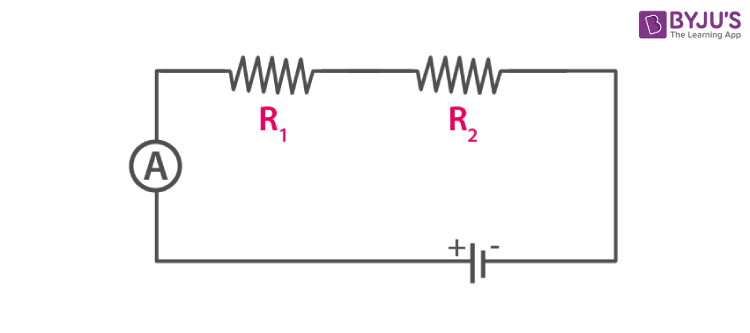If current I is drawn from the battery, the current will also be I through each resistor.

Applying Ohm’s law to the two resistors separately, we get,

V1 = IR1

V2 = IR2

V = V1 + V2

IR = IR1 + IR2

R = R1 + R2

Thus total resistance in series is

R = R1 + R2 + R3

Question: 9

Show by a diagram how two resistors R1 and R2 are joined in parallel. Obtain an expression for the total resistance of the combination.

Solution: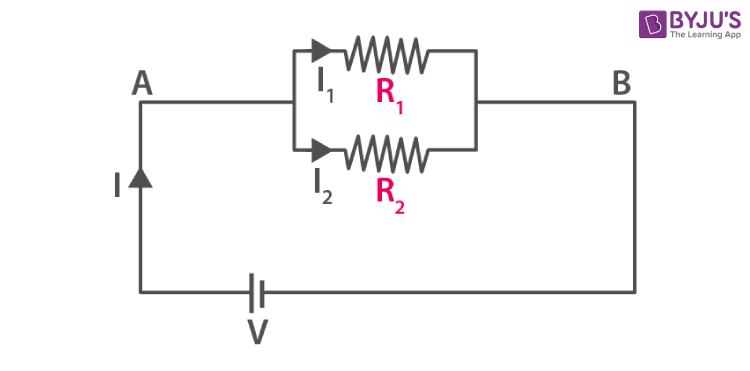Applying Ohm’s law separately to the two resistors, we have

I1 = V / R1

I2 = V / R2

I = I1 + I2

V / R = V / R1 + V / R2

1 / R = 1 / R1 + 1 / R2

Question: 10

State how are the two resistors joined with a battery in each of the following cases when:

(a) same current flows in each resistor

(b) potential difference is same across each resistor.

(c) equivalent resistance is less than either of the two resistances.

(d) equivalent resistance is more than either of the two resistances.

Solution:

(a) The two resistors are in series

(b) The two resistors are in parallel

(c) The two resistors are in parallel

(d) The two resistors are in series

Question: 11

The V-I graph for a series combination and for a parallel combination of two resistors is shown in fig. Which of the two, A or B, represents the parallel combination? Give a reason for your answer.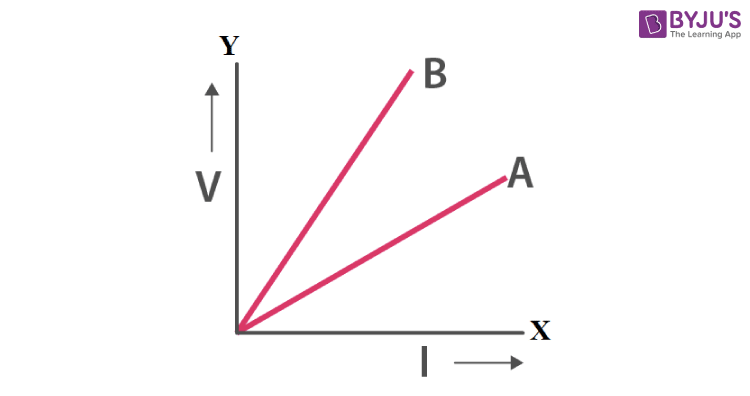Solution:

Change in V is less for the straight line A than for the straight line B (which means the straight line A is less steeper than B). Hence, the straight line A represents small resistance and the straight line B represents more resistance. The resistance decreases in parallel combination while the resistance increases in series combination. Thus the straight line A represents the parallel combination.

MULTIPLE CHOICE TYPE

Question: 1

In series combination of resistances:

(a) P.d. is same across each resistance

(b) Total resistance is reduced

(c) Current is same in each resistance

(d) All of the above are true

Solution:

Current is same in each resistance in series combination of resistances.

Question: 2

In parallel combination of resistances:

(a) P.D. is same across each resistance

(b) Total resistance is increased

(c) Current is same in each resistance

(d) All of the above are true

Solution:

P.D is same across each resistance in parallel combination of resistances

Question: 3

Which of the following combinations have the same equivalent resistance between X and Y?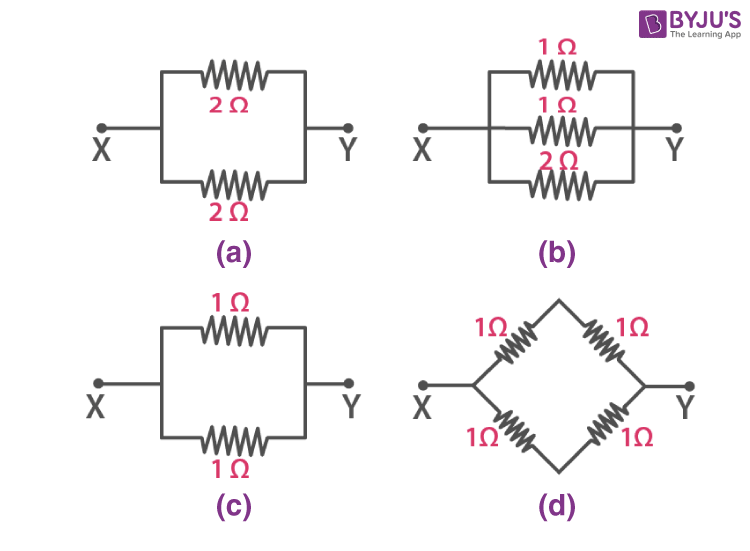Solution:

The resistors are connected in parallel in figure (a) between X and Y

Let R’ be their equivalent resistance

Now, 1 / R’ = 1 / 2 + 1 / 2

= 2 / 2 ohm or

R’ = 1 ohm 

A series combination of two 1 ohm resistors is in parallel with another series combination of two 1 ohm resistors in figure (d)

Series resistance of two 1 ohm resistors,

R = (1 + 1) ohm

R = 2 ohm

Therefore we can say that 2 ohm resistors are connected in parallel across X and Y

Let R’ be the net resistance across X and Y.

Then, 1 / R’ = 1 / 2 + 1 / 2

1 / R’ = 2 / 2 ohm or

R’ = 1 ohm 

Thus it is clear from equation  and  that the figures (a) and (d) have the same equivalent resistance between X and Y

NUMERICALS

Question: 1

The diagram in figure shows a cell of e.m.f. ε = 2 volt and internal resistance r = 1 ohm connected to an external resistance R = 4 ohm. The ammeter A measures the current in the circuit and the voltmeter V measures the terminal voltage across the cell. What will be the readings of the ammetere and voltmeter when (i) the key K is open, and (ii) the key K is closed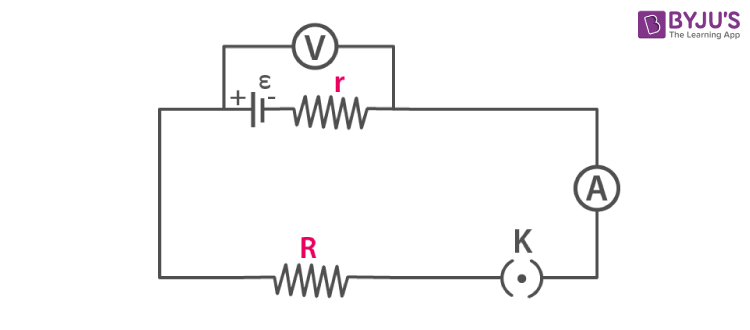Solution:

(i) Because of no current, ammeter reading = 0

Voltage V = ε – Ir

V = 2 – 0 × 1

V = 2 volt

I = ε / (R + r)

I = 2 / (4 + 1)

I = 2 / 5

I = 0.4 amp

Voltage V = ε – Ir

V = 2 – 0.4 × 1

V = 2 – 0.4

V = 1.6 V

Question: 2

A battery of e.m.f. 3.0 V supplies current through a circuit in which resistance can be changed. A high resistance voltmeter is connected across the battery. When the current is 1.5 A, the voltmeter reads 2.7 V. Find the internal resistance of the battery.

Solution:

Given

ε = 3 volt

I = 1.5 A

V = 2.7 V

V = ε – Ir

r = (e – V) / I

r = (3 – 2.7) / 1.5

r = 0.2 ohm

Question: 3

A cell of emf 1.8 V and internal resistance 2 ohm is connected in series with an ammeter of resistance 0.7 ohm and resistance of 4.5 ohm as shown in figure.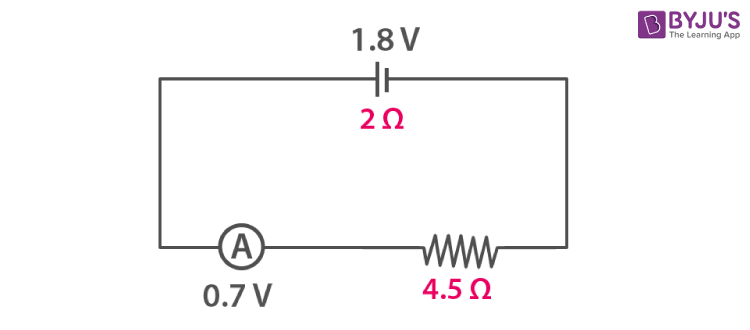(a) What would be the reading of the ammeter?

(b) What is the potential difference across the terminals of the cell?

Solution:

(a) ε = 1.8 V

Total resistance = 2 + 4.5 + 0.7

= 7.2 W

I =?

I = ε / R (total resistance)

I = 1.8 / 7.2

I = 0.25 A

(b) Current I = 0.25 A [calculated in (a) part]

Now, excluding internal resistance total resistance = 4.5 + 0.7

= 5.2 ohm

V = IR

V = 0.25 × 5.2

V = 1.3 V

Question: 4

A battery of emf 15 V and internal resistance 3 ohm is connected to two resistors of resistances 3 ohm and 6 ohm in series. Find:

(a) the current through the battery

(b) the p.d. between the terminals of the battery.

Solution:

(a) ε = 15 V

R = 6 + 3

R = 9 ohm

r = 3 ohm

I =?

I = ε / (R + r)

I = 15 / (9 + 3)

I = 15 / 12

I = 1.25 A

(b) Current I = 1.25 A [calculated in part (a)]

External resistance R = 6 + 3

R = 9 ohm

V = IR

V = 1.25 × 9

V = 11.25 V

Question: 5

A cell of e.m.f. ε and internal resistance r sends current 1.0 A when it is connected to an external resistance 1.9 ohm. But its sends current 0.5 A when it is connected to an external resistance of 3.9 ohm. Calculate the values of e and r.

Solution:

In first case

I = 1 A, R = 1.9 ohm

ε = I (R + r)

= 1 (1.9 + r)

= 1.9 + r 

In second case

I = 0.5 A, R = 3.9 ohm

ε = I (R + r)

= 0.5 (3.9 + r)

= 1.95 + 0.5r 

From equation  and 

1.9 + r = 1.95 + 0.5r

r = 0.05 / 0.5

r = 0.1 ohm

Now, substituting the value of r

ε = 1.9 + r

ε = 1.9 + 0.1

ε = 2 V

Question: 6

Two resistors having resistance 4 ohm and 6 ohm are connected in parallel. Find their equivalent resistance.

Solution

Let the equivalent resistance of the 4 ohm and 6 ohm resistors connected in parallel be R’

Then, 1 / R’ = 1 / 4 + 1 / 6

= (3 + 2) / 12

= 5 / 12 ohm or

R’ = 12 / 5

R’ = 2.4 ohm

Question: 7

Four resistors each of resistance 2 ohm are connected in parallel. What is the effective resistance?

Solution:

R1 = 2 ohm

R2 = 2 ohm

R3 = 2 ohm

R4 = 2 ohm

1 / R = 1 / R1 + 1 / R2 + 1 / R3 + 1 / R4

1 / R = 1 / 2 + 1 / 2 + 1 / 2 + 1 / 2

1 / R = 2

R = 0.5 ohm

Question: 8

You have three resistors of values 2 Ω, 3 Ω and 5 Ω . How will you join them so that the total resistance is less than 1 Ω? Draw diagram and find the total resistance.

Solution:

To get a total resistance less than 1 Ω, the three resistors should be connected in parallel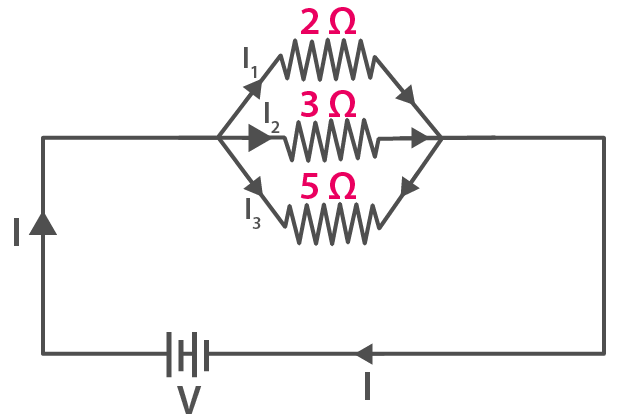Let the total resistance be R’

Then, 1 / R’ = 1 / 2 + 1 / 3 + 1 / 5

1 / R’ = (15 + 10 + 6) / 30

1 / R’ = 31 / 30 Ω or

R’ = 30 / 31

R’ = 0.97 Ω

Question: 9

Three resistors each of 2 W are connected together so that their total resistance is 3 W. Draw a diagram to show this arrangement and check it by calculation.

Solution:

A parallel combination of two resistors, in series with one resistor.

R1 = 2 ohm

R2 = 2 ohm

R3 = 2 ohm

1 / R’ = 1 / R1 + 1 / R2

1 / R’ = 1 / 2 + 1 / 2

1 / R’ = 1

R’ = 1 ohm

R = R’ + R3

R = 1 + 2

R = 3 ohm

Diagram is shown below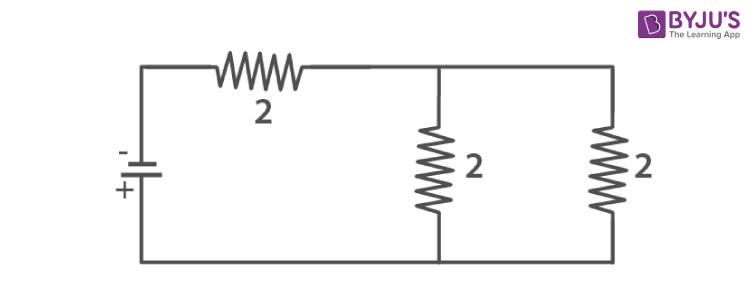Question: 10

Calculate the equivalent resistance between the points A and B in figure if each resistance is 2.0 Ω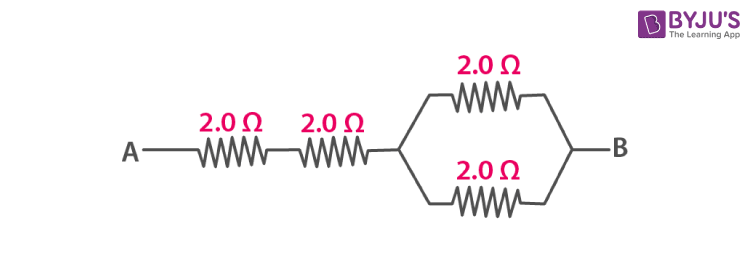Solution:

For a parallel resistances

Reff = (R1R2) / (R1 + R2)

Reff = (2 × 2) / (2 + 2)

Reff = 4 / 4

Reff = 1 Ω

Hence, total resistance = 2 + 2 + 1

= 5 Ω

Question: 11

A combination consists of three resistors in series. Four similar sets are connected in parallel. If the resistance of each resistor is 2 ohm, find the resistance of the combination.

Solution:

Resistance of each set:

r1 = 2 + 2 + 2 = 6 ohm

r2 = 2 + 2 + 2 = 6 ohm

r3 = 2 + 2 + 2 = 6 ohm

r4 = 2 + 2 + 2 = 6 ohm

Now, the above resistances are arranged in parallel

1 / r = 1 / r1 + 1 / r2 + 1 / r3 + 1 / r4

1 / r = 1 / 6 + 1 / 6 + 1 / 6 + 1 / 6

1 / r = 4 / 6

r = 6 / 4

r = 1.5 ohm

Question: 12

In the circuit shown below in figure, calculate the value of x if the equivalent resistance between the points A and B is 4 ohm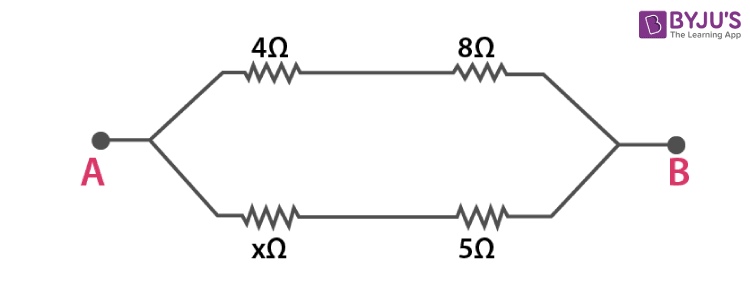Solution:

r1 = 4 ohm

r2 = 8 ohm

r3 = x ohm

r4 = 5 ohm

r = 4 ohm

r’ = r1 + r2

r’ = 4 + 8

r’ = 12 ohm

r’’ = r3 + r4

r’’ = (x + 5) ohm

1 / r = 1 / r’ + 1 / r’’

1 / 4 = 1 / 12 + 1 / (5 + x)

1 / 4 – 1 / 12 = 1 / (5 + x)

(3 – 1) / 12 = 1 / (5 + x)

2 / 12 = 1 / (5 + x)

1 / 6 = 1 / (5 + x)

x = 1 ohm

Question: 13

Calculate the effective resistance between the points A and B in the circuit shown in figure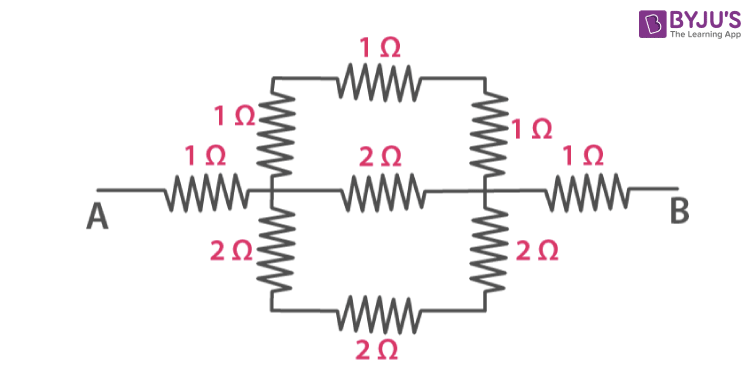Solution: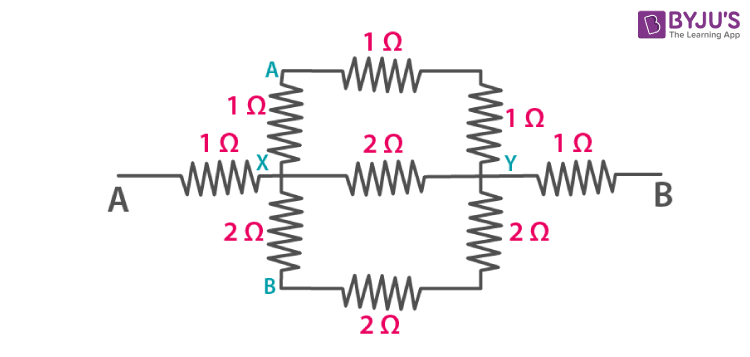In above figure,

Resistance between XAY = (1 + 1 + 1)

= 3 ohm

Resistance between XY = 2 ohm

Resistance between XBY = 6 ohm

Let the net resistance between the points X and Y be R’

Then, 1 / R’ = 1 / 2 + 1 / 3 + 1 / 6

1 / R’ = (3 + 2 + 1) / 6

1 / R’ = 6 / 6

1 / R’ = 1 ohm or

R’ = 1 ohm

Therefore, we can say that three 1 ohm resistors are connected in series between points A and B

Let the net resistance between points A and B be RAB

Then, RAB = (1 + 1 + 1) ohm

RAB = 3 ohm

Question: 14

A uniform wire with a resistance of 27 ohm is divided into three equal pieces and then they are joined in parallel. Find the equivalent resistance of the parallel combination.

Solution:

Since the wire is divided into three pieces, the new resistance = 27 / 3 = 9

Now, three resistance are joined in parallel

1 / r = 1 / r1 + 1 / r2 + 1 / r3

1 / r = 1 / 9 + 1 / 9 + 1 / 9

1 / r = 3 / 9

1 / r = 1 / 3

r = 3 ohm

Question: 15

A circuit consists of a 1 ohm resistor in series with a parallel arrangement of 6 ohm and 3 ohm resistors. Calculate the total resistance of the circuit. Draw a diagram of the arrangement.

Solution:

1 / r = 1 / 6 + 1 / 3

1 / r = 1 / 2

r = 2 ohm

R = 2 + 1

R = 3 ohm

Diagram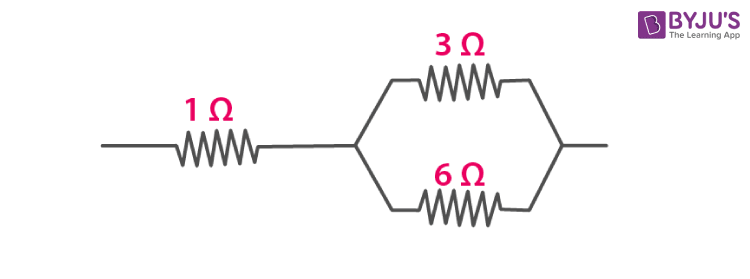Question: 16

Calculate the effective resistance between the points A and B in the network shown below in figure.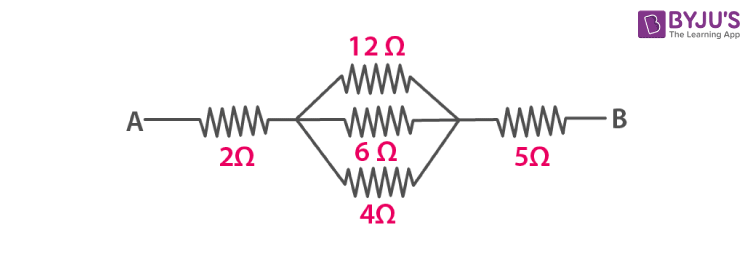Solution:

For parallel resistance

1 / R = 1 / 12 + 1 / 6 + 1 / 4

1 / R = (1 + 2 + 3) / 12

1 / R = 6 / 12

R = 12 / 6

R = 2 ohm

Now, all the resistances are in series

R = 2 + 2 + 5

R = 9 ohm

Question: 17

Calculate the equivalent resistance between the points A and B in figure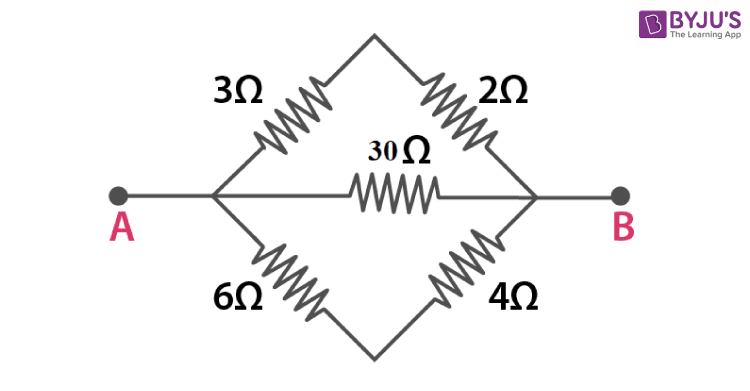Solution:

Given

R1 = 3 + 2 = 5 ohm

R2 = 30 W

R3 = 6 + 4 = 10 ohm

The resistors R1, R2 and R3 are connected in parallel

1 / R = 1 / R1 + 1 / R2 + 1 / R3

1 / R = 1 / 5 + 1 / 30 + 1 / 10

1 / R = (6 + 1 + 3) / 30

1 / R = 10 / 30

1 / R = 1 / 3

R = 3 ohm

Question: 18

In the network shown in adjacent figure, calculate the equivalent resistance between the points (a) A and B (b) A and C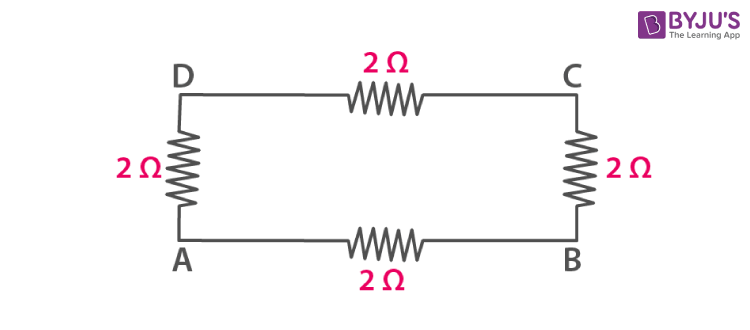Solution:

(a) R1 = 2 + 2 + 2

R1 = 6 ohm

R2 = 2 ohm

R1 and R2 are connected in parallel

1 / R = 1 / R1 + 1 / R2

1 / R = 1 / 6 + 1 / 2

1 / R = (1 + 3) / 6

1 / R = 4 / 6

R = 6 / 4

R = 1.5 ohm

(b) R1 = 2 + 2

R1 = 4 ohm

R2 = 2 + 2

R2 = 4 ohm

The resistors R1 and R2 are connected in parallel

1 / R = 1 / R1 + 1 / R2

1 / R = 1 / 4 + 1 / 4

1 / R = 2 / 4

1 / R = 1 / 2

R = 2 ohm

Question: 19

Five resistors, each of 3 ohm, are connected as shown in figure. Calculate the resistance (a) between the points P and Q, and (b) between the points X and Y.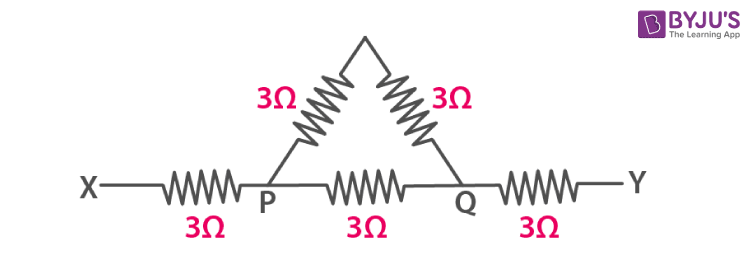Solution:

(a) R1 = 3 + 3

R1 = 6 ohm

R2 = 3 ohm

R1 and R2 are connected in parallel

1 / R = 1 / R1 + 1 / R2

1 / R = 1 / 6 + 1 / 3

1 / R = (1 + 2) / 6

1 / R = 3 / 6

1 / R = 1 / 2

R = 2 ohm

(b) We know that R = 2 ohm from the above calculation

R3 = 3 ohm

R4 = 3 ohm

R’ = R + R3 + R4

R’ = 2 + 3 + 3

R’ = 8 ohm

Question: 20

Two resistors of 2 ohm and 3 ohm are connected (a) in series, (b) in parallel, with a battery of 6.0 V and negligible internal resistance. For each case draw a circuit diagram and calculate the current through the battery.

Solution:

(a)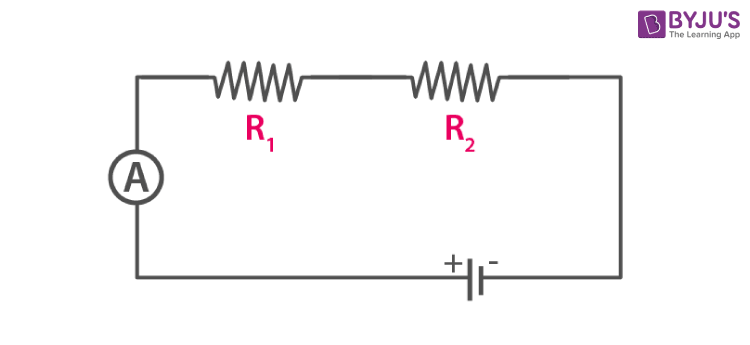R1 = 2 ohm

R2 = 3 ohm

R = R1 + R2

R = 2 + 3

R = 5 ohm

V = 6 V

Now, I = V / R

I = 6 / 5

I = 1.2 ohm

(b)Here, R1 and R2 are connected in parallel

1 / R = 1 / R1 + 1 / R2

1 / R = 1 / 2 + 1 / 3

1 / R = (3 + 2) / 6

1 / R = 5 / 6

R = 6 / 5

R = 1.2 ohm

V = 6 V

We know that,

I = V / R

I = 6 / 1.2

I = 5 A

Therefore in series: 1.2 A and in parallel: 5 A

Question: 21

A resistor of 6 ohm is connected in series with another resistor of 4 ohm. A potential difference of 20 V is applied across the combination. (a) Calculate the current in the circuit and (b) potential difference across the 6 ohm resistor.

Solution:

(a) To calculate current in the circuit

R1 = 6 ohm

R2 = 4 ohm

R = R1 + R2

R = 6 + 4

R = 10 ohm

V = 20 V

I = V / R

I = 20 / 10

I = 2 A

(b) To calculate the potential difference across the 6 ohm resistor

R = 6 ohm

I = 2 A

V =?

V = IR

V = 6 × 2

V = 12 V

Question: 22

Two resistors of resistance 4 Ω and 6 Ω are connected in parallel to a cell to draw 0.5 A current from the cell.

(a) Draw a labeled diagram of the arrangement

(b) Calculate current in each resistor.

Solution:

(a) Circuit diagram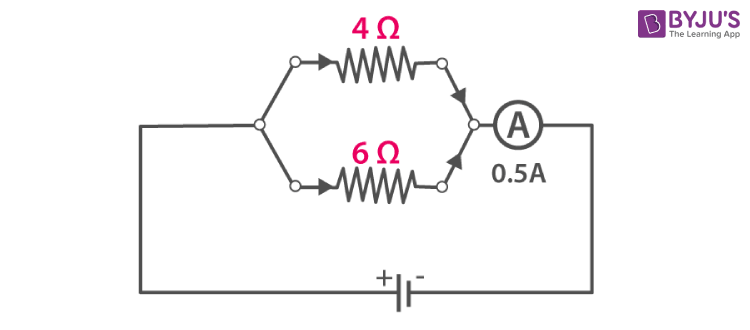(b) Equivalent resistance of the circuit

1 / R = 1 / 4 + 1 / 6

1 / R = (3 + 2) / 12

1 / R = 5 / 12

R = 12 / 5

R = 2.4 ohm

Thus, the e.m.f. of the cell is

V = IR

V = 0.5 × 2.4

V = 1.2 V

∴ Current through each resistor is

I4 = V / R4

I4 = 1.2 / 4

I4 = 0.3 A

I6 = V / R6

I6 = 1.2 / 6

I6 = 0.2 A

Hence, 0.3 A in 4 ohm and 0.2 A in 6 ohm

Question: 23

Calculate the current flowing through each of the resistors A and B in the circuit shown in figure?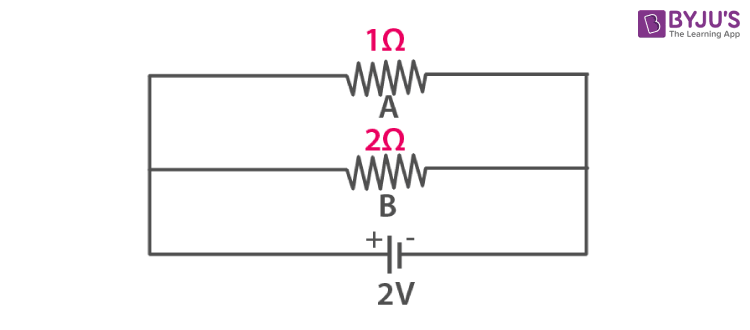Solution:

For resistor A

R = 1 ohm

V = 2 V

I = V / R

I = 2 / 1

I = 2 A

For resistor B

R = 2 ohm

V = 2 V

I = V / R

I = 2 / 2

I = 1 A

Hence, current flowing in resistor A is 2 A and current flowing in resistor B is 1 A

Question: 24

In figure, calculate :

(a) the total resistance of the circuit.

(b) the value of R, and

(c) the current flowing in R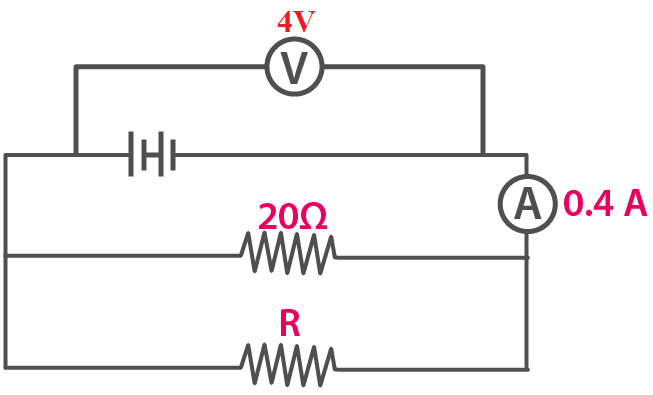Solution:

(a) To calculate the total resistance of the circuit

V = 4 V

I = 0.4 A

Total resistance R’ =?

R’ = V / I

R’ = 0.4 / 4

R’ = 10 ohm

(b) To calculate the value of R

R1 = 20 ohm

R’ = 10 ohm

1 / R’= 1 / R + 1 / R1

1 / 10 = 1 / R + 1 / 20

1 / R = 1 / 10 – 1 / 20

1 / R = (2 – 1) / 20

1 / R = 1 / 20

R = 20 ohm

(c) To calculate the current flowing in R

R = 20 ohm

V = 4 V

I = V / R

I = 4 / 20

I = 0.2 A

Question: 25

A particular resistance wire has a resistance of 3 ohm per meter. Find:

(a) The total resistance of three lengths of this wire each 1.5 m long, in parallel.

(b) The potential difference of the battery which gives a current of 2 A in each of the 1.5 m length when connected in the parallel to the battery (assume that resistance of the battery is negligible).

(c) The resistance of 5 m length of a wire of the same material, but with twice the area of cross section.

Solution:

(a) Resistance of wire per meter = 3 ohm

So, resistance of three lengths of this wire each 1.5 m long = 3 × 1.5 = 4.5 W

1 / R = 1 / 4.5 + 1 / 4.5 + 1 / 4.5

1 / R = 3 / 4.5

R = 1.5 ohm

(b) I = 2 A

V = IR

V = 2 × 4.5

V = 9 V

(c) R = 3 ohm for 1 meter wire

For 5 m

R = 3 × 5

R = 15 ohm

Here the area is twice i.e 2 A and Resistance is inversely proportional to area. Thus resistance becomes half

R = 15 / 2

R = 7.5 ohm

Question: 26

A cell supplies a current of 1.2 A through two 2 ohm resistors connected in parallel. When resistors are connected in series, it supplies a current of 0.4 A. Calculate: (i) the internal resistance and (ii) e.m.f. of the cell.

Solution:

In parallel R = 1 / 2 + 1 / 2 = 1 ohm

I = 1.2 A

ε = I (R + r)

ε = 1.2 (1 + r)

ε = 1.2 + 1.2r

In series

R = 2 + 2

R = 4 ohm

I = 0.4 A

ε = I (R + r)

ε = 0.4 (4 + r)

ε = 1.6 + 0.4r

This means:

1.2 + 1.2r = 1.6 + 0.4r

1.2r – 0.4r = 1.6 – 1.2

0.8r = 0.4

r = 0.4 / 0.8

r = 0.5 ohm

(i) Internal resistance r = 0.5 ohm

(ii) ε = I(R + r)

ε = 1.2 (1 + 0.5)

ε = 1.8 V

Question: 27

A battery of emf 15 V and internal resistance 3 ohm is connected to two resistors 3 ohm and 6 ohm connected in parallel. Find (a) the current through the battery (b) p.d. between the terminals of the battery (c) the current in 3 ohm resistor (d) the current in 6 ohm resistor.

Solution:

(a) In parallel

1 / R = 1 / 3 + 1 / 6

1 / R = (2 + 1) / 6

1 / R = 3 / 6

1 / R = 1 / 2

R = 2 ohm

r = 3 W

ε = 15 V

ε = I (R + r)

15 = I (2 + 3)

I = 15 / 5

I = 3 A

(b) V =?

R = 2 ohm

V = IR

V = 3 × 2

V = 6 V

(c) V = 6 V

R = 3 ohm

I = V / R

I = 6 / 3

I = 2 A

(d) R = 6 ohm

V = 6 V

I = V / R

I = 6 / 6

I = 1 A

Question: 28

The circuit diagram in figure shows three resistors 2 ohm, 4 ohm and R ohm connected to a battery of e.m.f. 2 V and internal resistance 3 ohm. If main current of 0.25 A flows through the circuit, find:

(a) the p.d. across the 4 ohm resistor

(b) the p.d. across the internal resistance of the cell,

(c) the p.d. across the R ohm or 2 ohm resistor, and

(d) the value of R.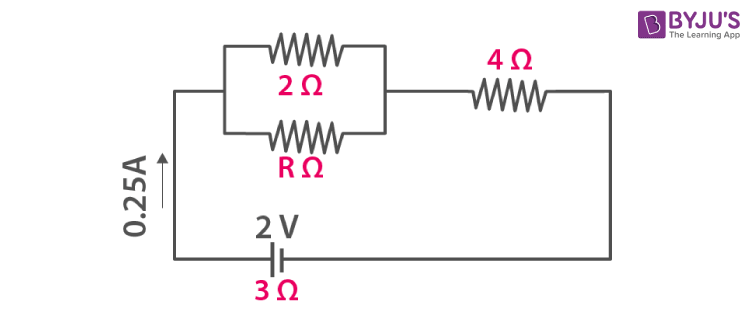Solution:

(a) To calculate the p.d. across the 4 ohm resistor

R = 4 ohm

I = 0.25 A

V = IR

V = 0.25 × 4

V = 1 V

(b) To calculate the p.d. across the internal resistance of the cell

Internal resistance r = 3 ohm

I = 0.25 A

V = IR

V = 0.25 × 3

V = 0.75 V

(c) To calculate the p.d. across the 2 ohm resistor

Effective resistance of parallel combination of 2 ohm resistances = 1 ohm

V = I / R

V = 0.25 / 1

V = 0.25 V

(d) To calculate the value of R

I = 0.25 A

ε = 2 V

r = 3 ohm

ε = I (R’ + r)

2 = 0.25 (R’ + 3)

R’ = 5 W

[2R / 2 + R] + 4 = 5

R = 2 ohm

Question: 29

Three resistors of 6.0 ohm, 2.0 ohm and 4.0 ohm are joined to an ammeter A and a cell of emf 6.0 V as shown in figure. Calculate:

(a) the efective resistance of the circuit.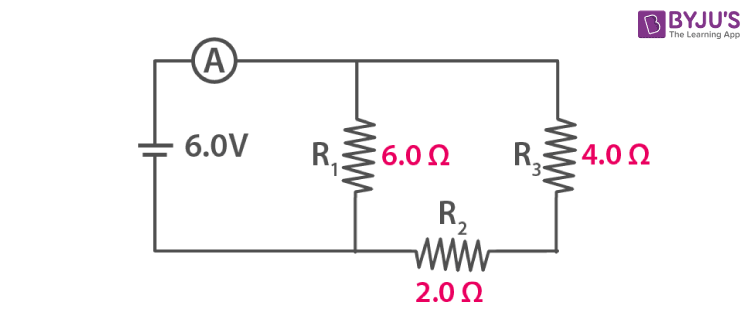Solution:

(a) R1 = 6 W

R’ = R2 + R3

R’ = 2 + 4

R’ = 6 W

R1 and R’ are connected in parallel

1 / R = 1 / R1 + 1 / R’

1 / R = 1 / 6 + 1 / 6

1 / R = 2 / 6

1 / R = 1 / 3

R = 3 ohm

(b) R = 3 ohm

V = 6 V

I =?

I = V / R

I = 6 / 3

I = 2 A

Question: 30

The diagram below in Fig., shows the arrangement of five different resistances connected to a battery of e.m.f. 1.8 V. Calculate: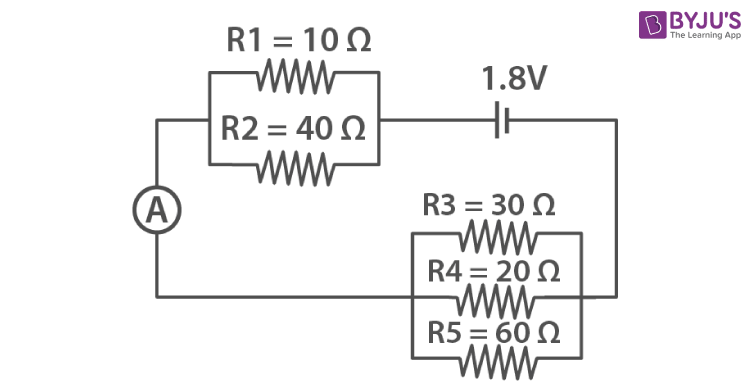a. The total resistance of the circuit

b. The reading of ammeter A.

Solution: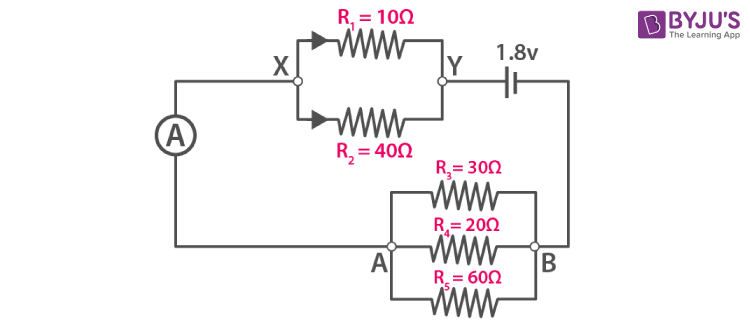(a) In the above figure,

Let RXY be the resistance between X and Y

Then, 1 / RXY = 1 / 10 + 1 / 40

1 / RXY = (4 + 1) / 40

1 / RXY = 5 / 40 ohm

Or RXY = 8 ohm

Let the net resistance between points A and B be RAB

Then, 1 / RAB = 10 ohm

∴ The total resistance of the circuit = 8 ohm + 10 ohm

= 18 ohm

(b) Current I = Voltage / Total resistance

I = 1.8 / 18 A

I = 0.1 A

Hence, the reading of ammeter is 0.1 A

Question: 31

A cell of e.m.f. 2 V and internal resistance 1.2 Ω is connected to an ammeter of resistance 0.8 Ω and two resistors of 4.5 Ω and 9 Ω as shown in fig.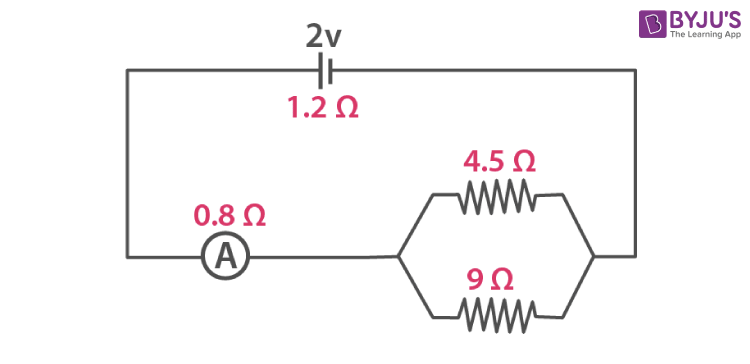Find:

(a) The reading of the ammeter,

(b) The potential difference across the terminals of the cells, and

(c) The potential difference across the 4.5 ohm resistor.

Solution:

The total resistance of the circuit is

Req = Rcell + Rammeter + R1 || R2

∴ Req = 1.2 + 0.8 + (R1R2) / R1 + R2

∴Req = 2 + (4.5 × 9) / 4.5 + 9

Req = 2 + 40.5 / 13.5

∴Req = 5 ohm

(a) The current through the ammeter is

I = Ecell / Req

I = 2 / 5

I = 0.4 A

(b) The potential difference across the ends of the cells is

Vcell = Ecell – IRcell

Vcell = 2 – 0.4 × 1.2

Vcell = 2 – 0.48

∴Vcell = 1.52 V

(c) The potential difference across the 4.5 ohm resistor is

V4.5 = Vcell – Vammeter

V4.5 = 1.52 – 0.4 × 0.8

V4.5 = 1.52 – 0.32

∴ V4.5 = 1.2 V

Exercise 8(C) Page No: 211

Question: 1

Write an expression for the electrical energy spent in flow of current through an electrical appliance in terms of current, resistance and time.

Solution:

The expression for the electrical energy spent in flow of current through an electrical appliance in terms of current, resistance and time is

Electrical energy, W = I2Rt joule

Question: 2

Write an expression for the electrical power spent in flow of current through a conductor in terms of (a) resistance and potential difference, (b) current and resistance.

Solution:

(a) Expression for electrical power spent in flow of current through a conductor in terms of resistance and potential difference is

Electrical Power, P = V2 / R

(b) Expression for electrical power spent in flow of current through a conductor in terms of current and resistance is

Electrical Power, P = I2R

Question: 3

Electrical power P is given by the expression P = (Q × V) ÷ time

(a)What do the symbols Q and V represent?

(b)Express the power P in terms of current and resistance explaining the meanings of symbols used there in.

Solution:

(a) The symbol Q represents Charge and the symbol V represents Voltage

(b) Electrical Power, P = I2R

-where I represents current and R represents resistance

Question: 4

Name the S.I. unit of electrical energy. How is it related to Wh?

Solution:

Joule is the S.I. unit of electrical energy. It is related to Wh as

1 Wh = 3600 J

Question: 5

Explain the meaning of the statement ‘the power of an appliance is 100 W’.

Solution:

The power of an appliance is 100 W. This means that 100 J of electrical energy is consumed by the appliance in 1 second.

Question: 6

State the S.I. unit of electrical power.

Solution:

Watt is the S.I. unit of electrical power.

Question: 7

(i)State and define the household unit of electricity.

(ii)What is the voltage of the electricity that is generally supplied to a house?

(iii) What is consumed while using different electrical appliances, for which electricity bills are paid?

Solution:

(i) Kilowatt hour (kWh) is the household unit of electricity. The electrical energy consumed by an electrical appliance of power 1 kW when it is used for one hour is One kilowatt hour (kWh)

(ii) The voltage of the electricity that is generally supplied to a house is 220 volt.

(iii) The electrical energy is consumed while using different electrical appliances, for which electricity bills are paid

Question: 8

Name the physical quantity which is measured in (i) kW, (ii) kWh. (iii) Wh

Solution:

(i) The quantity which is measured in kW is electrical power

(ii) The quantity which is measured in kWh is electrical energy

Question: 9

Define the term kilowatt – hour and state its value in S.I. unit.

Solution:

The electrical energy consumed by an electrical appliance of power 1 kW when it is used for one hour is one kilowatt hour (kWh). Its value in S.I. unit is 1kWh = 3.6 × 106 J

Question: 10

How do kilowatt and kilowatt-hour differ?

Solution:

Kilowatt- hour is the unit of electrical energy whereas the kilowatt is the unit of electrical power.

Question: 11

Complete the following:

(a) 1 kWh = (1 volt × 1 ampere × ……..) / 1000

(b)

1 kWh= ________ J

Solution:

(a) 1 kWh = (1 volt × 1 ampere × 1 hour) / 1000

(b) 1 kWh = 3.6 × 106 J

Question: 12

What do you mean by power rating of an electrical appliance? How do you use it to calculate (a) the resistance of the appliance and (b) the safe limit of the current in it, while in use?

Solution:

The power rating is an electrical appliance such as electric bulb, geyser etc. is rated with power (P) and voltage (V). If an electric bulb is rated as 50 W – 220 V, it means that when the bulb is lighted on a 220 V supply, 50 W electrical power is consumed by it.

(a) To calculate the resistance of the appliance, the expression is:

Resistance, R = V2 / P

(b) The safe limit of current in it, while in use is

I = P / V

Question: 13

An electric bulb is rated ‘100 W, 250 V’. What information does this convey?

Solution:

An electric bulb is rated ‘100 W, 250 V’ means that if the bulb is lighted on a 250 V supply, it consumes 100 W electric power (which means 100 J of electrical energy is converted into the light and heat energy in 1 second in the filament).

Question: 14

List the names of three electrical gadgets used in your house. Write their power, voltage rating and approximate time for which each one is used in a day. Hence find the electrical energy consumed by each in a month of 30 days.

Solution:

 Appliance Power (in watt) Voltage (in volts) Time (hours) Electrical energy (E = P × t) Television set Refrigerator Fluorescent tube 120 150 40 220 220 220 4 24 12 0.48 kWh 3.6 kWh 0.48 kWh

Question: 15

Two lamps, one rated 220 V, 50 W and the other rated 220 V, 100 W, are connected in series with mains of voltage 220 V. Explain why does the 50 W lamp consume more power.

Solution:

Resistance of 220 V, 50 W lamp is

R1 = V2 / P1

R1 = (220)2 / 100

R1 = 968 ohm

Resistance of 220 V, 100 W lamp is

R2 = V2 / P2

R2 = (220)2 / 100

R2 = 484 ohm

Same current I passes through each lamp because the two lamps are connected in series.

Power consumed in 220 V, 50 W lamp is

P1 = I2R1

Power consumed in 220 V, 100 W lamp is

P2 = I2R2

Here, R1 > R2

So, P1 > P2

Thus 50 W lamp consumes more power

Question: 16

Name the factors on which the heat produced in a wire depends when current is passed in it, and state how does it depend on the factors stated by you.

Solution:

The amount of heat produced in a wire on passing current through it, depends on the following three factors.

(i) The amount of current passing through the wire

(ii) The resistance of wire and

(iii) The time for which current is passed in the wire

(i) Dependence of heat produced on the current in wire: The amount of heat H produced in the wire is directly proportional to the square of current I passing through the wire, i.e., H ∝ I2

(ii) Dependence of heat produced on the resistance of wire: The amount of heat H produced in the wire is directly proportional to the resistance R of the wire, i.e., H ∝ R

(iii) Dependence of heat produced on the time: The amount of heat H produced in a wire is directly proportional to the time t for which current is passed in the wire i.e., H ∝ t

MULTIPLE CHOICE TYPE

Question: 1

When a current I flows through a resistance R for time t, the electrical energy spent is:

(a) IRt

(b) I2Rt

(c) IR2t

(d) I2R / t

Solution:

The electrical energy spent when a current I flows through a resistance R for time t is I2Rt

Question: 2

An electrical appliance has a rating 100 W, 120 V. The resistance of element of appliance when in use is:

(a) 1.2 ohm

(b) 144 ohm

(c) 120 ohm

(d) 100 ohm

Solution:

The resistance of element of appliance when in use is 144 ohm

NUMERICALS

Question: 1

An electric bulb of resistance 500 ohm draws current 0.4 A from the source. Calculate: (a) the power of bulb and (b) the potential difference at its end.

Solution:

Given

Resistance of electric bulb (R) = 500 ohm

Current drawn from the source (I) = 0.4 A

(a) Power of the bulb (P) = VI

V = I × R

V = 0.4 × 500

V = 200 V

(b) The potential difference at its end is 200 V

Therefore,

Power (P) = VI

P = 200 × 0.4

P = 80 W

Hence, the power of the bulb is 80 Watt

Question: 2

A current of 2 A is passed through a coil of resistance 75 Ω for 2 minutes. (a) How much heat energy is produced? (b) How much charge is passed through the resistance?

Solution:

Given,

Current (I) = 2 A

Resistance, R = 75 Ω

Time, t = 2 min or 120 s

(a) Heat produced, H = I2Rt or

H = (2)2 (75) (120) J = 36000 J

(b) Charge passed through the resistance, Q = It or

Q = (2) (120) C

Q = 240 C

Question: 3

Calculate the current through a 60 W lamp rated for 250 V. If the line voltage falls to 200 V, how is power consumed by the lamp affected?

Solution:

Given,

Power, P = 60 W

Voltage, V = 250 V

We know that,

Power, P = VI

I = P / V

I = 60 / 250

I = 0.24 A

Resistance of lamp R = V2 / P

R = (250)2 / 60

R = 1041.6 ohm

If voltage falls to 200 V, then the power consumed will be

P = V2 / R

P = (200)2 / 1041.6

P = 38.4 W

Hence, consumed reduces to 38.4 W

Question: 4

An electric bulb is rated ‘100 W, 250 V’. How much current will the bulb draw if connected to a 250 V supply?

Solution:

Given,

Power, P = 100 W

Voltage, V = 250 V

We know that,

Power, P = VI

I = P / V

I = 100 / 250

I = 0.4 A

Question: 5

An electric bulb is rated at 220 V, 100 W. (a) What is its resistance? (b) What safe current can be passed through it?

Solution:

(a) Given,

Power, P = 100 W

Voltage, V = 220 V

We know that,

Power, P = V2 / R

R = (220)2 / 100

R = 484 ohm

(b) The safe limit of current that can be passed through it is

I = P / V

I = 100 / 220

I = 0.45 A

Question: 6

A bulb of power 40 W is used for 12.5 h each day for 30 days. Calculate the electrical energy consumed.

Solution:

Energy consumed for each day, E = P × t

E = 40 × 12.5

E = 500 Wh

Energy consumed for 30 days

E = 500 × 30

E = 15000 Wh

E = 15 kWh

Question: 7

An electric press is rated ‘750 W, 230 V’. Calculate the electrical energy consumed by the press in 16 hours

Solution:

Energy, E = Power × time

E = 750 × 16

E = 12000 Wh

E = 12 kWh

Question: 8

An electrical appliance having a resistance of 200 ohm is operated at 200 V. Calculate the energy consumed by the appliance in 5 minutes (i) in joule, (ii) in kWh

Solution:

Given,

Resistance, R = 200 ohm

Voltage, V = 200 V

Time, t = 5 minutes

t = 5 × 60 sec

t = 300 sec

As we know,

Energy, E = V2t / R

(i) In joules

E = [(200)2 × 300] / 200

E = 60000 J

(ii) In kWh

As 1 kWh = 3.6 × 106 J

1 J = 1 / 3.6 × 106 kWh

60000 J = 60000 / 3.6 × 106

60000 J = 0.0167 kWh

Question: 9

A bulb rated 12 V, 24 W operates on a 12 volt battery for 20 minutes. Calculate:

(i) the current flowing through it, and

(ii) the energy consumed.

Solution:

Given,

Power, P = 24 W

Voltage, V = 12 V

Current, I =?

We know that,

Power, P = VI

(i) The current flowing through it is

I = 24 / 12

I = 2 A

(ii) Energy, E = P × t

E = 24 × 20 × 60

E = 28,800 J

Question: 10

A current of 0.2 A flows through a wire whose ends are at a potential difference of 15 V. Calculate:

(i)The resistance of the wire, and

(ii)The heat energy produced in 1 minute.

Solution:

Given,

Current, I = 0.2 A

Potential difference, V = 15 V

Time, t = 60 sec

As V = IR

(a) To calculate the resistance of the wire

R = V / I

R = 15 / 0.2

R = 75 ohm

(b) To calculate the heat energy produced in 1 minute

Heat energy, H = I2Rt

H = (0.2)2 × 75 × 60

H = 180 J

Question: 11

What is the resistance, under normal working conditions, of an electric lamp rated at ‘240 v’, 60 W? If two such lamps are connected in series across a 240 V mains supply, explain why each one appears less bright.

Solution:

Given,

Voltage, V = 240 V

Power, P = 60 W

We know that,

P = V2 / R

R = V2 / P

R = (240)2 / 60

R = 960 ohm

I = P / V

I = 60 / 240

I = 0.25 A

Thus when one lamp is connected across the mains, it draws 0.25 A current. If two such lamps are connected in series across the mains, current through each bulb becomes

[240 / (960 + 960) ohm] = 0.125 A (i.e., current is halved.) Hence, heating (= I2Rt) in each bulb becomes one fourth, so each bulb appears less bright.

Question: 12

Two bulbs are rated 60 W, 220 V and 60 W, 110 V, respectively. Calculate the ratio of their resistances.

Solution:

Given,

Voltage, V1 = 220 V

V2 = 110 V

Power, P1 = P2 = P = 60 W

We know that, R = V2 / P

R1 = V12 / P

R1 = (220)2 / 60

R2 = V22 / P

R2 = (110)2 / 60

Now, dividing R1 and R2 we get,

R1 / R2 = [(220)2 / 60] / [(110)2 / 60]

R1 / R2 = 4 / 1

R1: R2 = 4: 1

Question: 13

An electric bulb is rated 250 W, 230 V.

(i) the energy consumed in one hour, and

(ii) the time in which the bulb will consume 1.0 kWh energy when connected to 230 V mains?

Solution:

Given,

Power, P = 250 W

Voltage, V = 230 V

(i) Energy, E = P × t

Time, t = 1 × 60 × 60

t = 3600 sec

We know that,

E = 250 × 3600

E = 9 × 105 J

(ii) 1000 Wh = 250 × t

time, t = 1000 / 250

t = 4 hours

Question: 14

Three heaters each rated 250 W, 100 V are connected in parallel to a 100 V supply. Calculate:

(i)The total current taken from the supply,

(ii)The resistance of each heater, and

(iii)The energy supplied in kWh to the three heaters in 5 hours.

Solution:

Given,

Power, P = 250 W

Voltage, V = 100 V

(i) Current through each heater, I =?

As P = VI

I = P / V

I = 250 / 100

I = 2.5 A

∴ Current taken for the three heaters

= 3 × 2.5

= 7.5 A

(ii) Resistance for each heater, R = V / I

R = 100 / 2.5

R = 40 ohm

(iii) Time for which energy is supplied, t = 5 h

Energy, E = P × t

E = 250 × 5

E = 1250 Wh

E = 1.25 kWh

Energy for three heaters = 3 × 1.25

= 3.75 kWh

Question: 15

A bulb is connected to a battery of p.d. 4 V and internal resistance 2.5 ohm. A steady current of 0.5 A flows through the circuit. Calculate:

(i)The total energy supplied by the battery in 10 minutes,

(ii)The resistance of the bulb, and

(iii)The energy dissipated in the bulb in 10 minutes.

Solution:

Given,

Voltage, V = 4 V

Resistance of the battery, RB = 2.5 ohm

Current, I = 0.5 A

(i) Energy supplied by the battery, E = V2t / R

t = 10 × 60

t = 600 sec

R = V / I

R = 4 / 0.5

R = 8 ohm

E = [(4)2 × 600] / 8

E = 1200 J

(ii) Total resistance, R = 8 ohm

Resistance of the battery, RB = 2.5 ohm

Resistance of the bulb, Rb = 8 – 2.5 ohm

Rb = 5.5 ohm

(iii) Energy dissipated in the bulb in 10 min, E = I2Rt

E = (0.5)2 × 5.5 × 600

E = 825 J

Question: 16

Two resistors A and B of 4 ohm and 6 ohm, respectively are connected in parallel. The combination is connected across a 6 volt battery of negligible resistance. Calculate: (i) the power supplied by the battery, (ii) the power dissipated in each resistor.

Solution:

Given,

Resistance, RA = 4 ohm

Resistance, RB = 6 ohm

Voltage, V = 6 V

(i) Since the resistances are connected in parallel

Equivalent Resistance, 1 / R = 1 / RA + 1 / RB

1 / R = 1 / 4 + 1 / 6

1 / R = 10 / 24

R = 2.4 ohm

We know that,

Power, P = V2 / R

P = (6)2 / 2.4

P = 15 W

(ii) Power dissipation across each resistor, P = VI

Current across resistor RA, IA = V / RA

IA = 6 / 4 = 1.5 A

Power dissipation across resistor RA,

P = VIA

P = 6 × 1.5

P = 9 W

(iii) Current across resistor RB, IB = V / RB

IB = 6 / 6

IB = 1 A

Power dissipation across resistor RB,

P = VIB

P = 6 × 1

P = 6 W

Question: 17

A battery of e.m.f. 15 V and internal resistance 2 ohm is connected to two resistors of resistances 4 ohm and 6 ohm joined in series. Find the electrical energy spent per minute in 6 ohm resistor.

Solution:

Given,

e.m.f. of battery, V = 15 V

Internal resistance of battery, RB = 2 ohm

Resistances given in circuit,

R1 = 4 ohm and R2 = 6 ohm

(i) When resistors are connected in series,

Equivalent resistance, R = RB + R1 + R2

R = 12 ohm

Current in the circuit, I = 15 / 12

I = 1.25 A

Now,

Voltage across resistor R2, V2 = IR = 1.25 × 6

V2 = 7.50 V

Time, t = 1 min = 60 sec

Energy across R2, E = V2t / R

E = [(7.5)2 × 60] / 6

E = 562.5 J

Question: 18

Water in an electric kettle connected to a 220 V supply took 5 minutes to reach its boiling point. How long will it take if the supply had been of 200 V?

Solution:

P = V2 / R

Heat gained = (V2 / R) × t

(V12 / R) × t1 = (V22 / R) × t2

t2 = (V1 / V2)2 × t1

t2 = (220 / 200)2 × 300

t2 = 363 s

t2 = 6.05 min

Question: 19

An electric toaster draws current 8 A in a circuit with source of voltage 220 V. It is used for 2 h. Find the cost of operating the toaster if the cost of electrical energy is ₹ 4.50 per kWh

Solution:

Given,

Voltage, V = 220 V

Current, I = 8 A

Time, t = 2 h

Energy, E = VIt

E = 220 × 8 × 2

E = 3520 Wh

E = 3.52 kWh

Cost of energy = ₹ 4.50 per kWh

∴ Cost of 3.52 kWh of energy = ₹ 4.50 × 3.52 kWh

= ₹ 15.84

Question: 20

An electric kettle is rated 2.5 kW, 250 V’. Find the cost of running the kettle for two hours at ₹ 5.40 per unit.

Solution:

Given,

Power of kettle, P = 2.5 kW

Voltage, V = 250 V

Time, t = 2 h

As, Energy, E = P × t

E = 2.5 × 2

E = 5 kWh

Cost per unit of energy = ₹ 5.40

Cost for 5 kWh of energy = 5.40 × 5 = ₹ 27

Question: 21

A geyser is rated 1500 W, 250 V. This geyser is connected to 250 V mains. Calculate:

(i)The current drawn,

(ii)The energy consumed in 50 hours, and

(iii)The cost of energy consumed at ₹ 4.20 per kWh.

Solution:

Given,

Power of geyser, P = 1500 W

Voltage, V = 250 V

(i) Current, I = P / V

I = 1500 / 250

I = 6 A

(ii) Time, t = 50 h

Energy, E = P × t

E = 1500 × 50

E = 75000 Wh

E = 75 kWh

(iii) Cost per unit of energy = ₹ 4.20

Cost for 75 kWh of energy = 4.20 × 75 = ₹ 315

Selina Solutions Concise Physics Class 10 Chapter 8 Current Electricity discusses different types of sources of Current. Some of the important points covered in this chapter are concept of charge, concept of current, concept of potential and potential difference, concept of resistance, ohm’s law. Students are provided questions, numerical problems at the end of the chapter in order to assess their knowledge

List of subtopics covered in Selina Solutions Concise Physics Class 10 Chapter 8 Current Electricity

 Number Subtopic 8.1 Concept of charge 8.2 Concept of current 8.3 Concept of potential and potential difference 8.4 Concept of resistance 8.5 Ohm’s law 8.6 Experimental verification of Ohm’s law 8.7 Ohmic and non-ohmic resistors 8.8 Factors affecting the resistance of conductor 8.9 Specific resistance (or resistivity) 8.10 Choice of material of a wire 8.11 Superconductors 8.12 Electro-motive force (E.M.F.) of a cell 8.13 Terminal voltage of a cell 8.14 Internal resistance of a cell 8.15 Combination of resistors 8.16 Electrical energy 8.17 Measurement of Electrical Energy 8.18 Electrical power and its expression 8.19 Commercial unit of electrical energy 8.20 Power rating of common Electrical appliances 8.21 Household consumption of Electrical Energy 8.22 Heating effect of Current

List of Exercises

 Name of the exercise Page number 8(A) 186 8(B) 200 8(C) 211

### Key Features of Selina Solutions Concise Physics Class 10 Chapter 8 Current Electricity

• Selina Solutions are readily accessible for students
• Use of graphs and tables, wherever necessary
• Numerical problems are solved with precision
• After extensive research, Selina Solutions have been solved by our subject experts

The given solutions are as per the 2019-20 Concise Selina textbook. The Selina Solutions for the academic year 2023-24 will be updated soon.